• ## 剑指offer所有的题目总结

万次阅读 多人点赞 2018-09-17 10:13:28
} } */ public class Solution { /** * 算法思路，（剑指offer图片） * 1.根节点放到队列里面，队列不空，就打印队列头，打印这个节点，马上把这个节点的左右子节点放到队列中。 * 2.再要访问一个节点，把这...


自己找工作过程中进行的整理总结。

参考别的博客和书本的代码进行的总结，作为自己笔记用！！

零、小结：

一、位运算

1、二进制中1的个数

2、判断二进制中0的个数

3.二进制高位连续0的个数

二、二叉树

1、二叉搜索树第k个结点

2.0 从上往下打印二叉树

2.1二叉树打印成多行

2.2按之字形顺序打印二叉树

题目描述

3.数据流中位数

4.二叉树中和为某一值的路径

5.重建二叉树

6.树的子结构

7.二叉树的镜像

8、二叉搜素树的后序遍历序列

9、二叉搜索树与双向链表

10、二叉树的深度

11、平衡二叉树

12、二叉树的下一个节点

13、对称的二叉树

14、序列化二叉树

三、字符串

1.正则表达式的匹配

2.表示数值的字符串

3.0第一个只出现一次的字符

3.1字符流中第一个不重复的字符

4.翻转字符串

5.左旋转字符串

5.把字符串转换为整数

6、字符串的排列

四、数组

1.数组中重复的数字

2.构建乘积数组

3.二维数组的查找

4.数组中只出现一次的数字

5、和为S的两个数

6.和为S的连续正数序列

7、调整数组顺序使奇数位于偶数前面

8、数组中出现次数超过一半的数字

9、连续子数组的最大和

10、把数组排成最小的数

11、数组中的逆序对

12、数字在排序数组中出现的次数

五、其他

1.求1+2+3+...+n

2.不用加减乘除做加法

3、旋转数组的最小数字

六、其他

1.整数中1出现的次数（从1到n中的整数中1出现的次数）

2.扑克牌顺子

3.孩子们的游戏（圆圈中剩下的数）

4、替换空格

5、斐波那契数列

6、跳台阶

7、变态跳台阶

8、矩形覆盖

9、数值的整数次方

10、顺时针打印矩阵

11、最小的k个数

12、丑数

七、栈和队列

1、滑动窗口的最大值

2、用 两个栈实现队列

3、包含min函数的栈

4、栈的压入、弹出序列

八、回溯法

1、矩阵中的路径

2、机器人运动范围

九、链表

1、从尾到头打印链表

2、链表中倒数第k个结点

3、反转链表

4、合并两个排序的链表

5、复杂链表的复制

6、两个链表的第一个公共结点

7、链表中环的入口节点

8、删除链表中重复的节点

9、链表回文结构

十、非剑指offer

1、 左神的

基本是参考别的博客和书本的代码进行的总结整理，作为自己笔记用！！

零、小结：

1、<<      :     左移运算符，num << 1,相当于num乘以2

>>      :     右移运算符，num >> 1,相当于num除以2

>>>    :     无符号右移，忽略符号位，空位都以0补齐

2、//与1位与得1就是奇数，1只有最后一位是1

一、位运算

1、二进制中1的个数

输入一个整数，输出该数二进制表示中1的个数。其中负数用补码表示。

思路：最简单的思路，整数n每次进行无符号右移一位，同1做&运算，可以判断最后以为是否为1。

通常的剑指offer的思路，n&(n-1) 操作相当于把二进制表示中最右边的1变成0。所以只需要看看进行了多少次这样的操作即可。看看什么时候n为0.

public class erjinzhi {
public int NumberOf1(int n) {
/*int count = 0;  //自己的思路，主要就是n与2 4 8 16分别与，来判断
long temp = 1;
for(int i = 1; i <= 32;i++){
if((n&temp) > 0)
count++;
temp = temp * 2;

}
return count;*/
/*    	//简单的思路
int res = 0;
while (n!=0) {
res = res + n&1;
n>>>=1;
}
return res;*/
int count = 0;
while(n!=0)
{
n = n&(n-1);
count++;
}
return count;

}
}

2、判断二进制中0的个数

思路：每次右移一位，判断是否是0即可。暂时没有找到别的好思路。

    public static int findZero(int n) {
int count = 0;

while(n != 0) {
if((n&1)!=1)
count++;
n>>>=1;
}
return count;
}

3.二进制高位连续0的个数

思路：每次与最高位为1的二进制进行&操作。0x80000000的二进制是1000 0000 0000 0000 ...共32位，最高位为1.

参考https://blog.csdn.net/u013190513/article/details/70216730

https://www.cnblogs.com/hongten/p/hongten_java_integer_toBinaryString.html

https://blog.csdn.net/lpjishu/article/details/51323722

    public static int numberOfLeadingZeros0(int i){
if(i == 0)
return 32;
int n = 0;
int j = i & mask;
while(j == 0){
n++;
i <<= 1;
}
return n;
}

JDK中源码解决思路.

   public static int numberOfLeadingZeros(int i) {
// HD, Figure 5-6
if (i == 0)
return 32;
int n = 1;
if (i >>> 16 == 0) { n += 16; i <<= 16; }
if (i >>> 24 == 0) { n +=  8; i <<=  8; }
if (i >>> 28 == 0) { n +=  4; i <<=  4; }
if (i >>> 30 == 0) { n +=  2; i <<=  2; }
n -= i >>> 31;
return n;
}

二、二叉树

1、二叉搜索树第k个结点

给定一颗二叉搜索树，请找出其中的第k小的结点。例如， 5 / \ 3 7 /\ /\ 2 4 6 8 中，按结点数值大小顺序第三个结点的值为4。

思路：递归的方式：二叉搜索树的中序遍历就是排序的，所以用中序遍历，每一次中间的时候判断是否等于k即可。

非递归的方式：运用栈进行操作。相当于用栈实现了中序遍历，在中间进行了个数的判断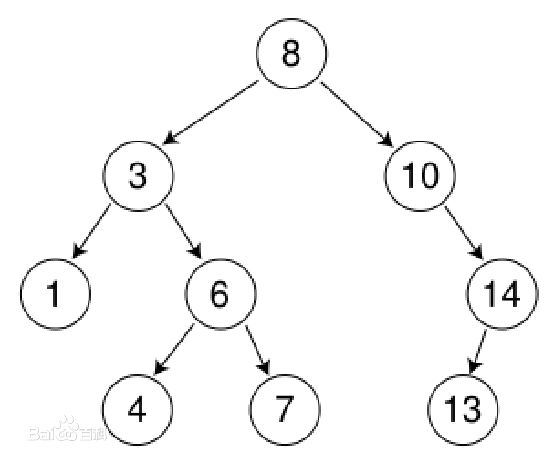int count = 0;
TreeNode KthNode(TreeNode pRoot, int k)
{
if(pRoot != null) {
TreeNode leftNode = KthNode(pRoot.left, k);
if(leftNode != null)
return leftNode;
count++;
if(count == k)
return pRoot;
TreeNode rightNode = KthNode(pRoot.right, k);
if(rightNode != null)
return rightNode;
}
return null;
}

//栈的方式

    TreeNode KthNode(TreeNode pRoot, int k)
{
Stack<TreeNode> stack = new Stack<TreeNode>();
if(pRoot==null||k==0) return null;
int t=0;
while(pRoot!=null ||stack.size()>0){
while(pRoot!=null){
stack.push(pRoot);
pRoot = pRoot.left;
}
if(stack.size()>0){
pRoot= stack.pop();
t++;
if(t==k) return pRoot;
pRoot= pRoot.right;
}
}
return null;
}

2.0 从上往下打印二叉树

从上往下打印出二叉树的每个节点，同层节点从左至右打印。

思路：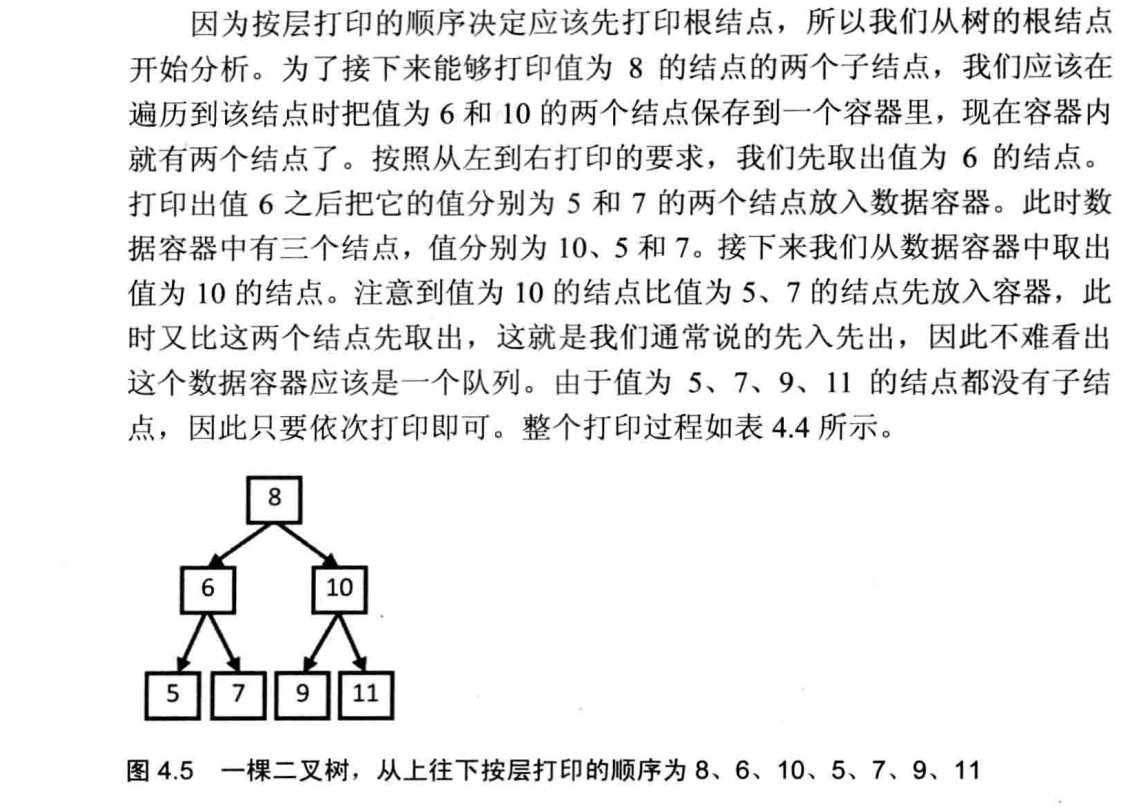import java.util.ArrayList;
/**
public class TreeNode {
int val = 0;
TreeNode left = null;
TreeNode right = null;

public TreeNode(int val) {
this.val = val;

}

}
*/
public class Solution {
/**
* 算法思路，（剑指offer图片）
* 1.根节点放到队列里面，队列不空，就打印队列头，打印这个节点，马上把这个节点的左右子节点放到队列中。
* 2.再要访问一个节点，把这个节点的左右放入，此时队头是同层的，对位是打印出来的左右。依次先入先出就可以得到结果。
* @param root
* @return
*/
public ArrayList<Integer> PrintFromTopToBottom(TreeNode root) {
ArrayList<Integer> layerList = new ArrayList<Integer>();
if (root == null)
return layerList;
while (!queue.isEmpty()) {
TreeNode node = queue.poll();
if (node.left != null)
if (node.right != null)
}
return layerList;
}

}

2.1二叉树打印成多行

从上到下按层打印二叉树，同一层结点从左至右输出。每一层输出一行。（注意是一行一行输出）

思路：主要采用左程云的思路，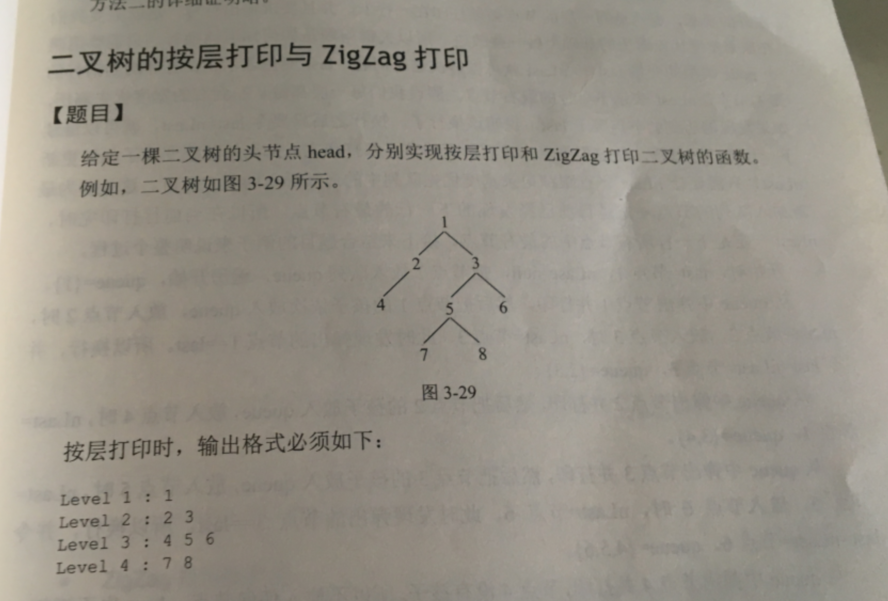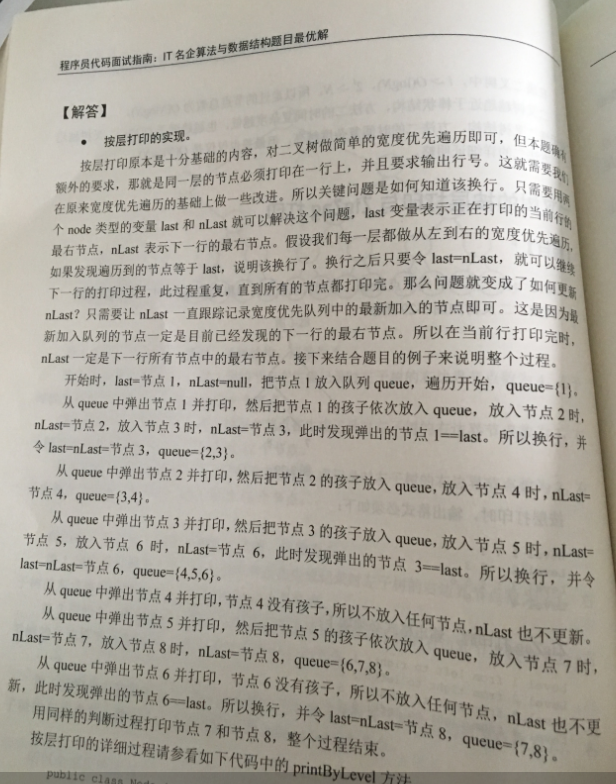static ArrayList<ArrayList<Integer> > Print(TreeNode pRoot) {
ArrayList<ArrayList<Integer>> res = new ArrayList<>();
if(pRoot == null)
return res;
TreeNode last = pRoot;
TreeNode nlast =null;
queue.offer(pRoot);
ArrayList<Integer> tmp = new ArrayList<>();
while (!queue.isEmpty()) {
pRoot = queue.poll();
//此时，下一层的最右要跟着更新
if (pRoot.left!=null) {
queue.offer(pRoot.left);
nlast = pRoot.left;
}
if (pRoot.right!=null) {
queue.offer(pRoot.right);
nlast = pRoot.right;
}
//如果到了本层的最右，就把这一层结果放入。注意最后一层时，isempty不成立，
//最后一层的结果要单独放入。
if (pRoot == last && !queue.isEmpty()) {
last = nlast;
tmp.clear();
}
}
return res;
}

2.2按之字形顺序打印二叉树

题目描述

请实现一个函数按照之字形打印二叉树，即第一行按照从左到右的顺序打印，第二层按照从右至左的顺序打印，第三行按照从左到右的顺序打印，其他行以此类推。

利用两个栈的辅助空间分别存储奇数偶数层的节点，然后打印输出。或使用链表的辅助空间来实现，利用链表的反向迭实现逆序输出。

import java.util.ArrayList;
import java.util.Stack;
/*
public class TreeNode {
int val = 0;
TreeNode left = null;
TreeNode right = null;

public TreeNode(int val) {
this.val = val;

}

}
*/
public class Solution {
//利用两个栈的辅助空间分别存储奇数偶数层的节点，然后打印输出。或使用链表的辅助空间来实现，利用链表的反向迭实现逆序输出。
public ArrayList<ArrayList<Integer> > Print(TreeNode pRoot) {
ArrayList<ArrayList<Integer>> res = new ArrayList<>();
if(pRoot == null)
return res;
Stack<TreeNode> s1 = new Stack<>();
Stack<TreeNode> s2 = new Stack<>();
s1.push(pRoot);
int level = 1;
while (!s1.empty()||!s2.empty()) {
if (level %2 != 0) {
ArrayList<Integer> list = new ArrayList<>();
while (!s1.empty()) {
TreeNode node = s1.pop();
if (node!= null) {
s2.push(node.left);//因为偶数层，先右后左，所以要先放左子树，栈
s2.push(node.right);

}
}
if (!list.isEmpty()) {
level++;
}
}
else {
ArrayList<Integer> list = new ArrayList<>();
while (!s2.empty()) {
TreeNode node = s2.pop();
if (node!= null) {
s1.push(node.right);
s1.push(node.left);

}
}
if (!list.isEmpty()) {
level++;
}
}
}
return res;
}

}

3.数据流中位数

如何得到一个数据流中的中位数？如果从数据流中读出奇数个数值，那么中位数就是所有数值排序之后位于中间的数值。如果从数据流中读出偶数个数值，那么中位数就是所有数值排序之后中间两个数的平均值。

思路：主要是博客的代码，参考了左的部分分析。

创建优先级队列维护大顶堆和小顶堆两个堆，并且小顶堆的值都大于大顶堆的值。比如6，1，3，0，9，8，7，2则较小的部分大根堆是0，1，2，3 较大的部分小根堆是6，7，8，9.

具体思路：

1.本代码为了保证两个堆的尺寸差距最大为1，采用奇数个时候插到大根堆，偶数个插到小根堆。

2.当数据总数为偶数时，新加入的元素，应当进入小根堆（注意不是直接进入小根堆，而是经大根堆筛选后取大根堆中最大元素进入小根堆），要保证小根堆里面所有数都比大根堆的大。

3.当数据为奇数个时，按照相应的调整进入大根堆。

4.如果个数位奇数个，则大根堆堆顶为中位数，否则就是两个堆顶除以2.比如新加入三个，那么第一个在大，第二个在小，第三个可能在大。所以就是大根堆的堆顶。

* 插入有两种思路： 左采用第一种，本代码采用第二种

* 1：直接插入大堆中，之后若两堆尺寸之差大于1(也就是2)，则从大堆中弹出堆顶元素并插入到小堆中

* 若两队之差不大于1，则直接插入大堆中即可。

* 2：奇数个数插入到大堆中，偶数个数插入到小堆中，

* 但是 可能会出现当前待插入的数比小堆堆顶元素大，此时需要将元素先插入到小堆，然后将小堆堆顶元素弹出并插入到大堆中

* 对于偶数时插入小堆的情况，一样的道理。why?

* 因为要保证最大堆的元素要比最小堆的元素都要小。

import java.util.Comparator;
import java.util.PriorityQueue;

public class Shujuliumedian {
int count = 0;
PriorityQueue<Integer> minheap = new PriorityQueue<>();
PriorityQueue<Integer> maxheap = new PriorityQueue<>(11, new Comparator<Integer>() {

@Override
public int compare(Integer o1, Integer o2) {
// TODO Auto-generated method stub

return o2.compareTo(o1);//o2大于o1返回1 ，否则返回-1
}
});
public void Insert(Integer num) {
count++;
if (count % 2 ==0) {//偶数进入小根堆,这个其实无所谓，定了一个平均分配的规则
//保证进入小根堆的元素要比大根堆最大的大，所以如果小调整
if (!maxheap.isEmpty() && num < maxheap.peek()) {
maxheap.offer(num);
num = maxheap.poll();
}
minheap.offer(num);
}
else {//奇数进入大根堆
if (!minheap.isEmpty() && num > minheap.peek()) {
minheap.offer(num);
num = minheap.poll();
}
maxheap.offer(num);
}
}

public Double GetMedian() {
double median = 0;
if (count % 2 ==1) {
median = maxheap.peek();
}
else
median = (minheap.peek()+maxheap.peek())/2.0;
return median;
}
}


4.二叉树中和为某一值的路径

输入一颗二叉树的跟节点和一个整数，打印出二叉树中结点值的和为输入整数的所有路径。路径定义为从树的根结点开始往下一直到叶结点所经过的结点形成一条路径。(注意: 在返回值的list中，数组长度大的数组靠前)

思路：     * 剑指offer思路 博客代码
* 代码步骤：一个链表记录路径，一个存放这个链表的链表记录最终的结果。
* 1.首先将根节点放入链表，target减去这个根节点
* 2.判断是否target同时是叶子节点，如果是就将当前的链表放在结果连表里
* 3.如果不是，就递归去访问左右子节点。

* 4.无论是找到没有，都要回退一步、

import java.util.ArrayList;
/**
public class TreeNode {
int val = 0;
TreeNode left = null;
TreeNode right = null;

public TreeNode(int val) {
this.val = val;

}

}
*/
public class Solution {
private ArrayList<ArrayList<Integer>> listAll = new ArrayList<ArrayList<Integer>>();

private ArrayList<Integer> list =new ArrayList<Integer>();
private ArrayList<ArrayList<Integer>> resultList = new ArrayList<ArrayList<Integer>>();
/**
* 剑指offer思路
* 代码步骤：一个链表记录路径，一个存放这个链表的链表记录最终的结果。前序遍历去访问。先访问根，在递归在左右子树找。注意回退
* 1.首先将根节点放入链表，target减去这个根节点
* 2.判断是否target同时是叶子节点，如果是就将当前的链表放在结果连表里
* 3.如果不是，就递归去访问左右子节点。
* 4.无论是找到没有，都要回退一步、
* @param root
* @param target
* @return
*/
public ArrayList<ArrayList<Integer>> FindPath(TreeNode root,int target) {
if(root == null)
return resultList;
target = target - root.val;
if(target == 0 && root.left == null && root.right == null){
}
else {
FindPath(root.left, target);
FindPath(root.right, target);

}
// 在返回父节点之前，在路径上删除该结点
list.remove(list.size()-1);
return resultList;
}

}

5.重建二叉树

输入某二叉树的前序遍历和中序遍历的结果，请重建出该二叉树。假设输入的前序遍历和中序遍历的结果中都不含重复的数字。例如输入前序遍历序列{1,2,4,7,3,5,6,8}和中序遍历序列{4,7,2,1,5,3,8,6}，则重建二叉树并返回。

思路：剑指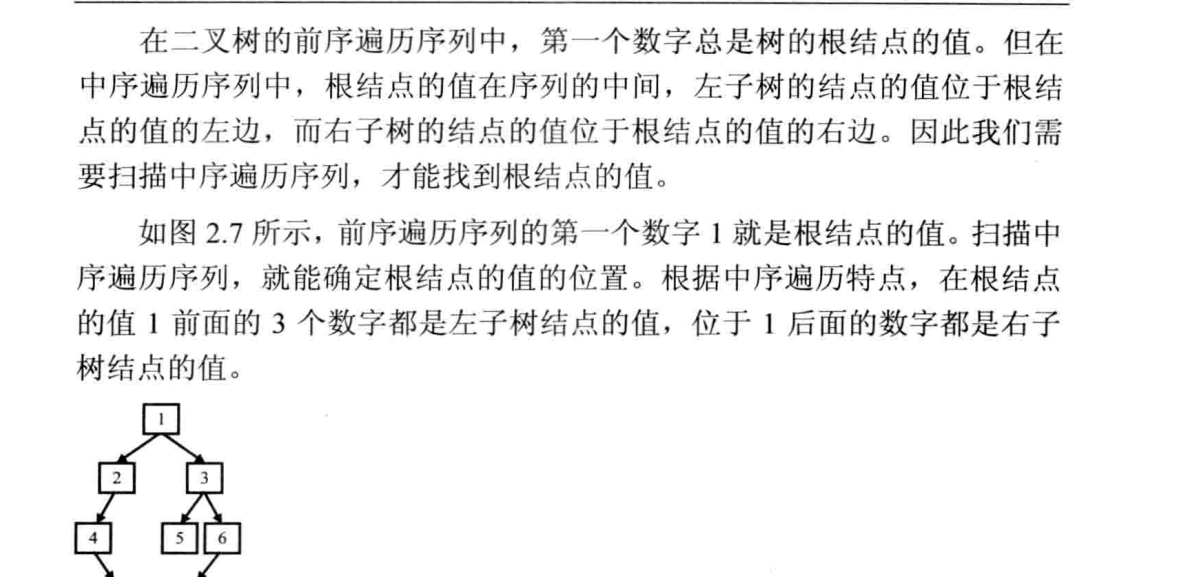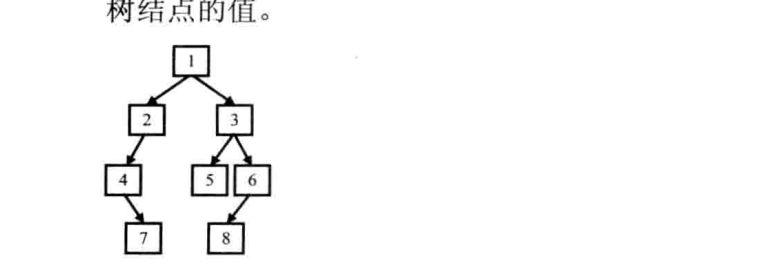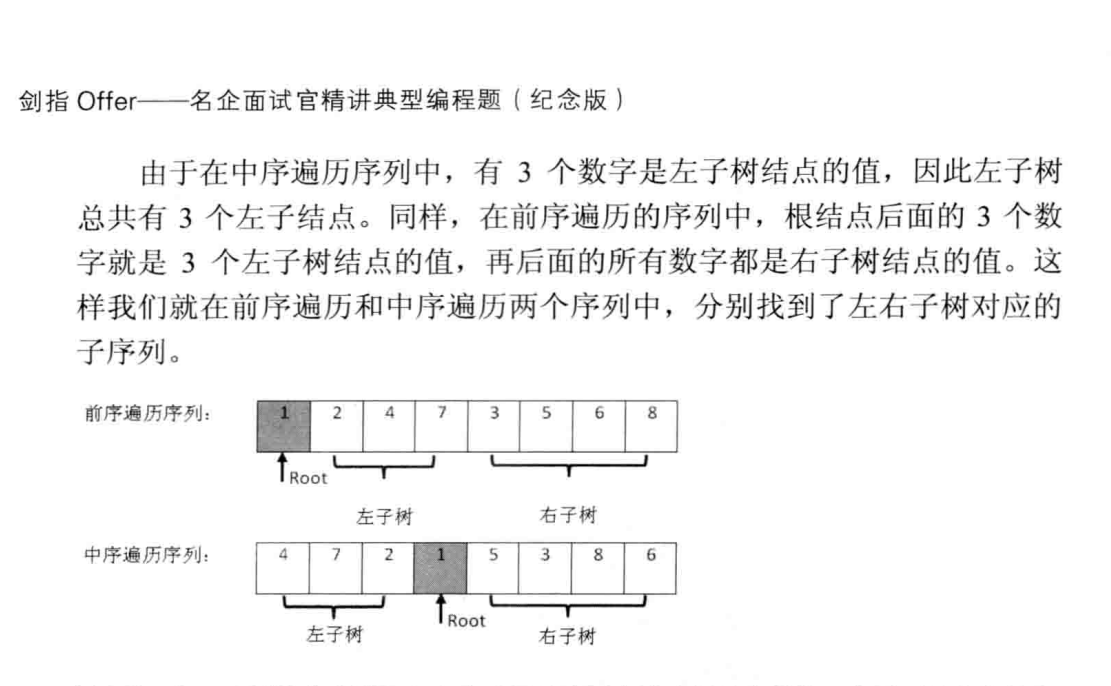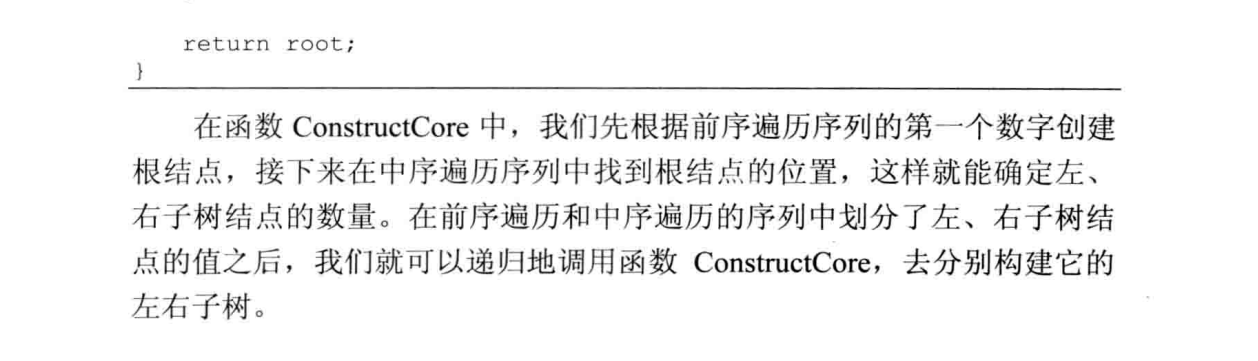/**
* Definition for binary tree
* public class TreeNode {
*     int val;
*     TreeNode left;
*     TreeNode right;
*     TreeNode(int x) { val = x; }
* }
*/
import java.util.Arrays;

public class Solution {
public TreeNode reConstructBinaryTree(int[] pre, int[] in) {
if (pre == null || in == null) {
return null;
}
if (pre.length == 0 || in.length == 0) {
return null;
}
if (pre.length != in.length) {
return null;
}
TreeNode root = new TreeNode(pre);//第一个
for (int i = 0; i < in.length; i++) {
if (pre == in[i]) {
//pre的0往后数i个是左子树的，copyofrange包含前面的下标，不包含后面的下标
//in的i往前数i个是左子树的。
root.left = reConstructBinaryTree(Arrays.copyOfRange(pre, 1, i + 1), Arrays.copyOfRange(in, 0, i));
//注意in是从i+1开始，因为i是现在的根，i+1开始才是右子树
root.right = reConstructBinaryTree(Arrays.copyOfRange(pre, i + 1, pre.length),
Arrays.copyOfRange(in, i + 1, in.length));
}
}
return root;
}
}

6.树的子结构

输入两棵二叉树A，B，判断B是不是A的子结构。（ps：我们约定空树不是任意一个树的子结构）

思路：   //先从根开始再把左作为根，再把右作为根由本函数决定。把一个为根的时候的具体比对过程是第二个函数决定。
//从根可以认为是一颗树，从左子树开始又可以认为是另外一颗树，从右子树开始又是另外一棵树。
//本函数就是判断这一整颗树包不包含树2，如果从根开始的不包含，就从左子树作为根节点开始判断，
//再不包含从右子树作为根节点开始判断。
//是整体算法递归流程控制。

/**
public class TreeNode {
int val = 0;
TreeNode left = null;
TreeNode right = null;

public TreeNode(int val) {
this.val = val;

}

}
*/
public class Solution {
//先从根开始再把左作为根，再把右作为根由本函数决定。把一个为根的时候的具体比对过程是第二个函数决定。
//从根可以认为是一颗树，从左子树开始又可以认为是另外一颗树，从右子树开始又是另外一棵树。
//本函数就是判断这一整颗树包不包含树2，如果从根开始的不包含，就从左子树作为根节点开始判断，
//再不包含从右子树作为根节点开始判断。
//是整体算法递归流程控制。
public boolean HasSubtree(TreeNode root1,TreeNode root2) {
boolean res = false;
if (root1 != null && root2 != null) {
if(root1.val == root2.val){
res = doesTree1haveTree2(root1,root2);
}
if(!res)
{
res = HasSubtree(root1.left, root2);
}
if(!res)
{
res = HasSubtree(root1.right, root2);
}
}
return res;
}
//本函数，判断从当前的节点 ，开始两个树能不能对应上，是具体的比对过程
public boolean doesTree1haveTree2(TreeNode root1,TreeNode root2) {
if(root2 == null)
return true;
if(root1 == null)
return false;
if(root1.val != root2.val){
return false;
}
//如果根节点可以对应上，那么就去分别比对左子树和右子树是否对应上
return doesTree1haveTree2(root1.left, root2.left) && doesTree1haveTree2(root1.right, root2.right);
}
}

7.二叉树的镜像

操作给定的二叉树，将其变换为源二叉树的镜像。

二叉树的镜像定义：源二叉树
8
/  \
6   10
/ \  / \
5  7 9 11
镜像二叉树
8
/  \
10   6
/ \  / \
11 9 7  5

/**
public class TreeNode {
int val = 0;
TreeNode left = null;
TreeNode right = null;

public TreeNode(int val) {
this.val = val;

}

}
*/
import java.util.Stack;
public class Solution {
/**
* 算法步骤
* 1.节点为空直接返回
* 2.如果这个节点的左右子树不为空，就交换。
* 3.递归对这个节点的左子树进行求镜像。对这个节点的右子树求镜像。
* @param root
*/
public void Mirror(TreeNode root){
if (root == null) {
return;
}
if(root.left != null || root.right != null) {
TreeNode temp = root.left;
root.left = root.right;
root.right = temp;
Mirror(root.left);
Mirror(root.right);
}

}
/*public void Mirror(TreeNode root) {
if (root == null) {
return;
}
Stack<TreeNode> stack = new Stack<TreeNode>();
stack.push(root);
while (!stack.isEmpty()) {
TreeNode node = stack.pop();
if (node.left != null || node.right != null) {
TreeNode temp = node.left;
node.left = node.right;
node.right = temp;
}
if(node.left != null)
stack.push(node.left);
if(node.right != null)
stack.push(node.right);

}
}*/
}

8、二叉搜素树的后序遍历序列

输入一个整数数组，判断该数组是不是某二叉搜索树的后序遍历的结果。如果是则输出Yes,否则输出No。假设输入的数组的任意两个数字都互不相同。

public class Solution {
/**二叉搜索树的性质：
* 所有左子树的节点小于根节点，所有右子树的节点值大于根节点的值。
* 算法步骤：
* 1.后序遍历的最后一个值为root，在前面的数组中找到第一个大于root值的位置。
* 2.这个位置的前面是root的左子树，右边是右子树。然后左右子树分别进行这个递归操作。
* 3.其中，如果右边子树中有比root值小的直接返回false
* @param sequence
* @return
*/
public boolean VerifySquenceOfBST(int [] sequence) {
if (sequence == null || sequence.length == 0)
return false;
return IsBST(sequence, 0, sequence.length -1);

}

public boolean IsBST(int [] sequence, int start, int end) {
if(start >= end) //注意这个条件的添加// 如果对应要处理的数据只有一个或者已经没
//有数据要处理（start>end）就返回true
return true;
int index = start;
for (; index < end; index++) {//寻找大于root的第一个节点，然后再分左右两部分
if(sequence[index] > sequence[end])
break;
}
for (int i = index; i < end; i++) {//若右子树有小于根节点的值，直接返回false
if (sequence[i] < sequence[end]) {
return false;
}
}
return IsBST(sequence, start, index-1) && IsBST(sequence, index, end-1);
}

}/*当案例为{4,6,7,5}的时候就可以看到：
（此时start为0，end为3）
一开始index处的值为1，左边4的是start为0，index-1为0，下一次递归的start和end是一样的，true！
右边，start为1，end-1为2，是{6,7}元素，下一轮递归是：
————7为root，index的值指向7，
————所以左边为6，start和index-1都指向6，返回true。
————右边index指向7，end-1指向6，这时候end > start！如果这部分还不返回true，下面的数组肯定超了    */


9、二叉搜索树与双向链表

题目描述

输入一棵二叉搜索树，将该二叉搜索树转换成一个排序的双向链表。要求不能创建任何新的结点，只能调整树中结点指针的指向。

/**
public class TreeNode {
int val = 0;
TreeNode left = null;
TreeNode right = null;

public TreeNode(int val) {
this.val = val;

}

}
*/
public class Solution {
/**二叉搜索树的中序遍历就是递增的排序，所以就运用中序遍历方法来做。
* 算法思想：
* 中序遍历的步骤，只不过在递归的中间部分不是输出节点值，而是调整指针指向。
*     10
*     /\
*    5 12
*   /\
*  4 7
* @param pRootOfTree
* @return
*/
public TreeNode Convert(TreeNode pRootOfTree) {
ConvertSub(pRootOfTree);
}
public void ConvertSub(TreeNode pRootOfTree) {
if(pRootOfTree == null)
return;
ConvertSub(pRootOfTree.left);
}
else {
}
ConvertSub(pRootOfTree.right);
}
}

10、二叉树的深度

题目描述

输入一棵二叉树，求该树的深度。从根结点到叶结点依次经过的结点（含根、叶结点）形成树的一条路径，最长路径的长度为树的深度。

/**
public class TreeNode {
int val = 0;
TreeNode left = null;
TreeNode right = null;

public TreeNode(int val) {
this.val = val;

}

}
*/
public class Solution {
// 注意最后加1，因为左右子树的深度大的+根节点的深度1
public int TreeDepth(TreeNode root) {
if(root == null)
return 0;
int left = TreeDepth(root.left);
int right = TreeDepth(root.right);
return left > right? left +1:right+1;
}
}

11、平衡二叉树

输入一棵二叉树，判断该二叉树是否是平衡二叉树

描述：如果某二叉树中任意节点的左右子树的深度相差不超过1，那么它就是一棵平衡二叉树。

public class Solution {
// 注意使用全局变量
boolean isBalance = true;
public boolean IsBalanced_Solution(TreeNode root) {
lengthOfTree(root);
return isBalance;
}

private int lengthOfTree(TreeNode root) {
if (root == null)
return 0;
int left = lengthOfTree(root.left);
int right = lengthOfTree(root.right);
if (Math.abs(left - right) > 1)
isBalance = false;
return Math.max(left, right) + 1;

}
}

第二种Better思路：从底向上判断，这样可以记录下一层的深度

public class Solution {
public boolean IsBalanced(TreeNode root) {
int depth = 0;
return IsBalanced(root, depth);
}

public boolean IsBalanced(TreeNode root, int depth) {
if (root == null) {
depth = 0;
return true;
}

int left = 0, right = 0;
if (IsBalanced(root.left, left) && IsBalanced(root.right, right)) {
int diff = left - right;
if (diff <= 1 && diff >= -1) {
depth = 1 + (left > right ? left : right);
return true;
}
}

return false;
}
}

12、二叉树的下一个节点

题目描述

给定一个二叉树和其中的一个结点，请找出中序遍历顺序的下一个结点并且返回。注意，树中的结点不仅包含左右子结点，同时包含指向父结点的指针。

/*
int val;

this.val = val;
}
}
*/
public class Solution {
/**参考左程云和有详解博客的思路，
* 主要分三种：
* 1.如果有右孩子，后继节点就是最左边的
* 2.如果没有右孩子，判断是否是父节点的左孩子，是的话，返回，不是继续网上找
* 3.找不到就是null
* @param pNode
* @return
*/
{
if(pNode == null)
return null;
// 如果有右子树，则找右子树的最左节点
if (pNode.right != null) {
pNode = pNode.right;
// 如果此时pNode没有左子树，那么它就是下一个结点 ，就是最左边的了
//如果有左子树，那就在左子树找最左边的
while(pNode.left != null)
pNode = pNode.left;
return pNode;

}
非跟结点，并且没有右子树
while(pNode.next != null) {
// 找到一个结点是该其父亲的左孩子  ,找到就是返回父节点作为后记
if (pNode.next.left == pNode)
return pNode.next;
//找不到这个左孩子的，就继续往上，next其实是parent
pNode = pNode.next;
}
return null;
}
}

13、对称的二叉树

请实现一个函数，用来判断一颗二叉树是不是对称的。注意，如果一个二叉树同此二叉树的镜像是同样的，定义其为对称的。

/*
public class TreeNode {
int val = 0;
TreeNode left = null;
TreeNode right = null;

public TreeNode(int val) {
this.val = val;

}

}
*/
public class Solution {
//利用递归进行判断，
//若左子树的左孩子等于右子树的右孩子且左子树的右孩子等于右子树的左孩子，
//并且左右子树节点的值相等，则是对称的。
boolean isSymmetrical(TreeNode pRoot)
{
if (pRoot == null)
return true;
return isCommon(pRoot.left,pRoot.right);
}
public boolean isCommon(TreeNode leftNode, TreeNode rightNode) {
if (leftNode == null && rightNode == null)
return true;
if (leftNode != null && rightNode != null) {
return leftNode.val == rightNode.val &&
isCommon(leftNode.left, rightNode.right) &&
isCommon(leftNode.right, rightNode.left);
}
return false;
}
}

14、序列化二叉树

请实现两个函数，分别用来序列化和反序列化二叉树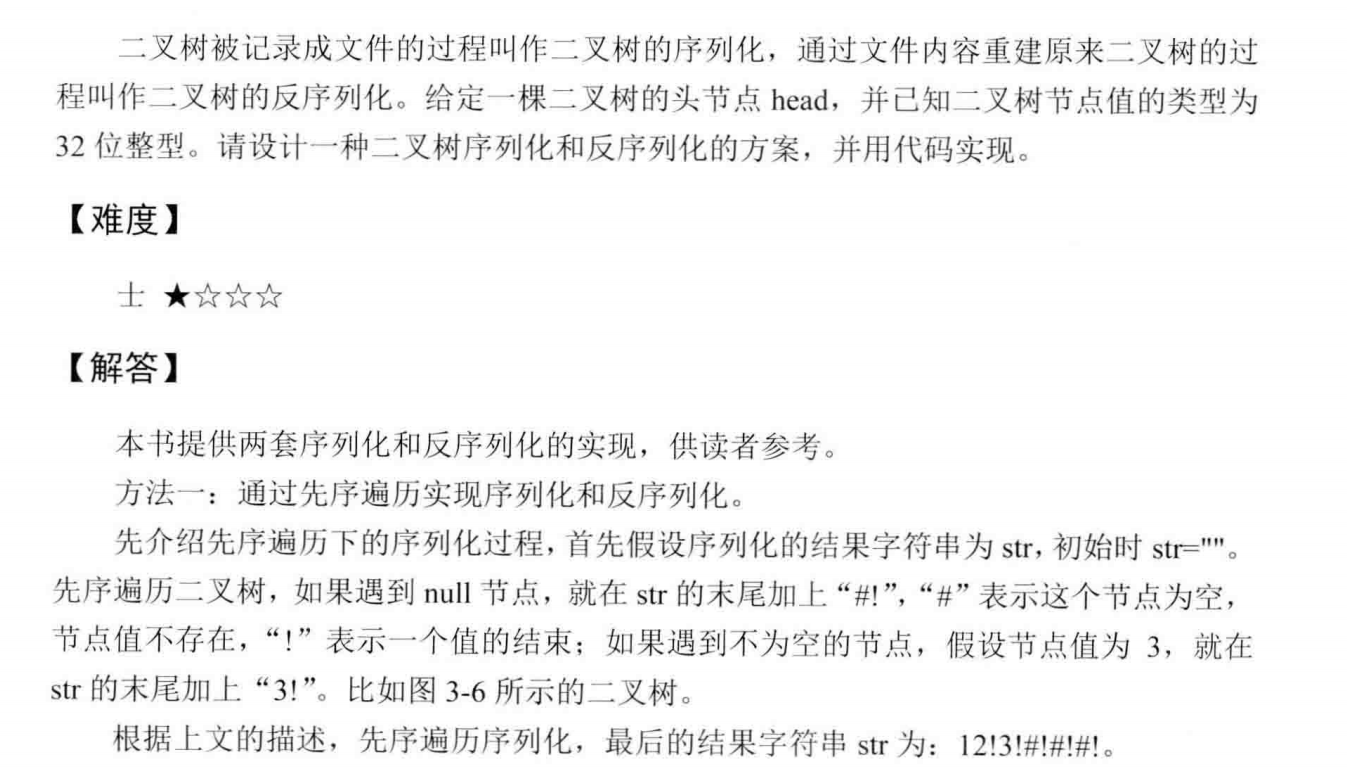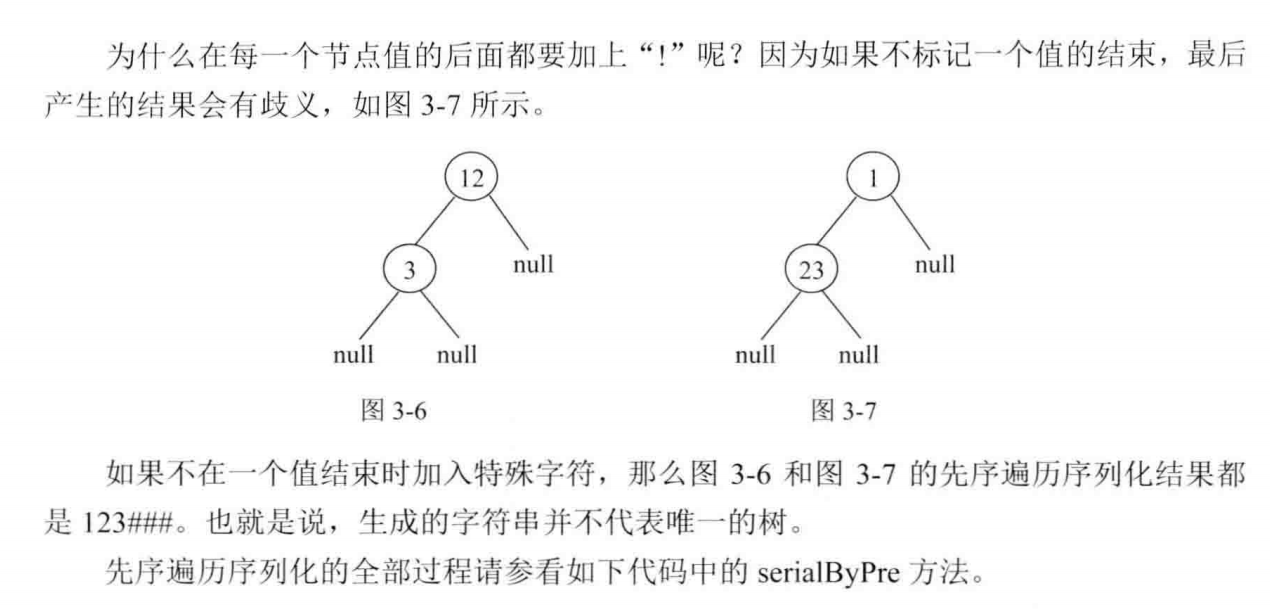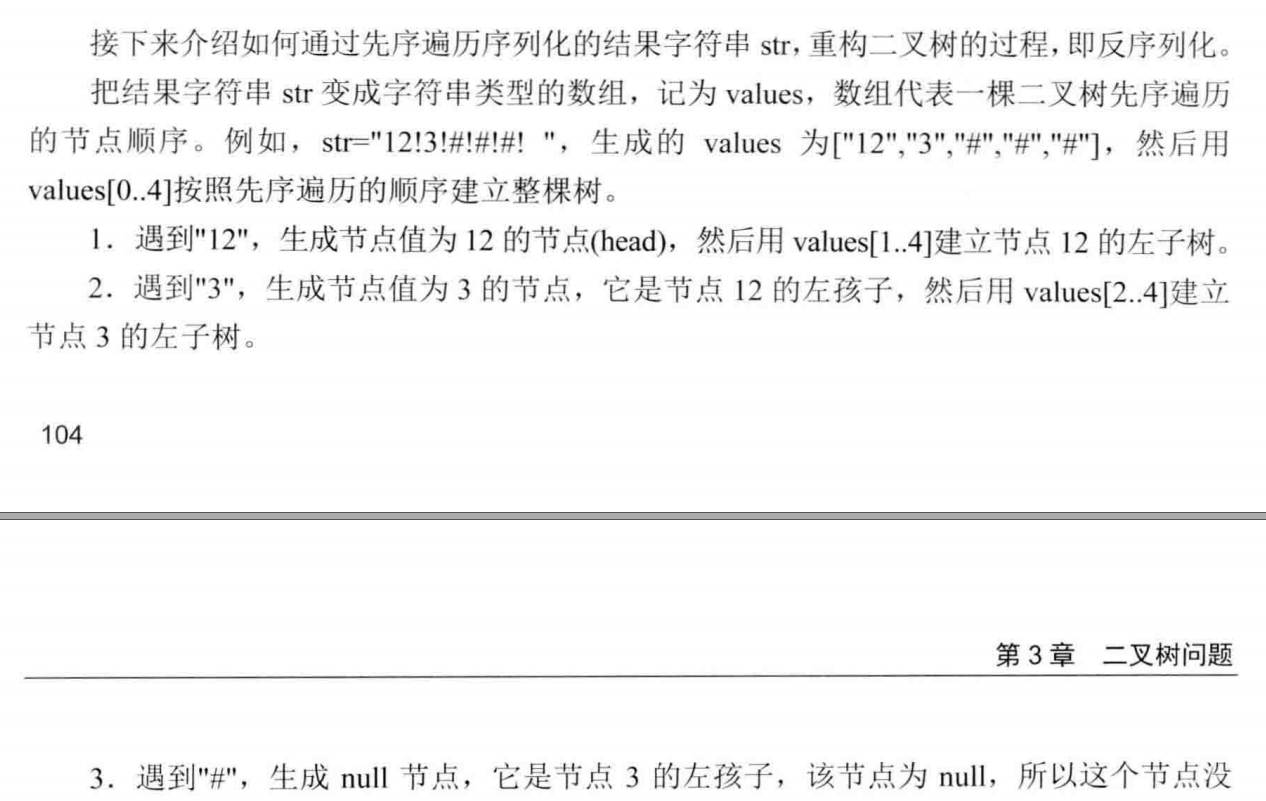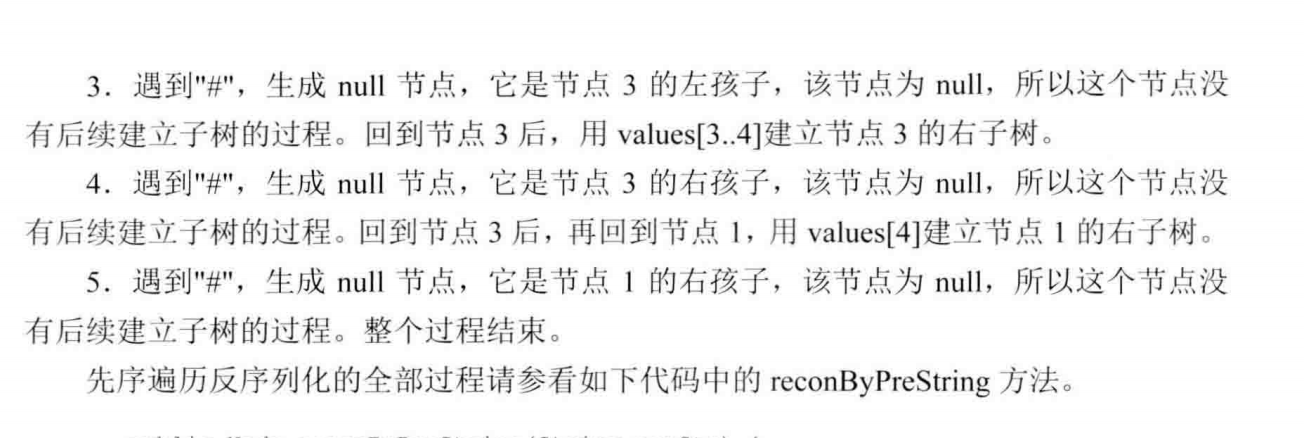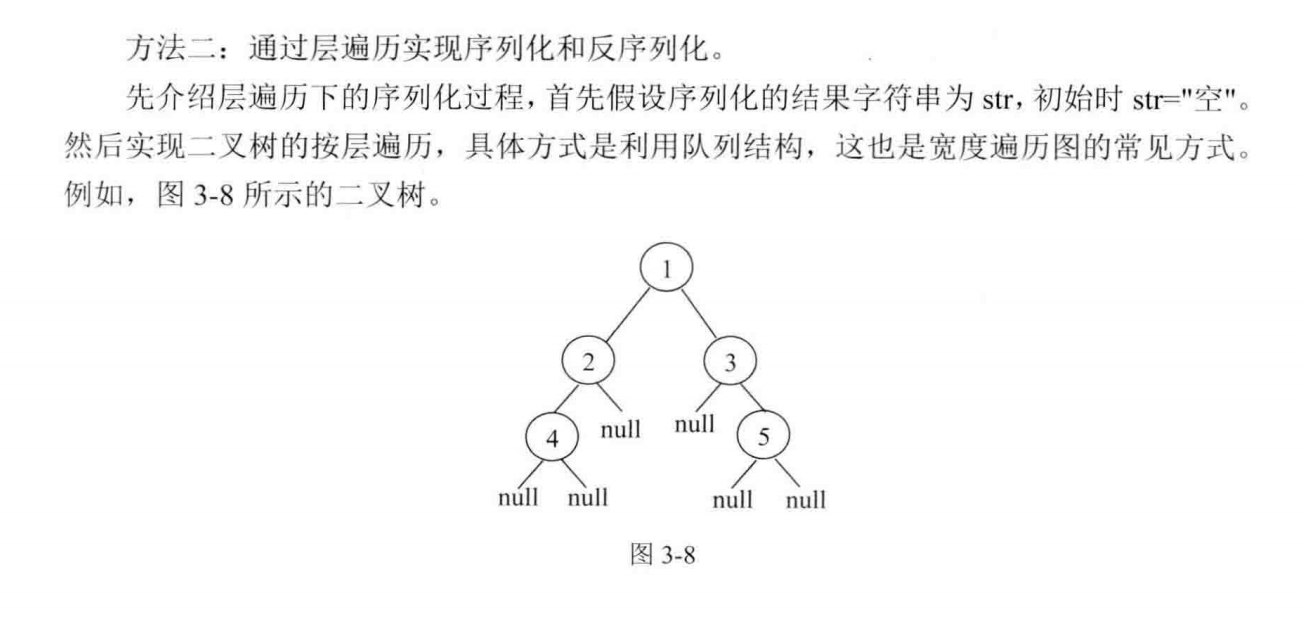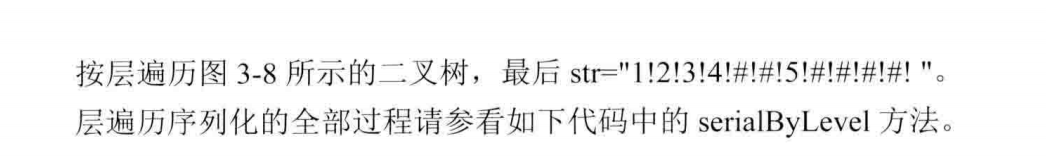这段代码是按照先序遍历的方法来做的：

/*
public class TreeNode {
int val = 0;
TreeNode left = null;
TreeNode right = null;

public TreeNode(int val) {
this.val = val;

}

}
*/
import java.util.Queue;
public class Solution {
//主要运用左程云的编程思想的方式来实现
String Serialize(TreeNode root) {
if(root == null)
return "#!";
String res = root.val+"!";
res = res + Serialize(root.left);
res = res + Serialize(root.right);
return res;
}

TreeNode Deserialize(String str) {
String [] values = str.split("!");
for (int i = 0; i < values.length; i++) {
queue.offer(values[i]);
}
return reconPre(queue);
}
TreeNode reconPre(Queue<String> queue) {
String value = queue.poll();
if(value.equals("#"))
return null;
}
}

三、字符串

1.正则表达式的匹配

请实现一个函数用来匹配包括'.'和'*'的正则表达式。模式中的字符'.'表示任意一个字符，而'*'表示它前面的字符可以出现任意次（包含0次）。 在本题中，匹配是指字符串的所有字符匹配整个模式。例如，字符串"aaa"与模式"a.a"和"ab*ac*a"匹配，但是与"aa.a"和"ab*a"均不匹配。

思路：参考网上的思路，主要就是分程序中的两种情况来讨论。第二位是不是*

/*
当模式中的第二个字符不是“*”时：
1、如果字符串第一个字符和模式中的第一个字符相匹配，
那么字符串和模式都后移一个字符，然后匹配剩余的。
2、如果字符串第一个字符和模式中的第一个字符相不匹配，直接返回false。
而当模式中的第二个字符是“*”时：
如果字符串第一个字符跟模式第一个字符不匹配，则模式后移2个字符，继续匹配。
如果字符串第一个字符跟模式第一个字符匹配，可以有3种匹配方式：
1、模式后移2字符，相当于x*被忽略；
2、字符串后移1字符，模式后移2字符； 相当于x*算一次
3、字符串后移1字符，模式不变，即继续匹配字符下一位，因为*可以匹配多位，相当于算多次
这里需要注意的是：Java里，要时刻检验数组是否越界。*/
public class Zhengze {
public boolean match(char[] str, char[] pattern) {
if (str == null || pattern == null) {
return false;
}
int strIndex = 0;
int patternIndex = 0;
return matchCore(str, strIndex, pattern, patternIndex);
}

public boolean matchCore(char[] str, int strIndex, char[] pattern, int patternIndex) {
// 有效性检验：str到尾，pattern到尾，匹配成功
if (strIndex == str.length && patternIndex == pattern.length)
return true;
// pattern先到尾，匹配失败
if (strIndex != str.length && patternIndex == pattern.length)
return false;
// 模式第2个是*，且字符串第1个跟模式第1个匹配,分3种匹配模式；如不匹配，模式后移2位
if (patternIndex + 1 < pattern.length && pattern[patternIndex + 1] == '*') {
if ((strIndex != str.length && pattern[patternIndex] == str[strIndex])
|| (pattern[patternIndex] == '.' && strIndex != str.length)) {
return // 模式后移2，视为x*匹配0个字符
matchCore(str, strIndex, pattern, patternIndex + 2)
// 视为模式匹配1个字符
|| matchCore(str, strIndex + 1, pattern, patternIndex + 2)
// *匹配1个，再匹配str中的下一个
|| matchCore(str, strIndex + 1, pattern, patternIndex);

} else {
return matchCore(str, strIndex, pattern, patternIndex + 2);
}
} // 模式第2个不是*，且字符串第1个跟模式第1个匹配，则都后移1位，否则直接返回false
if ((strIndex != str.length && pattern[patternIndex] == str[strIndex])
|| (pattern[patternIndex] == '.' && strIndex != str.length)) {
return matchCore(str, strIndex + 1, pattern, patternIndex + 1);
}
return false;
}
}


2.表示数值的字符串

请实现一个函数用来判断字符串是否表示数值（包括整数和小数）。例如，字符串"+100","5e2","-123","3.1416"和"-1E-16"都表示数值。 但是"12e","1a3.14","1.2.3","+-5"和"12e+4.3"都不是。

思路:按照一定的规则，如果第一位是+或-，就后移一位。

如果是数字，索引后移，数字表示1.

如果是点，要判断至此点的数量和e的数量是否已经有了，因为java 中e要求后面为整数，如果有了肯定false。索引后移，dotnum增加。

如果是e，判断是否重复e，或者前面没有数字返回false。enum++， 索引++，此时还要判断最后一位是不是e或者+或者-，如果是false。

public class StrexpressNum {
/*例子：
* 110   1a1   1.1.1  2.2  12e
*
* */
public  static boolean isNumeric(char[] str) {
if(str == null)
return false;
int length = str.length;
int dotNum = 0;//记录点的数量
int index = 0;//索引
int eNum = 0;//记录e的数量
int num = 0;//记录数字的数量
if (str == '+' || str == '-') {
index++;
}
while (index < length) {
if(str[index]>='0' && str[index]<='9') {
index++;
num = 1;
// .前面可以没有数字,所以不需要判断num是否为0
}else if(str[index]=='.') {
// e后面不能有.,e的个数不能大于1.java科学计数要求aeb，b为整数
if(dotNum >0 || eNum >0)
return false;
dotNum++;
index++;
}else if(str[index] == 'e' || str[index] == 'E') {
// 重复e或者e前面没有数字
if(eNum > 0 || num ==0)
return false;
eNum++;
index++;
// 符号不能在最后一位
if(index < length &&(str[index]=='+'||str[index]=='-'))
index++;
// 表示e或者符号在最后一位
if(index == length)
return false;
}else {
return false;
}

}
return true;
}
public static void main(String[] args) {
char [] str = {'1','2','e'};
System.out.println(isNumeric(str));

}
}

或者用正则表达式来匹配：

[+-]? 表示+或者-出现0次或1次。[0-9]{0,}表示0到9出现0次或者更多次。()表示这个分组作为一个整体。 \\.?表示.出现0次或1次。[0-9]{1,}表示0到9出现1次或者多次。()表示一个分组。如果把两个分组去掉进行判断是不准确的。100匹配到[0-9]{1,}出错。

    public boolean isNumeric(char[] str) {
String res = String.valueOf(str);
return res.matches("[+-]?[0-9]{0,}(\\.?[0-9]{1,})?([Ee][+-]?[0-9]{1,})?");
}

3.0第一个只出现一次的字符

题目描述

在一个字符串(0<=字符串长度<=10000，全部由字母组成)中找到第一个只出现一次的字符,并返回它的位置, 如果没有则返回 -1（需要区分大小写）.

import java.util.LinkedHashMap;
public class Solution {
public int FirstNotRepeatingChar(String str) {
//这个hashmap有序，所以用这个
//遍历字符串，第一次设为1 否则就加
for (int i = 0; i < str.length(); i++) {
if (!map.containsKey(str.charAt(i))) {
map.put(str.charAt(i), 1);
}
else
map.put(str.charAt(i),map.get(str.charAt(i))+1);
}
//找出现次数为1的
for (int i = 0; i < str.length(); i++) {
if (map.get(str.charAt(i)) == 1) {
return i;
}
}
return -1;
}
}

3.1字符流中第一个不重复的字符

思路：参考两个博客一种用hashmap来做，一种用字符数组来做。

hashmap方法：

    HashMap<Character, Integer> map = new HashMap<>();//记录字符出现次数
ArrayList<Character> list = new ArrayList<>();//记录当前的所有的字符
//Insert one char from stringstream
public void Insert(char ch)
{
if(map.containsKey(ch))
map.put(ch, map.get(ch)+1);
else
map.put(ch,1);
}
//return the first appearence once char in current stringstream
public char FirstAppearingOnce()
{
for(char c:list) {
if(map.get(c)==1)
return c;
}
return '#';
}

字符数组的方法：

char类型和int类型数值在 0-255之内的可以通用

默认初始值是ASCII的0（int）;char类型会自动转换成(int型)ASCII进行算术运算

   char [] chars = new char;//ascii字符共128，其他字符非中文认为256个，
//为每个字符预留空间。默认每个存的ascii值为0
StringBuffer sb = new StringBuffer();//记录当前的所有字符
//Insert one char from stringstream
public void Insert(char ch)
{
sb.append(ch);
chars[ch]++;//如果字符是1，那么就是在字符1对应的下标的地方
//也就是49的下标处，ascii加1.此时如果输出chars[ch],里面存ascii值
//为1，所以是一个不可显示的字符。
}
//return the first appearence once char in current stringstream
public char FirstAppearingOnce()
{
char [] str = sb.toString().toCharArray();
for(char c:str) {
if(chars[c] == 1)//判断这个字符数组中在这个字符下标处值是否为1.
return c;
}
return '#';
}

4.翻转字符串

牛客最近来了一个新员工Fish，每天早晨总是会拿着一本英文杂志，写些句子在本子上。同事Cat对Fish写的内容颇感兴趣，有一天他向Fish借来翻看，但却读不懂它的意思。例如，“student. a am I”。后来才意识到，这家伙原来把句子单词的顺序翻转了，正确的句子应该是“I am a student.”。Cat对一一的翻转这些单词顺序可不在行，你能帮助他么？

思路：两个思路，一个比较简单的就是用空格切分出来，student.  a am I。然后从后往前添加到stringbuffer里面。

另一个思路就是基本思路剑指offer，先将整个字符串翻转，然后将每个单词翻转。i love you  反转就是  uoy evol i,然后再每个单词进行反转。

public class Fanzhuan {
//第一种方法，用空格将字符串切分，
//倒着往stringbuffer里面插入。
public String ReverseSentence1(String str) {
if (str == null || str.trim().length() == 0) {
return str;
}
String[] strs =str.split(" ");//str = "i love you"则strs=i strs=love
StringBuffer sb = new StringBuffer();
for (int i = strs.length -1; i >= 0; i--) {
sb.append(strs[i]);
if (i>0) {//最后一个不添加空格
sb.append(" ");
}
}
return sb.toString();
}
//第二种思路，先将整个字符串反转，再逐个单词反转
public String ReverseSentence(String str) {
if (str == null || str.length() == 0)
return str;
if (str.trim().length() == 0)
return str;
StringBuilder sb = new StringBuilder();
String re = reverse(str);
String[] s = re.split(" ");
for (int i = 0; i < s.length - 1; i++) {
sb.append(reverse(s[i]) + " ");
}
sb.append(reverse(s[s.length-1]));
return String.valueOf(sb);
}

public String reverse(String str) {
StringBuilder sb = new StringBuilder();
for (int i = str.length() - 1; i >= 0 ; i--) {
sb.append(str.charAt(i));
}
return String.valueOf(sb);
}
}


5.左旋转字符串

汇编语言中有一种移位指令叫做循环左移（ROL），现在有个简单的任务，就是用字符串模拟这个指令的运算结果。对于一个给定的字符序列S，请你把其循环左移K位后的序列输出。例如，字符序列S=”abcXYZdef”,要求输出循环左移3位后的结果，即“XYZdefabc”。是不是很简单？OK，搞定它！

思路：前n位反转，后几位反转，最后总的反转

先反转前n位，再反转后几位，变为了cbafedZYX，再整体反转变为XYZdefabc

 public String LeftRotateString(String str,int n) {
if (str == null || str.trim().length() == 0) {
return str;
}
int len = str.length();
n = n % len;// 当len=3，n=4，其实相当于左旋转1位，所以需要取余
char[] charstr = str.toCharArray();
//先旋转前面的
reverse(charstr, 0, n-1);
//再旋转后面的字符串
reverse(charstr, n, len -1);
//最后整体反转
reverse(charstr, 0, len-1);
return String.valueOf(charstr);
}
//实现的是charstrs从i到j的反转，也可以使用上题中stringbuffer的反转方式
private void reverse(char[] charStrs, int i, int j) {
while(i<j) {
char temp = charStrs[i];
charStrs[i] =charStrs[j];
charStrs[j] = temp;
i++;
j--;
}
}


5.把字符串转换为整数

将一个字符串转换成一个整数，要求不能使用字符串转换整数的库函数。 数值为0或者字符串不是一个合法的数值则返回0

思路：若为负数，则输出负数，字符0对应48,9对应57，不在范围内则返回false。

public class Strtoint {
public int StrToInt(String str) {
if (str == null || str.length() == 0)
return 0;
int mark = 0;
int number = 0;
char[] chars = str.toCharArray();
if (chars == '-')
mark = 1;//第一位如果是-号，则从第二位开始循环
for (int i = mark; i < chars.length; i++) {
if(chars[i] == '+')
continue;
if(chars[i]<48 || chars[i]>57)
return 0;
number = number * 10+chars[i] - 48;
}
return mark==0?number:-number;//最后根据mark标记的正负号来决定数字正负
}

}


6、字符串的排列

题目描述

输入一个字符串,按字典序打印出该字符串中字符的所有排列。例如输入字符串abc,则打印出由字符a,b,c所能排列出来的所有字符串abc,acb,bac,bca,cab和cba。

输入描述:

输入一个字符串,长度不超过9(可能有字符重复),字符只包括大小写字母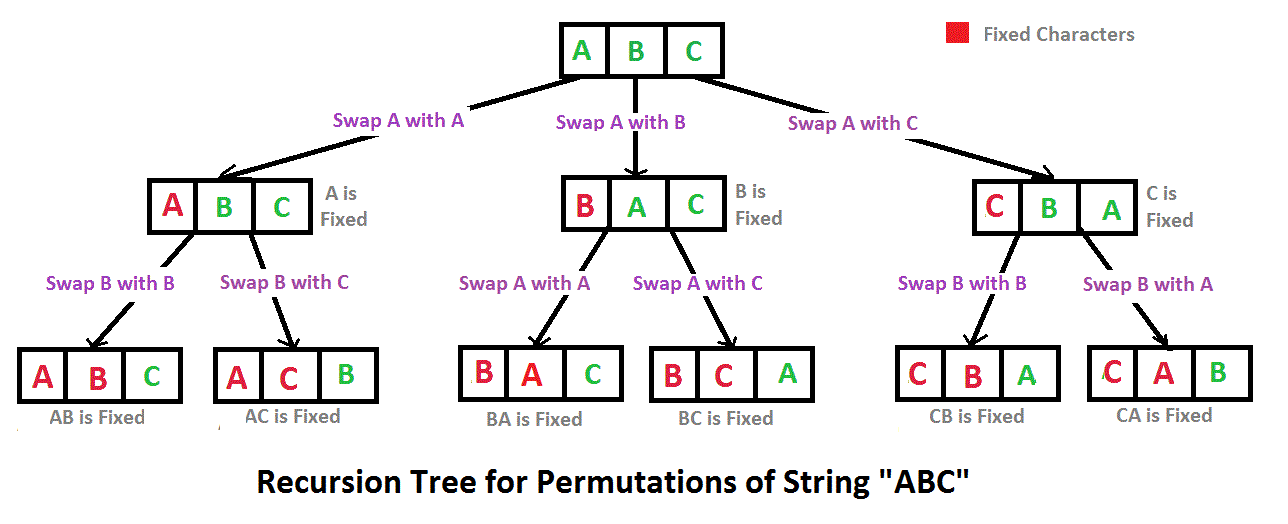import java.util.ArrayList;
import java.util.Collections;
import java.util.List;

public class Solution {
//解法来源：牛客网评论。基于回溯的递归实现。
/**剑指offer的思路解析。
* 步骤：
*
* @param str
* @return
*/
public ArrayList<String> Permutation(String str) {
List <String> res = new ArrayList<String>();
if(str != null && str.length() >0){
PermutationHelp(str.toCharArray(),0,res);
Collections.sort(res); //按字典序 输出字符串数组。
}

return (ArrayList)res;
}
public void PermutationHelp(char[] chars, int index, List<String> list) {
if(index == chars.length -1){ //当递归交换到最后一个位置的时候，就看看list有么有这个字符串，没有的话就放进去。
String val = String.valueOf(chars);
if (!list.contains(val)) {//如果最后list没有这个string，因为可能交换后有重复的
}
}
else {
for (int i = index; i < chars.length; i++) { //循环来执行交换操作，先交换，然后固定这个，下一个交换。最后要交换回来不要影响执行
swap(chars, index, i);
PermutationHelp(chars, index+1, list);//依次固定一个
swap(chars, index, i);
}
}
}
public void swap(char[] chars,int i, int j) {//交换数组中的两个位置中的值
char temp =chars[i];
chars[i] = chars[j];
chars[j] = temp;

}

}

四、数组

1.数组中重复的数字

在一个长度为n的数组里的所有数字都在0到n-1的范围内。 数组中某些数字是重复的，但不知道有几个数字是重复的。也不知道每个数字重复几次。请找出数组中任意一个重复的数字。 例如，如果输入长度为7的数组{2,3,1,0,2,5,3}，那么对应的输出是第一个重复的数字2

思路：比较好的思路的分析为，数组中的数字为0到n-1的范围内。如果这个数组中没有重复的数字，则对应的i位置的数据也为i。可以重排此数组，import java.util.ArrayList;
import java.util.Arrays;
import java.util.HashSet;
import java.util.Set;

public class chongfushuzi {
// 使用排序的方式
public boolean duplicate(int numbers[],int length,int [] duplication) {
if(numbers == null || numbers.length ==0) {
duplication = -1;
return false;
}
Arrays.sort(numbers);
for (int i = 0; i < length -1; i++) {//注意这个i的范围可能越界
if(numbers[i] == numbers[i+1]) {
duplication = numbers[i];
return true;
}
}
return false;

}
//使用额外空间的方法。
public boolean duplicate2(int numbers[],int length,int [] duplication) {
if(numbers == null || numbers.length ==0) {
duplication = -1;
return false;
}
ArrayList<Integer> list = new ArrayList<>();
for (int i = 0; i < length; i++) {
if(list.contains(numbers[i])) {
duplication = numbers[i];
return true;
}
}
return false;
}
//使用额外空间的方法。
public boolean duplicate4(int numbers[],int length,int [] duplication) {
if(length < 2||numbers==null){
return false;
}

Set<Integer> ss = new HashSet<Integer>();
for (int i = 0; i < numbers.length; i++) {
if (ss.contains(numbers[i])) {
duplication = numbers[i];
return true;
} else {
}
}
return false;
}

//比较好的解决方式,时间复杂度O(n),空间复杂度O(1)
//数组中的数字为0到n-1的范围内。
//如果这个数组中没有重复的数字，则对应的i位置的数据也为i。可以重排此数组
public boolean duplicate3(int numbers[],int length,int [] duplication) {
if(numbers == null || numbers.length ==0) {
duplication = -1;
return false;
}
for (int i = 0; i < length; i++) {
if (numbers[i] < 0 || numbers[i] > length - 1) {
duplication = -1;
return false;
}
}
for (int i = 0; i < length; i++) {
while(numbers[i] != i) {
if(numbers[i] == numbers[numbers[i]]) {
duplication = numbers[i];
return true;
}
else {
int tmp = numbers[i];
numbers[i] = numbers[tmp];
numbers[tmp] = tmp;
}

}
}
return false;
}
}


2.构建乘积数组

给定一个数组A[0,1,...,n-1],请构建一个数组B[0,1,...,n-1],其中B中的元素B[i]=A*A*...*A[i-1]*A[i+1]*...*A[n-1]。不能使用除法。

思路：用矩阵的方式，先计算左下三角，再计算右上三角。根据图来分析即可。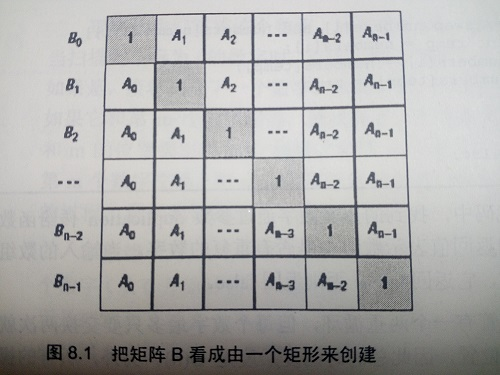public class MultiArray {
// 新建一个新数组B， 对A数组i项左侧自上往下累乘，
// 对A数组i项右侧自下往上累乘 时间复杂度O(n)
public int[] multiply(int[] A) {
// 将B拆分为A *...* A[i-1]和A[n-1]*...*A[i+1] 两部分
if(A == null || A.length ==0)
return A;
int length = A.length;
int [] B = new int[length];
B = 1;
// 先计算左下三角形，此时B只有一个元素，舍为1，
// B不包括A
for (int i = 1; i < length; i++) {
B[i] = B[i-1]*A[i-1];
}
int tmp =1;
//计算右上三角形
for (int i = length -1; i >=0; i--) {
//最终的B[i]是之前乘好的再乘以右边的
B[i] *=tmp;
tmp *= A[i];
}

return B;
}
}

复杂度为O(n^2)的解法如下：(有个对比就行)

public int[] multiplyWithFor(int[] A) {
int len = A.length;
// 定义一个结果对象
int[] result = new int[len];
// 定义一个基本的量
int rst = 1;
for (int i = 0; i < len; i++) {
// 如果相同，就路过继续
for (int j = 0; j < len; j++) {
if (i == j) {
continue;
}
// 如果不同，就相乘
rst *= A[j];
}
result[i] = rst;
rst = 1; // 还原基本的量
}
return result;
}
// 

3.二维数组的查找

在一个二维数组中，每一行都按照从左到右递增的顺序排序，每一列都按照从上到下递增的顺序排序。请完成一个函数，输入这样的一个二维数组和一个整数，判断数组中是否含有该整数。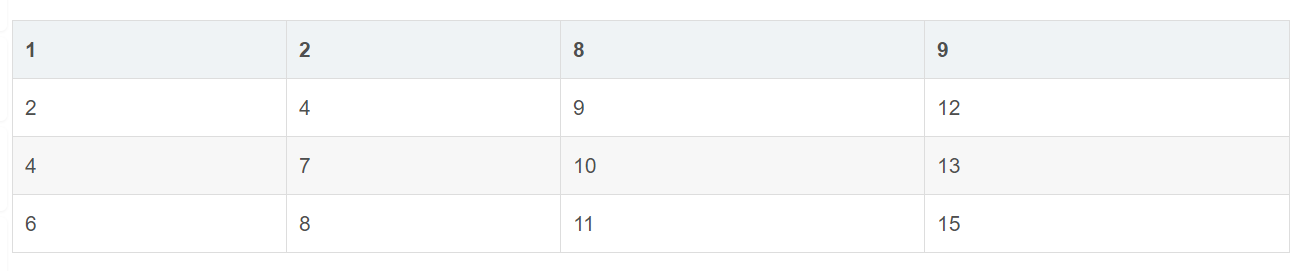思路：

从右上角开始，若小，向下走，删除一行，若大，向左走，删除一列

/*
利用二维数组由上到下，由左到右递增的规律，
那么选取右上角的元素a[row] [col]与target进行比较，
当target小于元素a[row] [col]时，那么target必定在元素a所在行的左边,
即col--；
当target大于元素a[row][col]时，那么target必定在元素a所在列的下边,
即row++；
*

    public boolean Find(int target, int [][] array) {
int row = 0;
int col = array.length -1;
while(row<array.length && col>= 0) {
if (array[row][col]==target) {
return true;
}
else if (array[row][col]<target) {
row++;
}
else {
col--;
}
}
return false;
}

4.数组中只出现一次的数字

一个整型数组里除了两个数字之外，其他的数字都出现了两次。请写程序找出这两个只出现一次的数字。

思路：主要参考左神的思路，辅之以剑指offer思路。主要如下：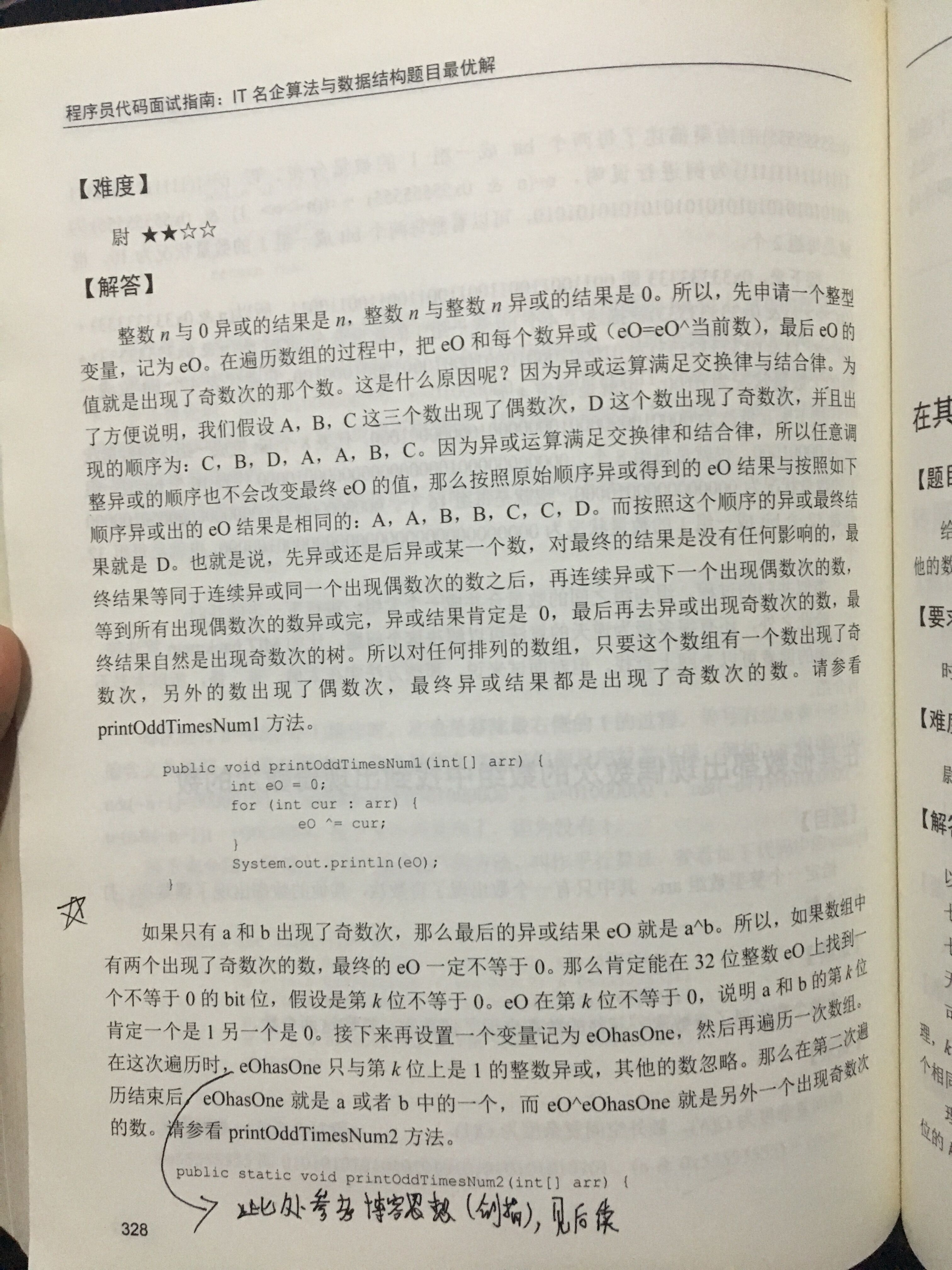参考的部分思路：当只有一个数出现一次时，我们把数组中所有的数，依次异或运算，最后剩下的就是落单的数，因为成对儿出现的都抵消了。

依照这个思路，我们来看两个数（我们假设是AB）出现一次的数组。我们首先还是先异或，剩下的数字肯定是A、B异或的结果，这个结果的二进制中的1，表现的是A和B的不同的位。我们就取第一个1所在的位数，假设是第3位，接着把原数组分成两组，分组标准是第3位是否为1。如此，相同的数肯定在一个组，因为相同数字所有位都相同，而不同的数，肯定不在一组。然后把这两个组按照最开始的思路，依次异或，剩余的两个结果就是这两个只出现一次的数字。

所以，只于k位上是1的异或，就把其中一组的落单的那个异或出来了。此外a^b=d  则a^d=b满足结果的互换。知道异或的结果和其中一个，可以求得另一个。

    public void FindNumsAppearOnce(int [] array,int num1[] , int num2[]) {
int eO = 0,eOne = 0;
for(int num:array)
eO ^=num;
int firstOne = eO &(~eO +1);//求得二进制中第一位1，比如101和011得到010
for(int cur:array)
if ((cur&firstOne) !=0) {//把第k位是1的一组找出来进行异或
eOne ^=cur;
}//最终结果就是第k位是1且落单的那个
num1 = eOne;
num2 = eOne^eO;//异或结果的运算规则。
}

5、和为S的两个数

输入一个递增排序的数组和一个数字S，在数组中查找两个数，使得他们的和正好是S，如果有多对数字的和等于S，输出两个数的乘积最小的。

思路：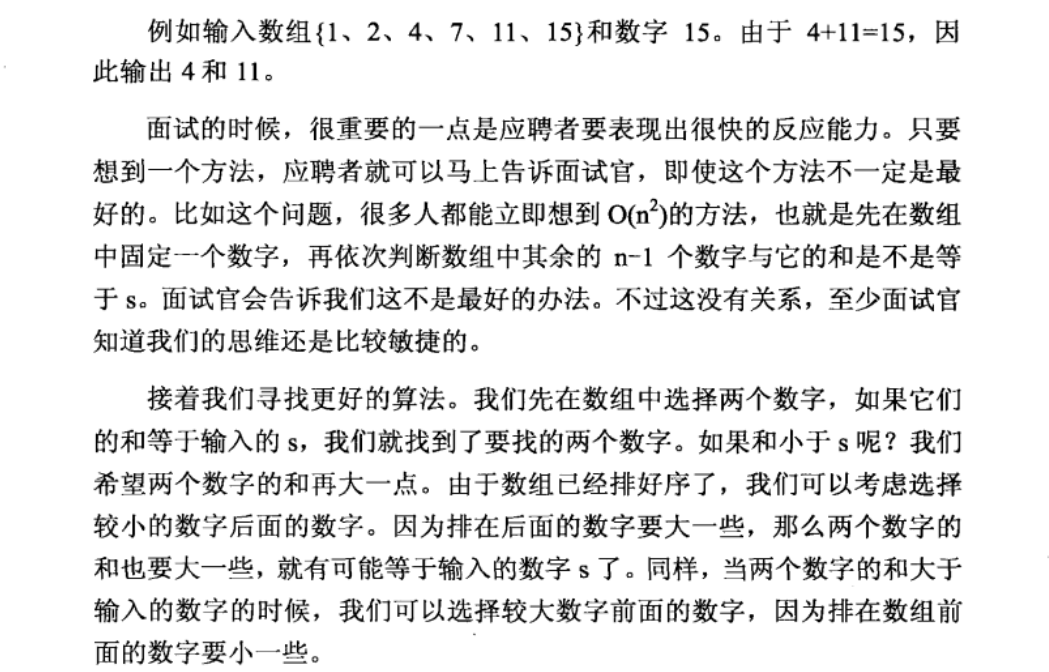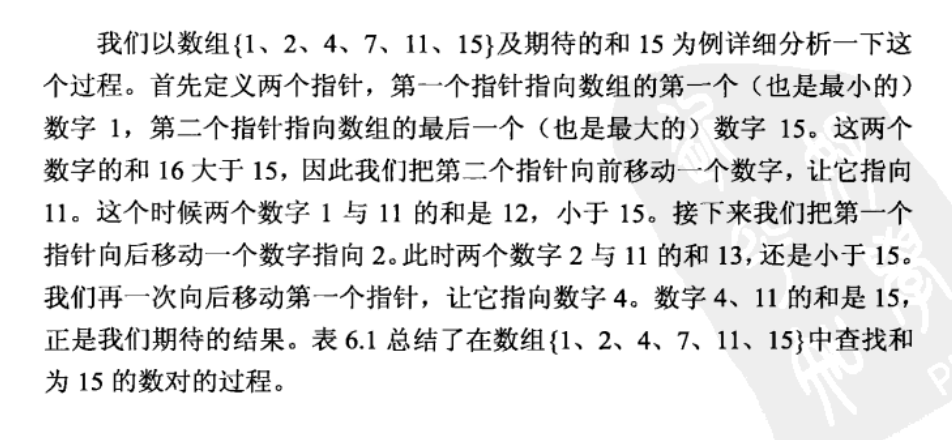// 数列满足递增，设两个头尾两个指针i和j，

// 若ai + aj == sum，就是答案（和一定，两个数字相差越远乘积越小）

// 若ai + aj > sum，aj肯定不是答案之一，j -= 1

// 若ai + aj < sum，ai肯定不是答案之一，i += 1

// O(n)

// 已经排好序，运用数学上的夹

    public ArrayList<Integer> FindNumbersWithSum(int [] array,int sum) {
ArrayList<Integer> list = new ArrayList<>();
if(array == null || array.length <2)
return list;
int low = 0;
int high = array.length -1;
while(low < high) {
int small = array[low];
int big = array[high];
if(small + big == sum) {
break;
}
else if (small+big < sum)
low++;
else
high--;

}
return list;
}

6.和为S的连续正数序列

小明很喜欢数学,有一天他在做数学作业时,要求计算出9~16的和,他马上就写出了正确答案是100。但是他并不满足于此,他在想究竟有多少种连续的正数序列的和为100(至少包括两个数)。没多久,他就得到另一组连续正数和为100的序列:18,19,20,21,22。现在把问题交给你,你能不能也很快的找出所有和为S的连续正数序列? Good Luck!

思路：剑指offer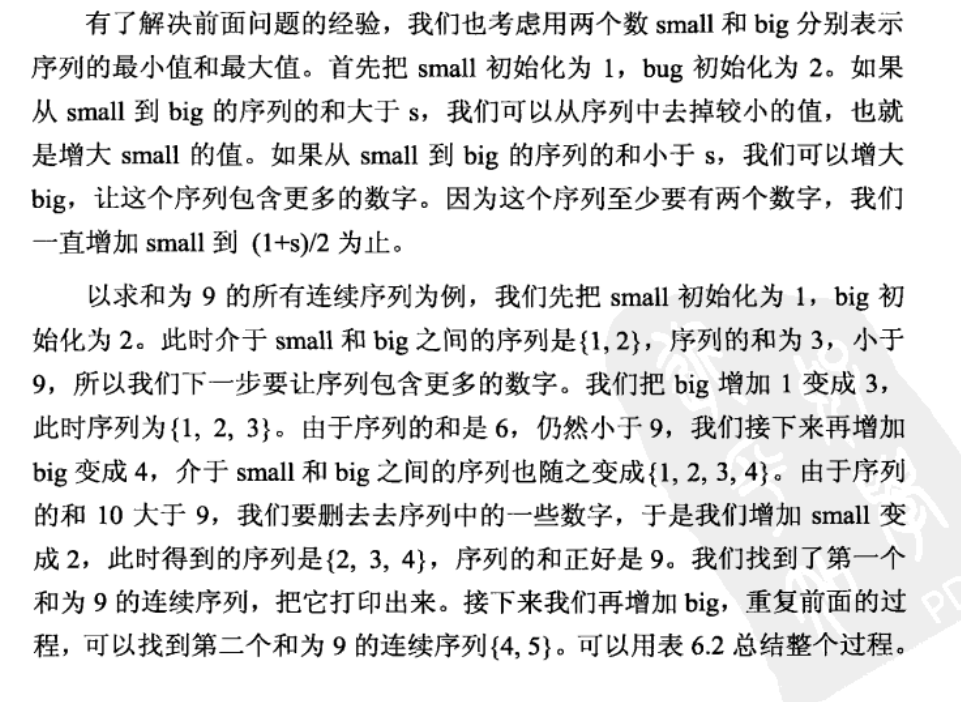/*
*初始化small=1，big=2;
*small到big序列和小于sum，big++;大于sum，small++;
*当small增加到(1+sum)/2是停止
*/
public ArrayList<ArrayList<Integer> > FindContinuousSequence(int sum) {
ArrayList<ArrayList<Integer>> res = new ArrayList<>();
ArrayList<Integer> list = new ArrayList<>();
if(sum < 3) //不重复，最少有两个数字
return res;
int small = 1;
int big = 2;
//因为是两个数，假设small是这个
//，big假设也是这个，和为sum+1，所以到此就可以停了，后面肯定更大
while(small !=(sum+1)/2) {
int cursum = SumOfList(small, big);
if(cursum == sum) {
for (int i = small; i <= big; i++) {
}
list.clear();//清理掉
big++;//找到一组，继续big++，找下一组满足要求的。
}
else if (cursum > sum) {
small++;
}
else {
big++;
}
}
return res;
}
//计算list内的数据的和
public int SumOfList(int small, int big) {
int sum = 0;
for (int i = small; i <= big; i++) {
sum +=i;
}
return sum;
}

7、调整数组顺序使奇数位于偶数前面

输入一个整数数组，实现一个函数来调整该数组中数字的顺序，使得所有的奇数位于数组的前半部分，所有的偶数位于数组的后半部分，并保证奇数和奇数，偶数和偶数之间的相对位置不变。

import java.util.ArrayList;
//import java.util.Arrays;
public class Solution {
public void reOrderArray(int [] array) {
ArrayList<Integer> jlist = new ArrayList<Integer>();
ArrayList<Integer> olist = new ArrayList<Integer>();
for (int i = 0; i < array.length; i++) {
if (array[i]%2 == 0)
else
//intValue是反过来

}
int temp = 0;
for (int i = 0; i < jlist.size(); i++) {
//System.out.println(jlist.get(i));
array[temp] = jlist.get(i).intValue();
temp++;
}
}
//前一种方法的执行时间低，但是需要额外的空间，后一种方法执行时间高一点，但是不需要额外的空间
/*public void reOrderArray(int[] array) {
int temp = 0;
for (int i = 0; i < array.length - 1; i++) {
for (int j = 0; j < array.length - 1 - i; j++) {
// 前偶后奇数就交换
if ((array[j] & 1) == 0 && (array[j + 1] & 1) == 1) {  //与1位与得1就是奇数，1只有最后一位是1
temp = array[j];
array[j] = array[j+1];
array[j+1] = temp;
}
}
}
} */
}

8、数组中出现次数超过一半的数字

数组中有一个数字出现的次数超过数组长度的一半，请找出这个数字。例如输入一个长度为9的数组{1,2,3,2,2,2,5,4,2}。由于数字2在数组中出现了5次，超过数组长度的一半，因此输出2。如果不存在则输出0。

public class Solution {
/**自己的空间复杂度的思路，哈希表记录。
* 此外：博客，基于快排、基于阵地。此处采用基于防守阵地的方式来进行。
* 左的思路。
* 一次再数组中删除两个不同的数，最后剩下的数   有可能    是超过一半的。所以要检验一下。 一个数出现次数大于一半，他肯定会被剩下来，但是剩下来的缺不一定满足。
* 算法步骤：
* 如果times为0，就把候选设为当前值。
* 如果下个数和候选一样，times就++。
* 如果下个数和候选不一样，times就--。相当于对子，同归于尽。因为超过一半的数肯定超过剩下的所有数。所以和这个数对，这个数肯定会剩下来。
* 但是剩下的数不一定是，比如 1 2 3 剩下3 比如 1 2 1 3 3 3 2 2 也是剩下3.所以要余外的判断，看是否这个数真的超过。
* @param array
* @return
*/
public int MoreThanHalfNum_Solution(int [] array) {
int cand = 0;
int times = 0;
for (int i = 0; i < array.length; i++) {
if(times == 0){
cand = array[i];
times = 1;
}
else if (array[i] == cand) {
times++;
}
else {
times--;
}
}
times = 0;
for (int i = 0; i < array.length; i++) {
if(array[i] == cand)
times++;
}
if (times*2 > array.length) {
return cand;
}
else
return 0;
}
}

9、连续子数组的最大和

题目描述

HZ偶尔会拿些专业问题来忽悠那些非计算机专业的同学。今天测试组开完会后,他又发话了:在古老的一维模式识别中,常常需要计算连续子向量的最大和,当向量全为正数的时候,问题很好解决。但是,如果向量中包含负数,是否应该包含某个负数,并期望旁边的正数会弥补它呢？例如:{6,-3,-2,7,-15,1,2,2},连续子向量的最大和为8(从第0个开始,到第3个为止)。给一个数组，返回它的最大连续子序列的和，你会不会被他忽悠住？(子向量的长度至少是1)

/* 算法时间复杂度O（n） 用total记录累计值，maxSum记录和最大 基于思想：对于一个数A，若是A的左边累计数非负，那么加上A能使得值不小于A，认为累计值对 整体和是有贡献的。如果前几项累计值负数，则认为有害于总和，total记录当前值。 此时 若和大于maxSum 则用maxSum记录下来 */

public class Solution {
/**
* 不是看当前的值，而是看当前的累计值，如果当前累计为负数，那么加上现在这个数，
* 肯定和就小了，所以继续从当前开始累计，如果为正，那就继续加，
* 但是要时刻保存下最大值来，因为后面的累计有可能小。
* @param array
* @return
*/
public int FindGreatestSumOfSubArray(int[] array) {
if(array.length == 0)
return 0;
int total = array;//当前的前面的和累计
int maxsum = array;//记录可能的最大值
for (int i = 1; i < array.length; i++) {
if(total >= 0)//
total = total + array[i];
else
total = array[i];
if(total>maxsum)
maxsum = total;
}
return maxsum;
}
}

10、把数组排成最小的数

题目描述

输入一个正整数数组，把数组里所有数字拼接起来排成一个数，打印能拼接出的所有数字中最小的一个。例如输入数组{3，32，321}，则打印出这三个数字能排成的最小数字为321323。

/* 解题思路： * 考虑到大数问题，先将整型数组转换成String数组，然后将String数组排序，最后将排好序的字符串数组拼接出来。关键就是制定排序规则。 * 排序规则如下： * 若ab > ba 则 a > b， * 若ab < ba 则 a < b， * 若ab = ba 则 a = b； * 解释说明： * 比如 "3" < "31"但是 "331" > "313"，所以要将二者拼接起来进行比较 */

import java.util.Arrays;
import java.util.Comparator;

public class Solution {

/**
* 主要就是规则的制定，将两个数字转换为字符串，防止溢出
* 假设两个数字m和n，拼接有mn和nm
* 如果mn>nm, 我们打印nm，此时定义n小于m。（此处小于是我们定义的）
* 不是m和n的大小关系，
* 而是看拼了之后的大小，来决定m和n的大小。
* 如果mn<nm 那么就是m小于n。
*
* @param numbers
* @return
*/
public String PrintMinNumber(int [] numbers) {
if(numbers == null || numbers.length == 0)
return "";
int len = numbers.length;
String[] str = new String[len];
StringBuffer sb = new StringBuffer();
//将数字型的放在字符串数组中。
for (int i = 0; i < len; i++) {
str[i] = String.valueOf(numbers[i]);
}
//根据定义的规则重新堆str进行升序排序
Arrays.sort(str, new Comparator<String>() {

@Override
public int compare(String s1, String s2) {
// TODO Auto-generated method stub
String c1 = s1 + s2;
String c2 = s2 + s1;

return c1.compareTo(c2);
}

});
//根据规则排好序，将结果依次放入stringbuffer中就行了
for (int i = 0; i < len; i++) {
sb.append(str[i]);
}

return sb.toString();
}
}

11、数组中的逆序对

题目描述

在数组中的两个数字，如果前面一个数字大于后面的数字，则这两个数字组成一个逆序对。输入一个数组,求出这个数组中的逆序对的总数P。并将P对1000000007取模的结果输出。 即输出P%1000000007

输入描述:

题目保证输入的数组中没有的相同的数字

数据范围：

对于%50的数据,size<=10^4

对于%75的数据,size<=10^5

对于%100的数据,size<=2*10^5

示例1

输入1,2,3,4,5,6,7,0

输出7

public class Solution {

/**主要是剑指offer的思想，用了归并排序，用count来记录数量
* 所不同的是用的--而不是++
* 每一次归并之前，记录当前有的个数，
* @param array
* @return
*/
int count = 0;
public int InversePairs(int [] array) {
if(array == null || array.length ==0)
return 0;
mergeSort(array, 0, array.length -1);
return count;

}
public void mergeSort(int []array, int start, int end) {
if(start < end) {
int mid = (start + end)/2;
mergeSort(array, start, mid);
mergeSort(array, mid + 1, end);
merge(array, start, mid, mid+ 1, end);
}
}
public void merge(int []array,int start1,int end1, int start2, int end2) {
int i = end1;
int j = end2;
int k = end2 - start1 ;
int [] temp = new int[end2- start1 +1];
while(i >= start1 && j >=start2) {
if(array[i] > array[j]) {
//假设此时两个归并的是17 19 22 || 16 18 21
//那么22大于21，所以可以看出对应22
//有三个，22 16 22 18 22 21
temp[k--] = array[i--];
count = count + j - start2 +1;
count %= 1000000007;
}
else
temp[k--] = array[j--];
}
while(i >= start1)
temp[k--] = array[i--];
while(j >= start2)
temp[k--] = array[j--];

int m = start1;
for(int element:temp)
array[m++] = element;

}
}

12、数字在排序数组中出现的次数

统计一个数字在排序数组中出现的次数。

思路：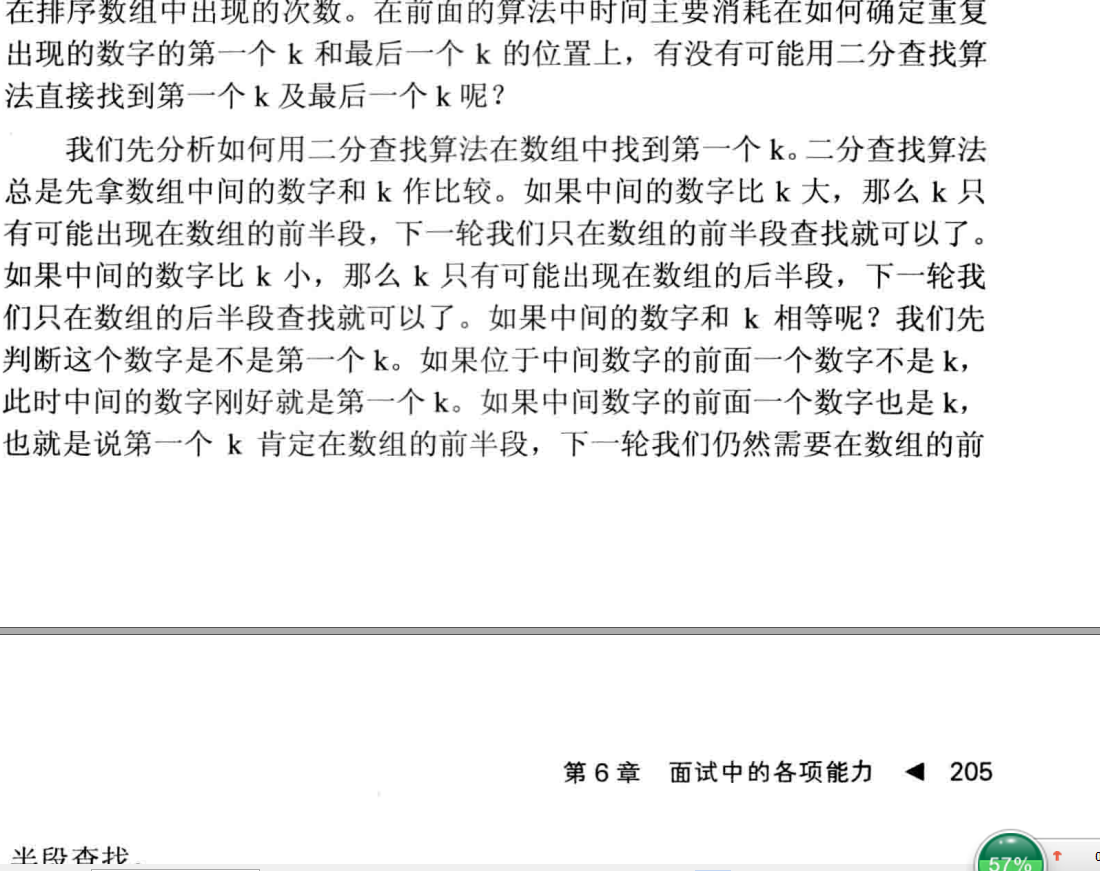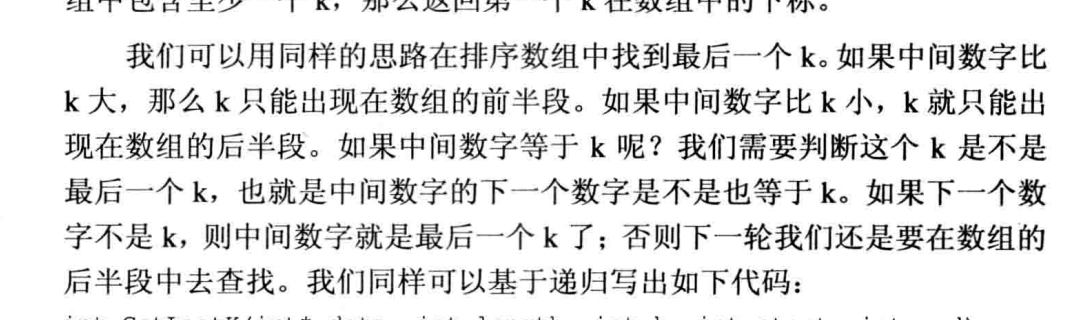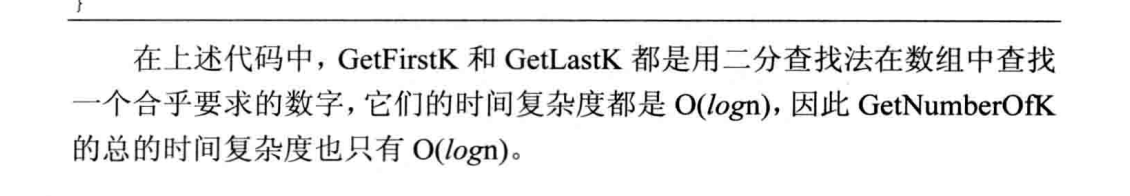public class Solution {
/**
* 博客上的解题思路，也就是剑指offer的思路
* 首先用递归二分法找到第一个k和最后一个k，然后得到个数
* @param array
* @param k
* @return
*/
public int GetNumberOfK(int [] array , int k) {
int num = 0;
if (array != null && array.length >0) {
int firstk = getFirstK(array, k,0, array.length-1);
int lastk = getLastK(array, k,0, array.length-1);
if (firstk > -1 && lastk > -1)
num = lastk -firstk +1;

}
return num;
}
/*
* 找到第一个出现的数字的下标
*/
public int getFirstK(int [] array, int k,int start, int end) {
if(start > end)
return -1;
int midindex = (start + end)/2;
int middata = array[midindex];
if (middata == k) {
//判断是不是第一个K，前一个不等于K，就是第一个K
if(midindex > 0 && array[midindex - 1]!=k||midindex == 0)
return midindex;
else
end = midindex -1;//如果不是第一个k，那么肯定是在前面找，所以end要往前放

}
else if (middata > k) {
end = midindex -1; //二分，如果这个大于k，所以要在前面找
}
else
start = midindex + 1;// 如果小于k，说明要往后找
return getFirstK(array,k, start, end);
}

/*
* 找到最后一个出现的数字的下标
*/
public int getLastK(int [] array, int k,int start, int end) {
if(start > end)
return -1;
int midindex = (start + end)/2;
int middata = array[midindex];
if(middata == k) {
//判断是不是最后一个K，后一个不等于K，就是最后一个K
if(midindex < array.length-1 && array[midindex + 1]!= k||midindex ==array.length -1)
return midindex;
else
start = midindex + 1;
}
else if (middata > k) {
end = midindex - 1;
}
else
start = midindex +1;
return getLastK(array, k,start, end);
}
}

五、发散思维能力

1.求1+2+3+...+n

求1+2+3+...+n，要求不能使用乘除法、for、while、if、else、switch、case等关键字及条件判断语句（A?B:C）。

思路：巧用递归，用了短路，使递归结束。

// &&两侧的表达式结果必须为boolean型，

// 所有&&右侧要用一个无关变量a判断是否与result相等，

// 让右侧的表达式返回boolean型。不管返回的是true还是false，

// 我们的目的仅仅是让&&右侧的表达式执行。

// &&连接的表达式，必须要将最终的boolean结果赋给变量，否则编译报错！

一直执行递归，当n=0时，短路，不执行，开始执行result+n，一直回溯就把n给加上来。

    public int Sum_Solution(int n) {
int result =0;
int temp = 0;
boolean flag = (n>0) && temp == (result = Sum_Solution(n-1));
result += n;
return result;
}

2.不用加减乘除做加法

写一个函数，求两个整数之和，要求在函数体内不得使用+、-、*、/四则运算符号。

思路：

首先看十进制是如何做的： 5+7=12，三步走

第一步：相加各位的值，不算进位，得到2。

第二步：计算进位值，得到10. 如果这一步的进位值为0，那么第一步得到的值就是最终结果。

第三步：重复上述两步，只是相加的值变成上述两步的得到的结果2和10，得到12。

同样我们可以用三步走的方式计算二进制值相加： 5-101，7-111 第一步：相加各位的值，不算进位，得到010，二进制每位相加就相当于各位做异或操作，101^111。

第二步：计算进位值，得到1010，相当于各位做与操作得到101，再向左移一位得到1010，(101&111)<<1。

第三步重复上述两步， 各位相加 010^1010=1000，进位值为100=(010&1010)<<1。
继续重复上述两步：1000^100 = 1100，进位值为0，跳出循环，1100为最终结果。

    public int Add(int num1,int num2) {
int sum = num1;//保证当num2为0时候返回num1
int carry = 0;
while(num2 != 0) {
sum = num1 ^ num2;
carry = (num1 & num2) << 1;
num1 = sum;
num2 = carry;

}
return sum;
}

使用递归的方式实现：

	public int Add(int num1, int num2) {
if (num2 == 0)
return num1;
return Add(num1 ^ num2, (num1 & num2) << 1);
}

3、旋转数组的最小数字

把一个数组最开始的若干个元素搬到数组的末尾，我们称之为数组的旋转。 输入一个非减排序的数组的一个旋转，输出旋转数组的最小元素。 例如数组{3,4,5,1,2}为{1,2,3,4,5}的一个旋转，该数组的最小值为1。 NOTE：给出的所有元素都大于0，若数组大小为0，请返回0

思路：思路：利用二分法，找到中间的数，然后和最左边的值进行比较，若大于最左边的数，则最左边从mid开始，若小于最右边值，则最右边从mid开始。若左中右三值相等，则取mid前后值中较小的数。

import java.util.ArrayList;
public class Solution {
public int minNumberInRotateArray(int [] array) {
int front = 0;
int rear = array.length-1;
int mid = (front + rear)/2;
while(front < rear){
if(rear - front == 1){
break;
}
mid = (front + rear)/2;
if(array[mid] >= array[front]){
front = mid;
}
else{
rear = mid;
}
}
return array[rear];
}
}

六、其他

1.整数中1出现的次数（从1到n中的整数中1出现的次数）

求出1~13的整数中1出现的次数,并算出100~1300的整数中1出现的次数？为此他特别数了一下1~13中包含1的数字有1、10、11、12、13因此共出现6次,但是对于后面问题他就没辙了。ACMer希望你们帮帮他,并把问题更加普遍化,可以很快的求出任意非负整数区间中1出现的次数。

思路：主要是根据编程之美博客的思路，左程云的思路类似，但是感觉代码稍微麻烦一点。

编程之美上给出的规律：
1、 如果第i位（自右至左，从1开始标号）上的数字为0（小于x），则第i位可能出现1的次数由更高位决定（若没有高位，视高位为0），等于更高位数字 * 当前位数的权重10^(i-1)。

2、如果第i位上的数字为1(等于x)，则第i位上可能出现1的次数不仅受更高位影响，还受低位影响（若没有低位，视低位为0），等于更高位数字 * 当前位数的权重10^(i-1)+（低位数字+1）。

3、如果第i位上的数字大于1(大于x)，则第i位上可能出现1的次数仅由更高位决定（若没有高位，视高位为0），等于（更高位数字+1） * 当前位数的权重10^(i-1)。

这个思路中，如果不是求1出现的次数，而是求其他1-9任意一个次数，就是比较大于、等于、小于这个数就行了，分别对应上边的三条规则。0的出现次数需要余外计算。

X的数目
这里的 X∈[1,9] ，因为 X=0 不符合下列规律，需要单独计算。

首先要知道以下的规律：
从 1 至 10，在它们的个位数中，任意的 X 都出现了 1 次。
从 1 至 100，在它们的十位数中，任意的 X 都出现了 10 次。
从 1 至 1000，在它们的百位数中，任意的 X 都出现了 100 次。

依此类推，从 1 至 10^ i ，在它们的左数第二位（右数第 i 位）中，任意的 X 都出现了 10^(i-1) 次。

这个规律很容易验证，这里不再多做说明。
接下来以 n=2593,X=5 为例来解释如何得到数学公式。从 1 至 2593 中，数字 5 总计出现了 813 次，其中有 259 次出现在个位，260 次出现在十位，294 次出现在百位，0 次出现在千位。

现在依次分析这些数据， 首先是个位。从 1 至 2590 中，包含了 259 个 10，因此任意的 X 都出现了 259 次。最后剩余的三个数 2591, 2592 和 2593，因为它们最大的个位数字 3 < X，因此不会包含任何 5。（也可以这么看，3 < X，则个位上可能出现的X的次数仅由更高位决定，等于更高位数字（259）*10^(1-1)=259）。

然后是十位。从 1 至 2500 中，包含了 25 个 100，因此任意的 X 都出现了 25×10=250 次。剩下的数字是从 2501 至 2593，它们最大的十位数字 9 > X，因此会包含全部 10 个 5。最后总计 250 + 10 = 260。（也可以这么看，9>X，则十位上可能出现的X的次数仅由更高位决定，等于更高位数字（25+1）*10^(2-1)=260）。

接下来是百位。从 1 至 2000 中，包含了 2 个 1000，因此任意的 X 都出现了 2×100=200 次。剩下的数字是从 2001 至 2593，它们最大的百位数字 5 == X，这时情况就略微复杂，它们的百位肯定是包含 5 的，但不会包含全部 100 个。如果把百位是 5 的数字列出来，是从 2500 至 2593，数字的个数与百位和十位数字相关，是 93+1 = 94。最后总计 200 + 94 = 294。（也可以这么看，5==X，则百位上可能出现X的次数不仅受更高位影响，还受低位影响，等于更高位数字（2）*10^(3-1)+（93+1）=294）。

最后是千位。现在已经没有更高位，因此直接看最大的千位数字2< X，所以不会包含任何 5。（也可以这么看，2< X，则千位上可能出现的X的次数仅由更高位决定，等于更高位数字（0）*10^(4-1)=0）。
到此为止，已经计算出全部数字 5 的出现次数。

总结一下就是：

求x出现的次数，分别拆分，然后根据编程之美判断这一位与x的大小关系，分情况写出出现的次数。

  public int NumberOf1Between1AndN_Solution(int n) {
int low,cur,temp,i=1;
int high = n;//记录高位数
int total = 0; //总的1的数量
if(n < 1)
return 0;
while(high!=0) {
//记忆2593 此时i=2，依次拆分25 9 3
high = n/powerBaseof10(i); 获取第i位的高位
temp = n%powerBaseof10(i);
cur = temp/powerBaseof10(i-1);// 获取第i位
low = temp%powerBaseof10(i-1);// 获取第i位的低位
if(cur ==1) {
total += high * powerBaseof10(i-1) + low +1;
}
else if (cur > 1) {
total += (high + 1) * powerBaseof10(i -1);
}
else {
total += high * powerBaseof10(i-1);
}
i++;
}
}
//就是求10^base次方
public int powerBaseof10(int base) {
return (int)Math.pow(10, base);
}

2.扑克牌顺子

LL今天心情特别好,因为他去买了一副扑克牌,发现里面居然有2个大王,2个小王(一副牌原本是54张^_^)...他随机从中抽出了5张牌,想测测自己的手气,看看能不能抽到顺子,如果抽到的话,他决定去买体育彩票,嘿嘿！！“红心A,黑桃3,小王,大王,方片5”,“Oh My God!”不是顺子.....LL不高兴了,他想了想,决定大\小 王可以看成任何数字,并且A看作1,J为11,Q为12,K为13。上面的5张牌就可以变成“1,2,3,4,5”(大小王分别看作2和4),“So Lucky!”。LL决定去买体育彩票啦。 现在,要求你使用这幅牌模拟上面的过程,然后告诉我们LL的运气如何。为了方便起见,你可以认为大小王是0。

思路：剑指和博客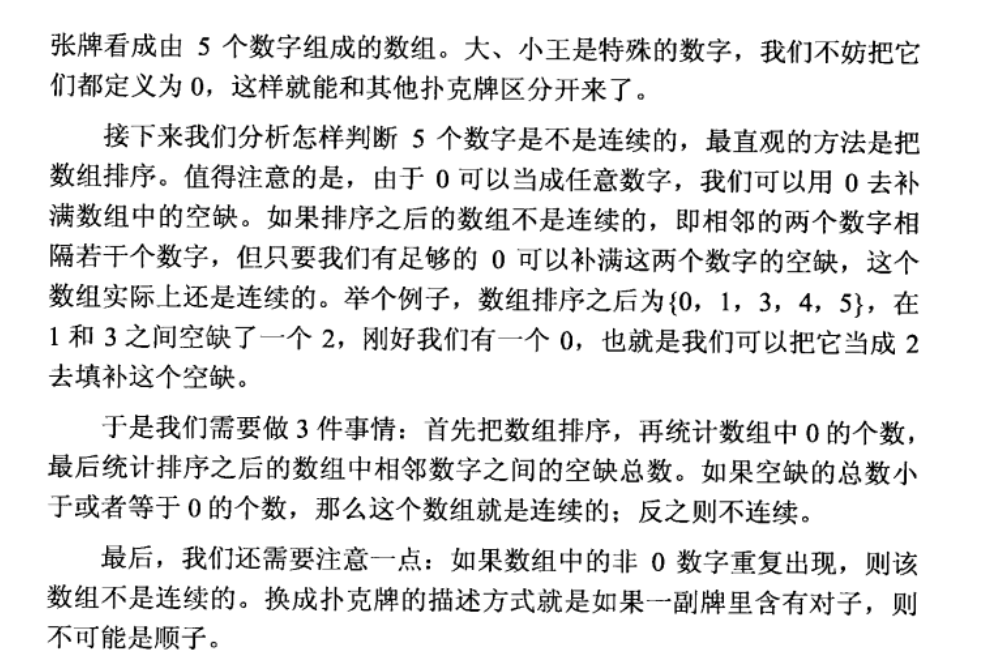思路:用数组记录五张扑克牌，将数组调整为有序的，若0出现的次数>=顺子的差值，即为顺子。

    public boolean isContinuous(int [] numbers) {
if(numbers == null || numbers.length ==0)
return false;
int zero = 0;//记录0的个数
int diff = 0;//记录空缺的数
Arrays.sort(numbers);

for (int i = 0; i < numbers.length -1; i++) {
if(numbers[i] == 0) {
zero++; //找不到非0的就继续下一次循环
continue;
}
if (numbers[i] != numbers[i+1]) {
diff += numbers[i+1] - numbers[i] -1 ;//4 和 8，中间空缺3
}
else
return false;//说明有对子，肯定不是顺子

}
if(diff<= zero)
return true;//如果diff小于zero，那么zero放在最后就行，因为任意值
return false;
}

3.孩子们的游戏（圆圈中剩下的数）

每年六一儿童节,牛客都会准备一些小礼物去看望孤儿院的小朋友,今年亦是如此。HF作为牛客的资深元老,自然也准备了一些小游戏。其中,有个游戏是这样的:首先,让小朋友们围成一个大圈。然后,他随机指定一个数m,让编号为0的小朋友开始报数。每次喊到m-1的那个小朋友要出列唱首歌,然后可以在礼品箱中任意的挑选礼物,并且不再回到圈中,从他的下一个小朋友开始,继续0...m-1报数....这样下去....直到剩下最后一个小朋友,可以不用表演,并且拿到牛客名贵的“名侦探柯南”典藏版(名额有限哦!!^_^)。请你试着想下,哪个小朋友会得到这份礼品呢？(注：小朋友的编号是从0到n-1)

思路：使用递推或者是循环链表的方式。

循环链表的方式：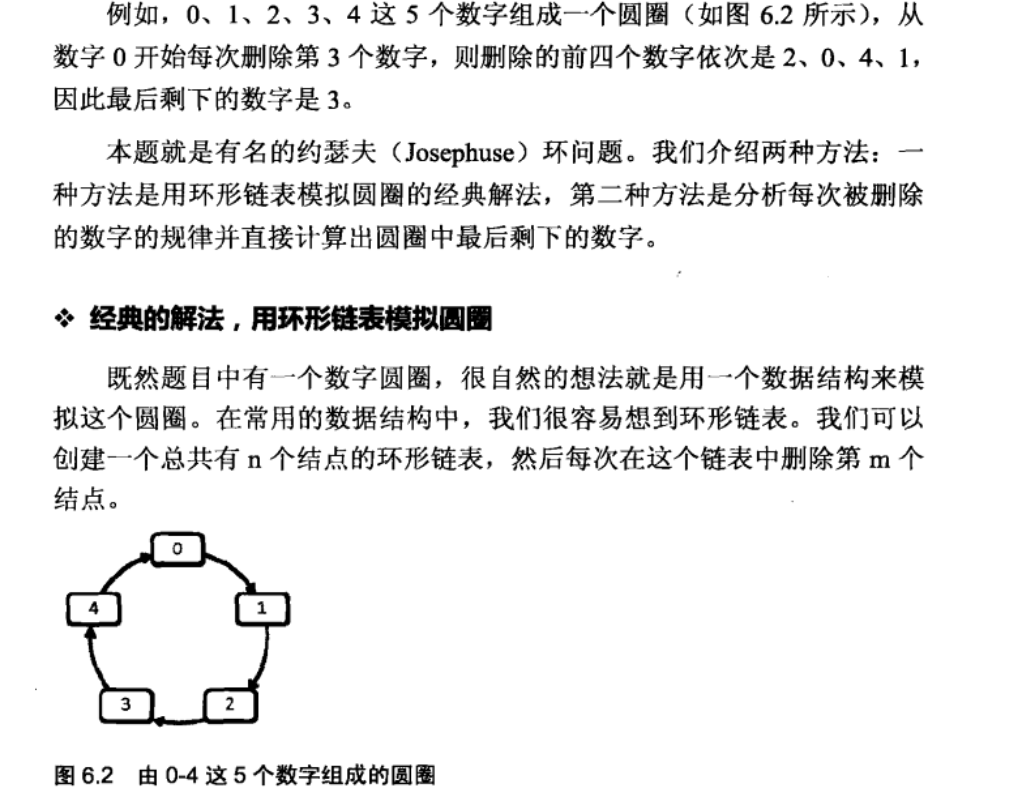bt = (bt + m -1)%list.size();解析：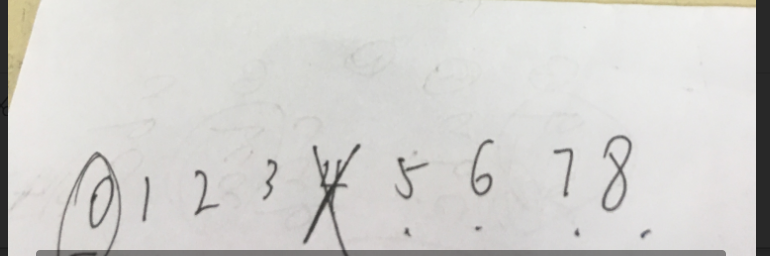假设有9个人，m=5，那么第一次是去掉4，然后从5开始数，下次刚好是0.

   public int LastRemaining_Solution(int n, int m) {
for (int i = 0; i < n; i++) {
}
int bt = 0;
while(list.size() >1) {
删除报数为m-1的人（从0开始）
//解析看前面
bt = (bt + m -1)%list.size();
list.remove(bt);
}
return list.size()==1?list.get(0):-1;
}

递推公式的：主要剑指offer上有，没有细看。

// 第一种方法使用递推公式
public int LastRemaining_Solution(int n, int m) {
if (m < 1 || n < 1)
return -1;
int last = 0;
// i代表有目前有个人
for (int i = 2; i <= n; i++)
last = (last + m) % i;
return last;
}

4、替换空格

在一个二维数组中（每个一维数组的长度相同），每一行都按照从左到右递增的顺序排序，每一列都按照从上到下递增的顺序排序。请完成一个函数，输入这样的一个二维数组和一个整数，判断数组中是否含有该整数。

思路：新定义一个往后追加。

public class Solution {
public String replaceSpace(StringBuffer str) {
StringBuffer newstr = new StringBuffer();
for(int i = 0;i<str.length();i++)
{
if(str.charAt(i) != ' ')
{
newstr.append(str.charAt(i));

}
else
{
newstr.append("%20");
}
}
return newstr.toString();
}
}

5、斐波那契数列

大家都知道斐波那契数列，现在要求输入一个整数n，请你输出斐波那契数列的第n项（从0开始，第0项为0）。

n<=39

递归简洁，但是效率不高。循环O（N）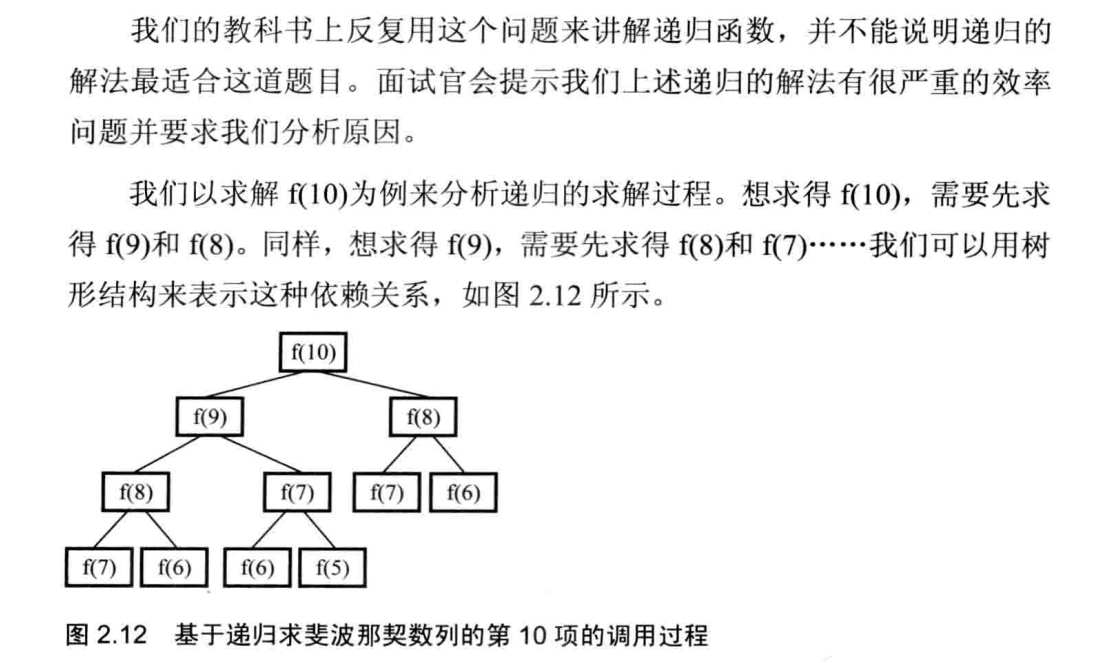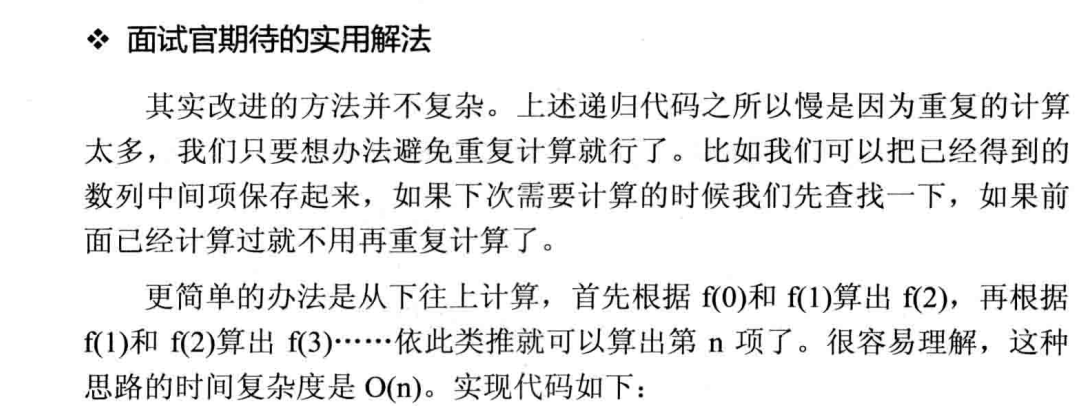public class Solution {
public int Fibonacci(int n) {
/*int pretwo = 0;
int preone = 1;
int res = 0;
if(n==0)
return 0;
if(n==1)
return 1;
for(int i = 2;i <= n;i++)
{
res = preone + pretwo;
pretwo = preone;
preone = res;

}
return res;*/
int res = 0;

if(n==1 || n==2)
return 1;
else if(n == 0)
return 0;
else {
res = Fibonacci(n-1) + Fibonacci(n-2);
}
return res;
}
}

6、跳台阶

一只青蛙一次可以跳上1级台阶，也可以跳上2级。求该青蛙跳上一个n级的台阶总共有多少种跳法（先后次序不同算不同的结果）。

思路：斐波拉契数序列，初始条件n=1:只能一种方法，n=2:两种
对于第n个台阶来说，只能从n-1或者n-2的台阶跳上来，所以
F(n) = F(n-1) + F(n-2)

public class Solution {
public int JumpFloor(int target) {
int res = 0;
if(target == 1)
return 1;
else if(target == 2)
return 2;
else
res = JumpFloor(target-1) + JumpFloor(target-2);
return res;
}
}

7、变态跳台阶

一只青蛙一次可以跳上1级台阶，也可以跳上2级……它也可以跳上n级。求该青蛙跳上一个n级的台阶总共有多少种跳法。

思路：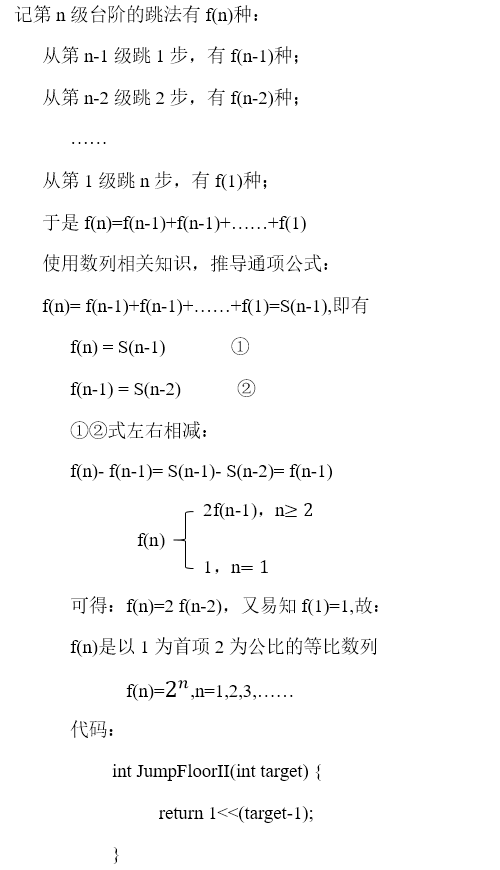public class Solution {
public int JumpFloorII(int target) {
int res = 1;
for(int i =1;i <=target-1;i++){

res = res * 2;

}
return res;
}
}

8、矩形覆盖

我们可以用2*1的小矩形横着或者竖着去覆盖更大的矩形。请问用n个2*1的小矩形无重叠地覆盖一个2*n的大矩形，总共有多少种方法？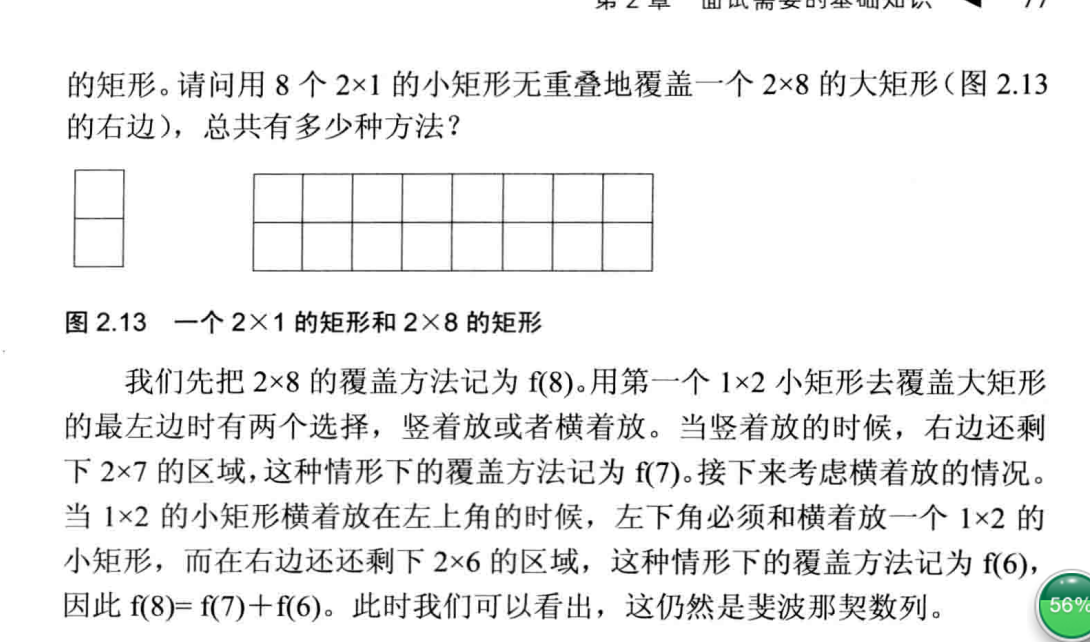public class Solution {
public int RectCover(int target) {
int res = 0;
if(target == 0)
return 0;
else if(target == 2)
return 2;
else if(target ==1)
return 1;
else
res = RectCover(target - 1) + RectCover(target-2);
return res;
/*int result = 0;
if (target > 0) {
if (target <= 2)
return target;
else
return result = RectCover(target - 1) + RectCover(target - 2);
}
return result;  */
}
}

9、数值的整数次方

给定一个double类型的浮点数base和int类型的整数exponent。求base的exponent次方

public class Solution {
public double Power(double base, int exponent) {
//本题注意的是exponent小于0 或者 等于 0 的情况 还有base为0的情况
double result = 1;
if(exponent < 0){
base = 1/base;
exponent = (-1) * exponent;
for (int i = 1; i <= exponent; i++) {
result = result *base;
}
}
else{
for (int i = 1; i <= exponent; i++) {
result = result *base;
}
}
return result;
}
}

/*{
double res = 0;

if (exponent == 0) {
return 1.0;
}
if (exponent > 0) {
res = mutiply(base,exponent);
}else {
res = mutiply(1/base,-exponent);
}
return res;
}

public double mutiply(double base, int e) {
double sum = 1;
for (int i = 0; i < e; i++) {
sum = sum * base;
}
return sum;
}
}*/
/*博客
public class Solution {
//时间复杂度O(n)
public double Power(double base, int exponent) {
int n = Math.abs(exponent);
if (n == 0)
return 1;
if (n == 1)
return base;
//以上两个if判断可省。for循环中判断
double result =
1.0
;
for (int i = 0; i < n; i++) {
result *= base;
}
if (exponent < 0) {
result = 1 / result;
}
return result;
}
}

*/



10、顺时针打印矩阵

输入一个矩阵，按照从外向里以顺时针的顺序依次打印出每一个数字，例如，如果输入如下4 X 4矩阵： 1 2 3 4 5 6 7 8 9 10 11 12 13 14 15 16 则依次打印出数字1,2,3,4,8,12,16,15,14,13,9,5,6,7,11,10.

思路：左神的

import java.util.ArrayList;
public class Solution {
/**
* 基本思路：
* 1.左上角的坐标和右下角的坐标固定了一圈矩阵，先打印这一圈矩阵。其中，对矩阵只有一行或者一列分别打印。
* 2.打印完了这一圈，左上角坐标都+1 ，右下角的都减一，到更内一圈。
*比如：4*4的矩阵，(0,0)--(3,3)然后，(1,1)--(2,2)
* 3.当左上角跑到右下角下面就结束了。
* —---------|
|         |
|         |
|----------
* @param matrix
* @return
*/
public  ArrayList<Integer> printMatrix(int [][] matrix){
ArrayList<Integer> list = new ArrayList<Integer>();
int topRow = 0;
int topCol = 0;
int downRow = matrix.length - 1;
int downCol = matrix.length - 1;
while (topRow <= downRow && topCol <= downCol) { //当满足左上角的小于等于右下角就可以循环
printCircle(list, matrix, topRow++, topCol++, downRow--, downCol--);
}
return list;
}

public  void printCircle(ArrayList<Integer> list, int [][] matrix, int topRow, int topCol, int downRow, int downCol) {
if (topRow == downRow) { //子矩阵只有一行的时候
for (int i = topCol; i <= downCol; i++) {//注意循环开始的条件，是从这一列开始，不是从零
}
}
else if (topCol == downCol) {//子矩阵只有一列的时候
for (int i = topRow; i <= downRow; i++) {//
}
}
else { //其他的情况下
int currentRow = topRow;
int currentCol = topCol;
while (currentCol != downCol) {//左到右 本行最后一个不访问，在下个循环里面。如图
currentCol++;
}
while (currentRow != downRow) {//上到下0
currentRow++;
}
while (currentCol != topCol) {//右到左
currentCol--;
}
while (currentRow != topRow) {//下到上
currentRow--;
}
}
}
}

11、最小的k个数

题目描述

输入n个整数，找出其中最小的K个数。例如输入4,5,1,6,2,7,3,8这8个数字，则最小的4个数字是1,2,3,4,。

import java.util.ArrayList;
import java.util.Collections;
import java.util.List;
import java.util.Arrays;
public class Solution {
public  ArrayList<Integer> GetLeastNumbers_Solution(int[] input, int k) {
ArrayList<Integer> list = new ArrayList<>();
if (input == null || k <= 0 || k > input.length) {
return list;
}
int[] kArray = Arrays.copyOfRange(input, 0, k);
//1.构建大顶堆
for(int i=kArray.length / 2 - 1;i>=0;i--){
//从第一个非叶子节点开始，下次第二个，依次调整构建大根堆
}
//2.依次来判断，有小于堆顶的那就替换掉继续调整一个堆
for (int i = k; i < input.length; i++) {
if (input[i] < kArray) {
kArray = input[i];
}
}
//最后把堆里的元素读出来
for (int i = kArray.length - 1; i >= 0; i--) {
}

return list;
}
public static void adjustHeap(int []arr,int i,int length){ //从i节点开始调整，
int temp = arr[i];//先取出当前元素i
for(int k = i*2+1;k<length;k = k*2+1){//从i结点的左子结点开始，也就是2i+1处开始
if(k+1<length && arr[k]<arr[k+1]){//如果左子结点小于右子结点，k指向右子结点
k++;
}
if(arr[k] >temp){//如果子节点大于父节点，将子节点值赋给父节点（不用进行交换）
arr[i] = arr[k];
i = k;
}else{
break;
}
}
arr[i] = temp;//将temp值放到最终的位置
}

/**自己的思路：先鲁棒性判断是否k超了长度或者k为0
* 基本步骤就是：
* 1.先把k个数放到list里面，
* 2.把list排序，从k后面一个开始遍历，如果比list里面最大的值小，就替换掉。重新排序一次。循环执行
* @param input
* @param k
* @return
*/
/*public  ArrayList<Integer> GetLeastNumbers_Solution(int [] input, int k) {
List<Integer> list = new ArrayList<Integer>();
if (k>input.length || k==0) {
return (ArrayList)list;
}

for (int i = 0; i < k; i++) {
}
for (int i = k; i < input.length; i++) {

Collections.sort(list);
if(input[i] < list.get(k-1)){
list.set(k-1, input[i]);
}
}
return (ArrayList)list;
}
//public static void main(String[] args) {
//	int [] input = {4,5,1,6,2,7,3,8};
//	System.out.println(GetLeastNumbers_Solution(input,4).toString());
//}
//}
*/
}

12、丑数

题目描述

把只包含质因子2、3和5的数称作丑数（Ugly Number）。例如6、8都是丑数，但14不是，因为它包含质因子7。 习惯上我们把1当做是第一个丑数。求按从小到大的顺序的第N个丑数。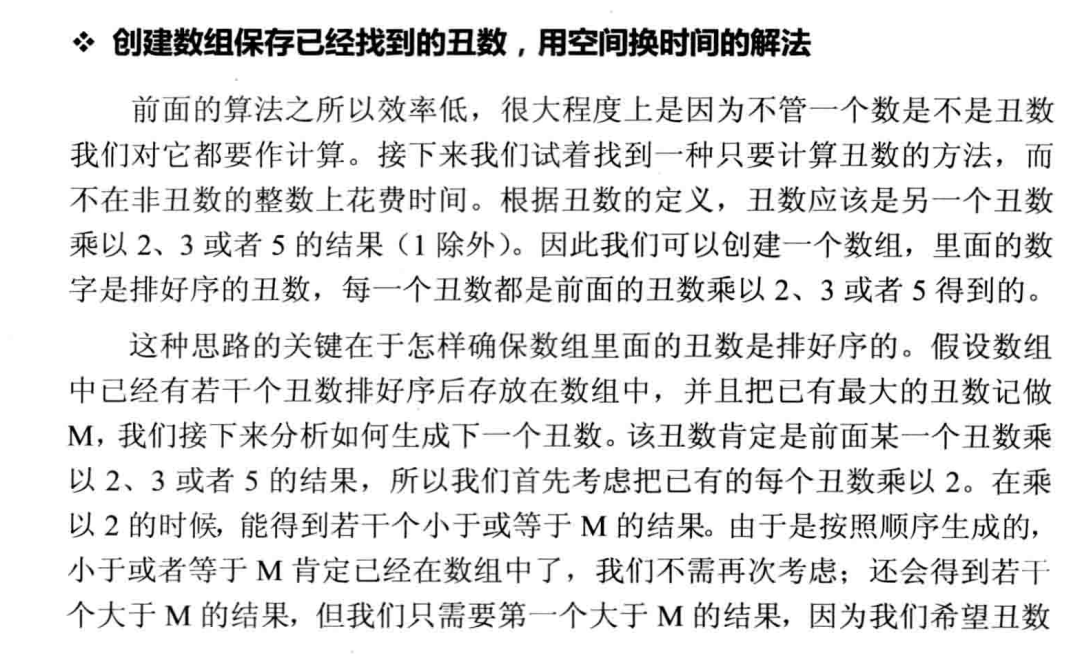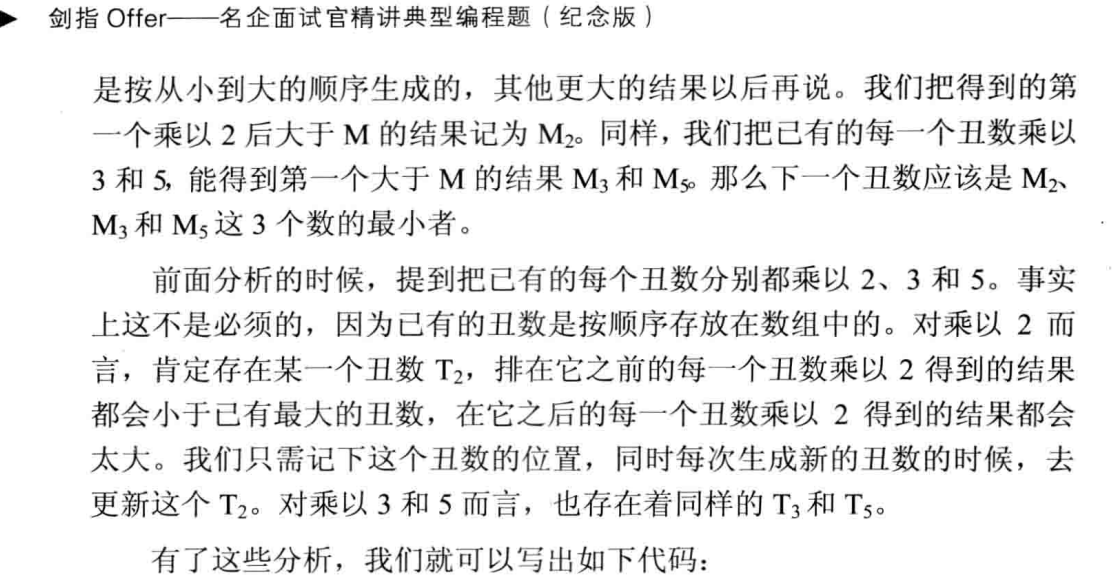public class Solution {
/**
* 剑指offer的思路：
* 取一个数组来放丑数，下一个丑数必定是这个数组里面的数乘以2或者3
* 或者5，这三种中最小的那一个。依次，判断下去
* @param index
* @return
*/
public int GetUglyNumber_Solution(int index) {
if(index <= 0)
return 0;
int [] res = new int[index];
res = 1;//先把1放入
int m2 = 0;//控制乘以2的位置，假设得到一个丑数是乘以2得到的，
//那么下一次就是数组中的下一个丑数可能达到。
int m3 = 0;
int m5 = 0;
for (int i = 1; i < index; i++) {
int min = Math.min(res[m2]*2, Math.min(res[m3]*3, res[m5]*5));
res[i] = min;//最小的那个作为当前的丑数
//判断是由谁乘以得到的
if(res[m2] *2 == min)//假设res是乘以2得到的丑数，那么下一次就要判断
//是否是res乘以2可能得到丑数，所以就要++
m2++;
if(res[m3]*3 == min)
m3++;
if(res[m5]*5 == min)
m5++;
}
return res[index - 1];
}
}

七、栈和队列

1、滑动窗口的最大值

给定一个数组和滑动窗口的大小，找出所有滑动窗口里数值的最大值。例如，如果输入数组{2,3,4,2,6,2,5,1}及滑动窗口的大小3，那么一共存在6个滑动窗口，他们的最大值分别为{4,4,6,6,6,5}； 针对数组{2,3,4,2,6,2,5,1}的滑动窗口有以下6个： {[2,3,4],2,6,2,5,1}， {2,[3,4,2],6,2,5,1}， {2,3,[4,2,6],2,5,1}， {2,3,4,[2,6,2],5,1}， {2,3,4,2,[6,2,5],1}， {2,3,4,2,6,[2,5,1]}。

思路：左程云的代码和思路，博客的代码其实可以用左的思路来说明。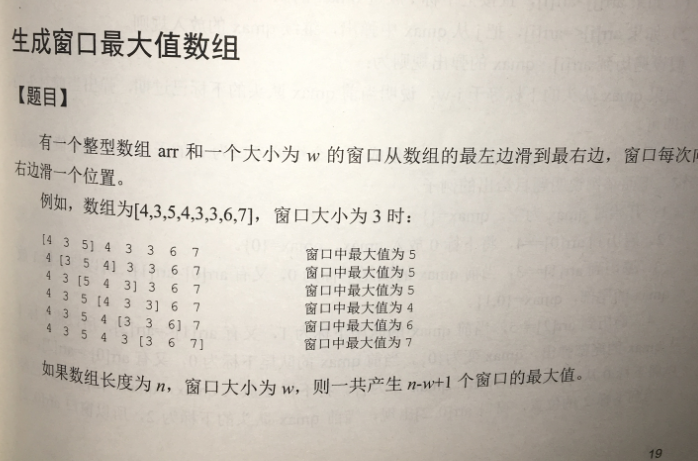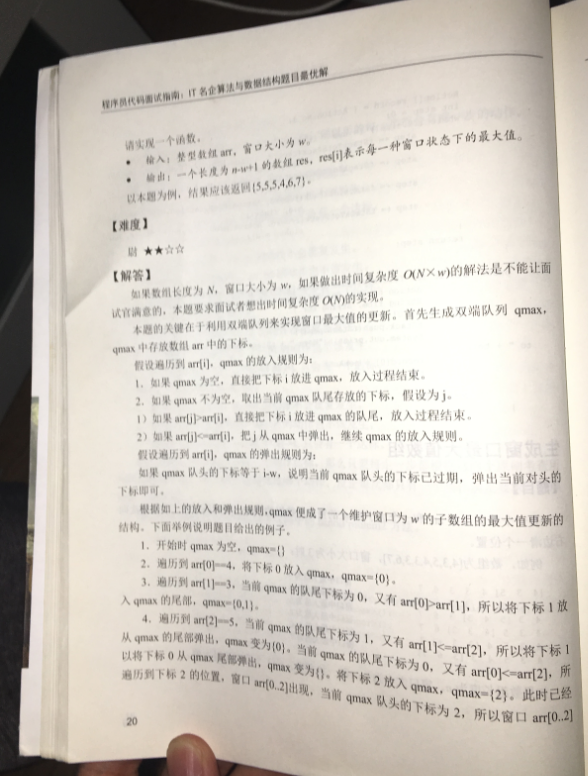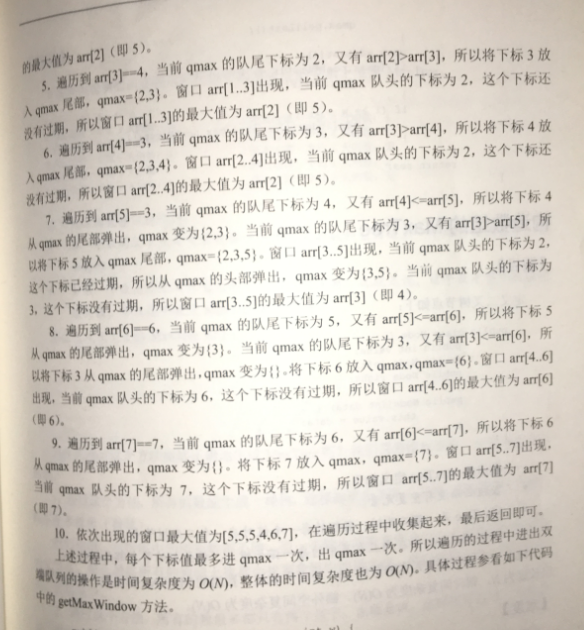public static ArrayList<Integer> maxInWindows(int[] num, int size) {
ArrayList<Integer> maxWindows = new ArrayList<>();
if (num == null || size == 0 || num.length == 0 || num.length < size)
return maxWindows;
for (int i = 0; i < size; i++) {

   // 如果已有数字小于待存入的数据，
// 如果已有数字小于待存入的数据，
// 这些数字已经不可能是滑动窗口的最大值
// 这些数字已经不可能是滑动窗口的最大值
// 因此它们将会依次地从队尾删除
while (!dq.isEmpty() && num[i] > num[dq.getLast()])
dq.removeLast();
}
//System.out.println(dq);
for (int i = size; i < num.length; i++) {
while (!dq.isEmpty() && num[i] >= num[dq.getLast()])
dq.removeLast();

if (!dq.isEmpty() && dq.getFirst() <= i - size)
dq.removeFirst();
System.out.println(i + "--" + dq);
}
return maxWindows;
}
// 因此它们将会依次地从队尾删除
while (!dq.isEmpty() && num[i] > num[dq.getLast()])
dq.removeLast();
}
//System.out.println(dq);
for (int i = size; i < num.length; i++) {
while (!dq.isEmpty() && num[i] >= num[dq.getLast()])
dq.removeLast();

if (!dq.isEmpty() && dq.getFirst() <= i - size)
dq.removeFirst();
System.out.println(i + "--" + dq);
}
return maxWindows;
}

左程云的代码：

思路：其实当有新的数来的时候，无论这个数是大于队尾还是小于队尾都要保存。只不过如果新来的大于队尾，说明队尾的数永远不会是大的数了，因为来了更大的，所以直接弹出就好了。如果新来的数小于队尾，说明队中的仍是大值，但是这个大的数值可能会过期，所以新来的小数仍然要保存。如下两个例子

a. 5 4 3 2 窗口是3，所以[5 4 3] 2第一个是5，如果新数4 和 3 不保存，当第二个窗口的时候，[4 3 2]就把4没有了

b.5 6 7 2窗口是3，要依次队尾中添加，最后剩7，前面的小，去掉就好了。

import java.util.ArrayList;

public static void main(String[] args) {
int num[] = { 2, 3, 4, 2, 6, 2, 5, 1 };
System.out.println(maxInWindows(num, 3));

}
//因为函数形式是这样的，其实因为返回的窗口最大值的数组一共就是n-w+1,不需要arraylist
public static  ArrayList<Integer> maxInWindows(int [] num, int size){
ArrayList<Integer> res = new ArrayList<>();
if(num == null || size <=0 || size > num.length)
return res;
for (int i = 0; i < num.length; i++) {
//如果新值大于队尾的，之前的那个队尾永远不是最大了，就直接弹出来就好了
while(!qmax.isEmpty() && num[qmax.peekLast()]<=num[i])
qmax.pollLast();
if(qmax.peekFirst() == i -size)//此时，下标已经过期，说明此时的窗口其实
//没有包含这个下标了
qmax.pollFirst();
if(i >= size-1)//保证一开始的不存入，假设3 2 1，
//只有下标大于窗口时候才判断加入此时的对头
}
return res;
}
}

2、用 两个栈实现队列

题目描述：用两个栈来实现一个队列，完成队列的Push和Pop操作。 队列中的元素为int类型。

思路：入栈给stack1，出栈时，若stack2不为空，则出栈，若为空，把stack1的内容全都放入stack2，然后再出栈

import java.util.Stack;

public class Solution {
Stack<Integer> stack1 = new Stack<Integer>();
Stack<Integer> stack2 = new Stack<Integer>();

public void push(int node) {
stack1.push(node);
}

public int pop() {
if(stack2.isEmpty()){
while(!stack1.isEmpty()){
stack2.push(stack1.pop());
}

}
return stack2.pop();
}
}

3、包含min函数的栈

定义栈的数据结构，请在该类型中实现一个能够得到栈中所含最小元素的min函数（时间复杂度应为O（1））。

思路：

import java.util.Stack;

public class Solution {

/*
* 思路：用一个栈stack保存数据，用另外一个栈min保存依次入栈最小的数
* 比如，stack中依次入栈，5, 4, 3, 8, 10,11,12,1
* 则min依次入栈，5, 4, 3, 3, 3, 3, 3, 1
* 每次入栈的时候，如果入栈的元素比min中的栈顶元素小或等于则入栈，否则入stack的栈顶元素。
* 保持stack中和min中保持相同个数的元素 ，同时保持min的栈顶是此时原栈的最小值。
*/
Stack<Integer> stackData = new Stack<>(); //声明时候的异同
Stack<Integer> stackMin = new Stack<Integer>();
public void push(int node) {
stackData.push(node);
// 如果min为空或者node比min栈中的元素小，则入min栈
if(stackMin.size() == 0 || stackMin.peek() > node)
stackMin.push(node);
else // 否则把min栈中的顶部元素重复入栈
stackMin.push(stackMin.peek());
}

public void pop() {//因为时刻保持两个栈的高度相同，所以两个都pop，时刻保持min的栈顶是原栈的最小值。
//如果返回应该是返回原栈的。
if(!stackData.isEmpty()){
stackData.pop();
stackMin.pop();
}
}

public int top() {
return stackData.peek();
}

public int min() {
return stackMin.peek();
}
}

4、栈的压入、弹出序列

输入两个整数序列，第一个序列表示栈的压入顺序，请判断第二个序列是否可能为该栈的弹出顺序。假设压入栈的所有数字均不相等。例如序列1,2,3,4,5是某栈的压入顺序，序列4,5,3,2,1是该压栈序列对应的一个弹出序列，但4,3,5,1,2就不可能是该压栈序列的弹出序列。（注意：这两个序列的长度是相等的）

import java.util.ArrayList;
import java.util.Stack;

public class Solution {
/**借用一个辅助的栈，遍历压栈顺序，先讲第一个放入栈中，这里是1，
然后判断栈顶元素是不是出栈顺序的第一个元素，这里是4，很显然1≠4，所以我们继续压栈，
直到相等以后开始出栈，出栈一个元素，则将出栈顺序向后移动一位，直到不相等，
这样循环等压栈顺序遍历完成，如果辅助栈还不为空，说明弹出序列不是该栈的弹出顺序。
举例：
入栈1,2,3,4,5
出栈4,5,3,2,1
首先1入辅助栈，此时栈顶1≠4，继续入栈2
此时栈顶2≠4，继续入栈3
此时栈顶3≠4，继续入栈4
此时栈顶4＝4，出栈4，弹出序列向后一位，此时为5，,辅助栈里面是1,2,3
此时栈顶3≠5，继续入栈5
此时栈顶5=5，出栈5,弹出序列向后一位，此时为3，,辅助栈里面是1,2,3
….
依次执行，最后辅助栈为空。如果不为空说明弹出序列不是该栈的弹出顺序。
* @param pushA
* @param popA
* @return
*/
public boolean IsPopOrder(int[] pushA, int[] popA) {
if (pushA == null || popA == null || pushA.length == 0
|| popA.length == 0)
return false;
int index = 0; //作为弹出序列的一个索引
Stack<Integer> stack = new Stack<Integer>();
for (int i = 0; i < pushA.length; i++) {
stack.push(pushA[i]);
while (!stack.isEmpty() && stack.peek() == popA[index]) {// 当栈不为空且栈顶元
//素等于弹出序列元素时候，就弹出一个，同时让弹出序列后移一个
stack.pop();
index++;
}
}
return stack.isEmpty();//如果最后，栈不为空，相当于没有按照给定的弹出popA弹出完毕，
//就说明不能按照popA，返回false
}

}

八、回溯法

1、矩阵中的路径

请设计一个函数，用来判断在一个矩阵中是否存在一条包含某字符串所有字符的路径。路径可以从矩阵中的任意一个格子开始，每一步可以在矩阵中向左，向右，向上，向下移动一个格子。如果一条路径经过了矩阵中的某一个格子，则之后不能再次进入这个格子。 例如 a b c e s f c s a d e e 这样的3 X 4 矩阵中包含一条字符串"bcced"的路径，但是矩阵中不包含"abcb"路径，因为字符串的第一个字符b占据了矩阵中的第一行第二个格子之后，路径不能再次进入该格子。

思路：回溯算法也叫试探法，它是一种系统地搜索问题的解的方法。回溯算法的基本思想是：从一条路往前走，能进则进，不能进则退回来，换一条路再试。首先，在矩阵中任选一个格子作为路径的二七店。假设矩阵中某个格子的字符为ch并且这个格子将对应于路径上的第i个字符。如果路径上的第i个字符不是ch，那么这个格子不可能处在路径上的第i个位置。如果路径上的第i个字符正好是ch，那么朝相邻的格子寻找路径上的第i+1个字符。除在边界上的格子之外，其他格子都有4个相邻的格子。重复这个过程直到路径上的所有字符都在矩阵中找到相应的位置。

由于回溯法的递归特性，路径可以看成一个栈。当矩阵中定位了路径中的前n个字符的位置之后，在与第n个字符对应的格子的周围都没有找到第n+1个字，需要在路径上会退到第n-1个字符，重新定位第n个字符。由于路径不能重复进入矩阵的格子，还需要定义和矩阵大小一样的布尔值矩阵，用来标识路径是否已经进入了每个格子。

public class Solution {
/**
* 判断字符矩阵是否包含某一个字符序列
* @param matrix
* @param rows  矩阵行数
* @param cols  矩阵列数
* @param str   目标字符序列
* @return
*/
public boolean hasPath(char[] matrix, int rows, int cols, char[] str) {
boolean visitFlags[] = new boolean[matrix.length];
for (int row = 0; row < rows; row++) {
for (int col = 0; col < cols; col++) {
if (hasPathCore(matrix, rows, cols, row, col, str, 0, visitFlags))
return true;
}
}

return false;
}

/**
* 回溯法递归实现判断
* @param matrix    字符矩阵
* @param rows  矩阵行数
* @param cols  矩阵列数
* @param row   当前行索引
* @param col   当前列索引
* @param str   目标字符序列
* @param k 目标字符序列中当前字符索引
* @param visitFlags    字符矩阵是否被访问过标记
* @return
*/
boolean hasPathCore(char[] matrix, int rows, int cols, int row, int col, char[] str,  int k, boolean[] visitFlags) {
int index = row * cols + col;
// 行列索引超限、当前字符已经被访问过、当前字符不等于目标字符序列的当前字符，直接返回false
if (row < 0 || col < 0 || row >= rows || col >= cols ||
visitFlags[index] || matrix[index] != str[k])
return false;

visitFlags[index] = true;   // 设置访问标记
if (k == str.length - 1)    // 递归结束条件，k已经到达目标字符序列的最后一个字符
return true;

k++;    // 匹配目标字符序列的下一个字符

// 在当前字符的上、下、左、右的元素搜索下一个目标字符，递归
if (hasPathCore(matrix, rows, cols, row + 1, col, str, k, visitFlags) ||
hasPathCore(matrix, rows, cols, row - 1, col, str, k, visitFlags) ||
hasPathCore(matrix, rows, cols, row, col + 1, str, k, visitFlags) ||
hasPathCore(matrix, rows, cols, row, col - 1, str, k, visitFlags))
return true;

// // 在当前字符的上、下、左、右的元素没有搜索到下一个目标字符，将访问标记重置为false，返回false；
visitFlags[index] = false;
return false;
}
}

2、机器人运动范围

地上有一个m行和n列的方格。一个机器人从坐标0,0的格子开始移动，每一次只能向左，右，上，下四个方向移动一格，但是不能进入行坐标和列坐标的数位之和大于k的格子。 例如，当k为18时，机器人能够进入方格（35,37），因为3+5+3+7 = 18。但是，它不能进入方格（35,38），因为3+5+3+8 = 19。请问该机器人能够达到多少个格子？

思路：利用递归实现，每次只能走上下左右四个点，进行判断点的位置是否越界，点数之和是否大于K，是否已经走过了。

public int movingCount(int threshold, int rows, int cols) {
int flag[][] = new int[rows][cols]; //记录是否已经走过
return helper(0, 0, rows, cols, flag, threshold);
}

private int helper(int i, int j, int rows, int cols, int[][] flag, int threshold) {
if (i < 0 || i >= rows || j < 0 || j >= cols ||
numSum(i) + numSum(j) > threshold || flag[i][j] == 1)
return 0;
flag[i][j] = 1;
return helper(i - 1, j, rows, cols, flag, threshold)
+ helper(i + 1, j, rows, cols, flag, threshold)
+ helper(i, j - 1, rows, cols, flag, threshold)
+ helper(i, j + 1, rows, cols, flag, threshold) + 1;
}

private int numSum(int i) {
int sum = 0;
while (i > 0) {
sum += i % 10;
i = i / 10;
}
return sum;
}

九、链表

1、从尾到头打印链表

输入一个链表，按链表值从尾到头的顺序返回一个ArrayList。

也可以用栈：

public class Solution {
if(listNode == null){
ArrayList list = new ArrayList();
return list;
}
Stack<Integer> stk = new Stack<Integer>();
while(listNode != null){
stk.push(listNode.val);
listNode = listNode.next;
}
ArrayList<Integer> arr = new ArrayList<Integer>();
while(!stk.isEmpty()){
}
return arr;
}
}

/**
*    public class ListNode {
*        int val;
*        ListNode next = null;
*
*        ListNode(int val) {
*            this.val = val;
*        }
*    }
*
*/
import java.util.ArrayList;
import java.util.Collections;
public class Solution {
if(listNode == null){
ArrayList first = new ArrayList();
return first;
}
while (listNode != null) {
listNode = listNode.next;

}

}
}


2、链表中倒数第k个结点

输入一个链表，输出该链表中倒数第k个结点。

/*
public class ListNode {
int val;
ListNode next = null;

ListNode(int val) {
this.val = val;
}
}*/
public class Solution {
public ListNode FindKthToTail(ListNode head,int k) {
//一种思路是先遍历一遍求长度，然后输出倒数k个
//正常的思路是，设置两个游标,让快的领先k个
if (head == null || k <= 0) {
return null;
}
for (int i = 1; i < k; i++) { //快的先走k-1步，倒数第三个，其实应该快的指到第三个，只需要走两步即可。
if(fast.next == null) //这个是k与链表长度的关系，如果，链表长度小于k，肯定在走到k之前就出现
//null，直接返回null即可
return null;
else
fast = fast.next;
}
while(fast.next != null){ //快的从第k个，慢的从第1个，同时开始走。
slow = slow.next;
fast = fast.next;
}
return slow;
}
}

3、反转链表

输入一个链表，反转链表后，输出新链表的表头。

/*
public class ListNode {
int val;
ListNode next = null;

ListNode(int val) {
this.val = val;
}
}*/
public class Solution {
return null;
ListNode pre = null;
ListNode next = null;
}
return pre;

}
}
/*
//反转链表
ListNode pre = null;
ListNode next = null;
// 即pre让节点可以反转所指方向，但反转之后如果不用next节点保存next1节点的话，此单链表就此断开了
// 所以需要用到pre和next两个节点
// 1->2->3->4->5
// 1<-2<-3->4->5
// 做循环，如果当前节点不为空的话，始终执行此循环，此循环的目的就是让当前节点从指向next到指向pre
// 如此就可以做到反转链表的效果
}
return pre;
}
//合并两个递增的链表并且保证最终的链表也是单调不减的。
*/

4、合并两个排序的链表

题目描述

输入两个单调递增的链表，输出两个链表合成后的链表，当然我们需要合成后的链表满足单调不减规则。

思路：输入两个单调递增的链表，输出两个链表合成后的链表，当然我们需要合成后的链表满足单调不减规则。
比较两个链表的首结点，哪个小的的结点则合并到第三个链表尾结点，并向前移动一个结点。
步骤一结果会有一个链表先遍历结束，或者没有
第三个链表尾结点指向剩余未遍历结束的链表
返回第三个链表首结点

/*
public class ListNode {
int val;
ListNode next = null;

ListNode(int val) {
this.val = val;
}
}*/
public class Solution {
public ListNode Merge(ListNode list1,ListNode list2) {
if (list1 == null) {
return list2;
}
if (list2 == null) {
return list1;
}
//新建一个用于存放融合之后的链表
//因为，融合的过程中链表是一直移动的，所以要把链表的头保存下来，才能返回正确的一条链
ListNode res = new ListNode(0);  //此处或者res = null，这样就不是res.next 而是res
ListNode merlistNode = res;
while (list1 != null && list2 != null) { //依次比较，将较小的节点连到融合节点上
if(list1.val < list2.val){
merlistNode.next = list1;      //连上小的list1
list1 = list1.next;         //list1 可以往后移动一个，下次用移动后的和list2比较
merlistNode = merlistNode.next;// merlistNode也往后移动一个
}

else {
merlistNode.next = list2;
list2 = list2.next;
merlistNode = merlistNode.next;
}

}
//把未结束的链表连接到合并后的链表尾部
if(list1 != null)
merlistNode.next = list1;
if(list2 != null)
merlistNode.next = list2;
return res.next;
}
//递归的方式
/*public ListNode Merge(ListNode list1,ListNode list2) {
if (list1 == null) {
return list2;
}
if (list2 == null) {
return list1;
}
if (list1.val <= list2.val) {
}else {
}

}  */
}

5、复杂链表的复制

题目描述

/*
public class RandomListNode {
int label;
RandomListNode next = null;
RandomListNode random = null;

RandomListNode(int label) {
this.label = label;
}
}
*/
import java.util.HashMap;
public class Solution {
/**左程云思路：除了这个还有不用链表的思路。
* 算法步骤：遍历两遍链表，第一遍将仅仅将数赋值给map中的值，第二遍将指针指向赋值。注意保存头指针的位置。
* 1.第一遍遍历，key是第一个链表中的节点，value是复制后的链表节点，但是指针都指向null。
* 2.第二遍遍历，将相对应的next和random均复制。
* @return
*/
{
HashMap<RandomListNode, RandomListNode> map =new HashMap<RandomListNode, RandomListNode>();
while (current != null) {//第一遍遍历
map.put(current,new RandomListNode(current.label));// hashmap里面，key放的是之前的链表节点，value现在只放值
current = current.next;
}
while (current != null) {//第二遍遍历
//现在map中是1--1'  2--2'。为了让1'指向2'  要给1'的next赋值， 要找1'就得get(1)。值是2',要找2'就是get(1.next)
map.get(current).next = map.get(current.next);
map.get(current).random = map.get(current.random);
current = current.next;
}
}
}

6、两个链表的第一个公共结点

题目描述

输入两个链表，找出它们的第一个公共结点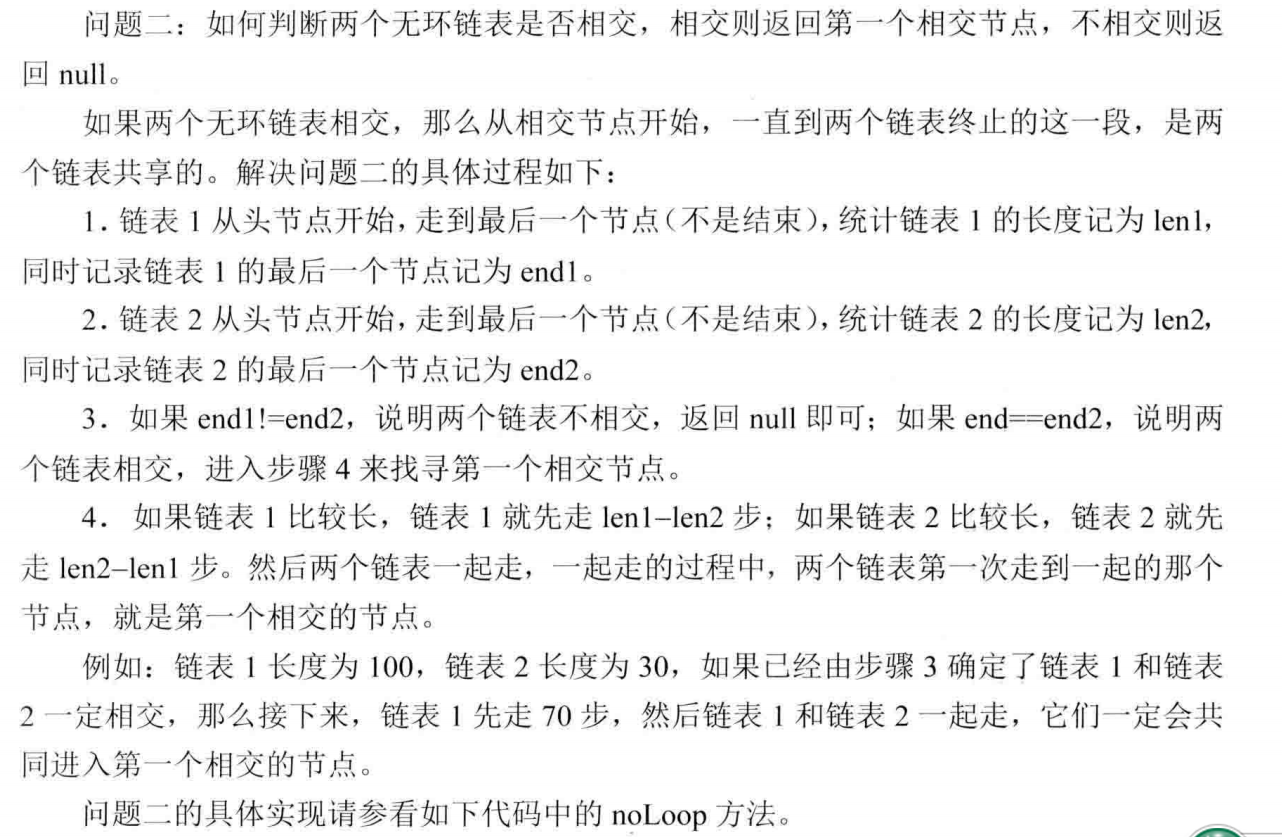/*
public class ListNode {
int val;
ListNode next = null;

ListNode(int val) {
this.val = val;
}
}*/
public class Solution {

/**采用了左程云的代码思想：
* 首先，如果相交，那么尾节点一定是一样的。
* 接下来，谁长谁就先走一定的步数，然后一起走，肯定是同时到达相交的点。
* @return
*/
return null;
int n = 0;
while(cur1.next != null) {
n++; //记录长度
cur1 = cur1.next;
}
while(cur2.next != null) {
n--;
cur2 = cur2.next;
}
if(cur1 != cur2)
return null;
n= Math.abs(n);
while(n !=0 ) {
n--;    //先cur1走完这部分
cur1 = cur1.next;
}
while(cur1 != cur2) {
cur1 = cur1.next;
cur2 = cur2.next;
}
return cur1;
}
}

7、链表中环的入口节点

给一个链表，若其中包含环，请找出该链表的环的入口结点，否则，输出null。

思路：

第一步，找环中相汇点。分别用p1，p2指向链表头部，
* p1每次走一步，p2每次走二步，直到p1==p2找到在环中的相汇点。

通过141题，我们知道可以通过快慢指针来判断是否有环，现在我们假设两个指针相遇在z点，如图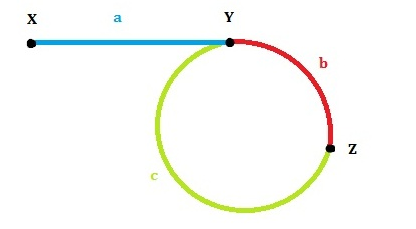那么我们可以知道fast指针走过a+b+c+b

slow指针走过a+b

那么2*(a+b) = a+b+c+b

所以a = c

那么此时让slow回到起点，fast依然停在z，两个同时开始走，一次走一步

那么它们最终会相遇在y点，正是环的起始点

/*
public class ListNode {
int val;
ListNode next = null;

ListNode(int val) {
this.val = val;
}
}
*/
public class Solution {
/**
* 主要思路就是一快 一慢两个指针，如果有环，最终快的肯定能追上慢的，
* 找环的入口的思路见博客。
* @return
*/
{
return null;
}
while(fast != null && fast.next != null) {//因为fast每次要走两步，所有需要判断fast的下一个是否为空
slow = slow.next;
fast = fast.next.next;//一个走一步 一个走两步
if(slow == fast) {
while(slow != fast) {
slow = slow.next;
fast = fast.next;
}
return slow;
}
}
return null;
}
}

8、删除链表中重复的节点

题目描述

在一个排序的链表中，存在重复的结点，请删除该链表中重复的结点，重复的结点不保留，返回链表头指针。 例如，链表1->2->3->3->4->4->5 处理后为 1->2->5

重点是第一个也可能是重复的点，因此新建一个preNode节点保存前一个节点

/*
public class ListNode {
int val;
ListNode next = null;

ListNode(int val) {
this.val = val;
}
}
*/
public class Solution {
/**
* 主要参考博客的解题思路
* @return
*/
{
return null;
// 新建一个节点，防止头结点被删除
ListNode firstNode = new ListNode(-1);
// 指向前一个节点
ListNode preNode = firstNode;
while (p!=null &&p.next !=null) {//注意条件的顺序，否则不对 因为如果p为null，p.next肯定异常
if(p.val == p.next.val) {
int val = p.val;
// 向后重复查找
while (p != null&&p.val == val) {
p = p.next;
}
// 上个非重复值指向下一个非重复值：即删除重复值
preNode.next = p;
}
else {
// 如果当前节点和下一个节点值不等，则向后移动一位
preNode = p;
p = p.next;
}
}
return firstNode.next;

}
}

9、链表回文结构--左神

十、非剑指offer

1、 左神的

给定一个字符串str，str表示一个公式，公式里可能有整数，加减乘除符号和左右括号，返回公式计算的结果。例如，str = 48×((70-65)-43)+8×1。返回-1816。

【说明】

可以认定给定的字符串一定是正确的公式，不需要对str做公式有效性检查。

如果是负数就需要有括号括起来，比如4*(-3)。但如果负数作为公式的开头或者括号部分的开头，则可以没有括号，比如-3*4和（-3*4）都是合法的。

不需要考虑溢出问题
import java.util.LinkedList;

/**
* 算法顺序大致如下：
*                     保证其中没有加减乘除导致无法知道优先级。
*                  3、执行getNum 计算队列中的加减法，没有优先级问题，可以直接进行运算即可。
* 有括号的情况下：1、执行value，按上述第一步执行，碰到括号，则进入递归，去按上述所有的步骤计算括号里面的。
*                  2、剩下的按照上边的算法继续计算。
* Created by shixi_shengzhi on 2018/9/9
*/
public class Main {

public static int getValue(String str) {
return value(str.toCharArray(), 0);
}

public static int[] value(char[] str, int i) {
int pre = 0;
int[] bra = null;
while (i < str.length && str[i] != ')') {
if (str[i] >= '0' && str[i] <= '9') {
pre = pre * 10 + str[i++] - '0';
} else if (str[i] != '(') { //+ - * / ，每当碰到运算符号就先把里面的乘除法运算完，保证队列里面都是加减法
pre = 0;
} else { // 碰到符号（
bra = value(str, i + 1); //重新递归上述过程。返回括号中的结果以及位置
pre = bra;
i = bra + 1;
}
}
//公式执行完或者碰到 )
return new int[]{getNum(que), i}; //计算公式中还保留的加减法
}

/**
* 计算乘除法，把乘除法的结果还有加减号在放在队列中。
*
* @param que
* @param num
*/
if (!que.isEmpty()) {
int cur = 0;
String top = que.pollLast();
if (top.equals("+") || top.equals("-")) {
} else {
cur = Integer.valueOf(que.pollLast());
num = top.equals("*") ? (cur * num) : (cur / num);
}
}
}

/**
* 计算队列中的加减法
*
* @param que
* @return
*/
public static int getNum(LinkedList<String> que) {
int res = 0;
String cur = null;
int num = 0;
while (!que.isEmpty()) {
cur = que.pollFirst();
if (cur.equals("+")) {
} else if (cur.equals("-")) {
} else {
num = Integer.valueOf(cur);
res += add ? num : (-num);
}
}
return res;
}

public static void main(String[] args) {
String exp = "48*((70-65)-43)+8*1";
exp = "3+5*6";
exp = "3+4*5+6";
System.out.println(getValue(exp));
//        System.out.println(getValue(exp));
//
//        exp = "4*(6+78)+53-9/2+45*8";
//        System.out.println(getValue(exp));
//
//        exp = "10-5*3";
//        System.out.println(getValue(exp));
//
//        exp = "-3*4";
//        System.out.println(getValue(exp));
//
//        exp = "3+1*4";
//        System.out.println(getValue(exp));

}

}



展开全文• 牛客剑指offer １．二维数组查找 题目描述 在一个二维数组中（每个一维数组的长度相同），每一行都按照从左到右递增的顺序排序，每一列都按照从上到下递增的顺序排序。请完成一个函数，输入这样的一个二维数组和一个...
牛客剑指offer
１．二维数组查找
题目描述
在一个二维数组中（每个一维数组的长度相同），每一行都按照从左到右递增的顺序排序，每一列都按照从上到下递增的顺序排序。请完成一个函数，输入这样的一个二维数组和一个整数，判断数组中是否含有该整数。
思路
可以从左下开始进行查找，i代表行,j代表列，如果当前的array[i][j]大于target则说明需要找更小的，i-1上移一位得到的就是更小的值。如果当前的array[i][j]小于target则说明需要找更大的，j+1右移一位得到的就是更大的。直到i等于0,j等于array的宽度代表找完了。时间复杂度为m+n。
class Solution {
public:
bool Find(int target, vector<vector<int> > array) {
if(array.size()==0) return false;
int j=0;
int i=array.size()-1;
int edge=array.size()-1;
while (i>=0&&j<=edge){
if(array[i][j]==target){
return true;
}
else if(array[i][j]<target){
j++;
}
else{
i--;
}
}
return false;
}
};

２.替换空格
题目描述
请实现一个函数，将一个字符串中的每个空格替换成“%20”。例如，当字符串为We Are Happy.则经过替换之后的字符串为We%20Are%20Happy。
思路
替换如果是从前往后的替换的话，则插入一位，之后的所有都需要后移一位，显然操作起来比较复杂。所以还是从后往前的替换。要注意函数中的length是限制新的字符串的最大长度。
class Solution {
public:
void replaceSpace(char *str,int length) {
if(str==NULL||length<=0)
return;
//统计字符串中的空字符的个数
int blanknum=0;//空格字符数量
int newlen=0;//定义新的字符串长度
int oldlen=0;
for(int i=0;str[i]!='\0';i++)
{
oldlen++;
if(str[i]==' ')
blanknum++;
}
newlen=oldlen+2*blanknum;//新的字符串长度
//因为原先有个空格，所以只需要乘以2，不是3
//替换空格字符
//需要判断新的字符串长度是否大于oldlen
if(newlen>length)//length是给的数组最大长度
return;
for(int i=oldlen;i>=0;i--)
{
//小于的话进行替换
if(str[i]!=' ')
str[newlen--]=str[i];
else
{
str[newlen--]='0';
str[newlen--]='2';
str[newlen--]='%';
}

}
}
};

３．从尾到头打印链表
题目描述
输入一个链表，按链表从尾到头的顺序返回一个ArrayList。
思路
这个很简单从头到位遍历链表，然后使用vector来存储返回的值，插入的时候一律插在vector的起始位置。
/**
*  struct ListNode {
*        int val;
*        struct ListNode *next;
*        ListNode(int x) :
*              val(x), next(NULL) {
*        }
*  };
*/
class Solution {
public:
vector<int> result;
while(p!=NULL){
vector<int>::iterator b=result.begin();
result.insert(b,p->val);
p=p->next;
}
return result;
}
};


４．重建二叉树
题目描述
输入某二叉树的前序遍历和中序遍历的结果，请重建出该二叉树。假设输入的前序遍历和中序遍历的结果中都不含重复的数字。例如输入前序遍历序列{1,2,4,7,3,5,6,8}和中序遍历序列{4,7,2,1,5,3,8,6}，则重建二叉树并返回。
思路
二叉树最基础的知识，通过前序遍历找根节点，在中序遍历中找到index，根据index分出左子树和右子树，再进行递归。
/**
* Definition for binary tree
* struct TreeNode {
*     int val;
*     TreeNode *left;
*     TreeNode *right;
*     TreeNode(int x) : val(x), left(NULL), right(NULL) {}
* };
*/
class Solution {
public:
TreeNode* reConstructBinaryTree(vector<int> pre,vector<int> vin) {
//递归的结束条件
if(pre.empty()||vin.empty()){
return nullptr;
}
//寻找根节点在中序遍历中的index 好分左子树和右子树
int root_index=0;
for(int i=0;i<vin.size();++i){
if(pre==vin[i]){
root_index=i;
break;
}
}
//根据上面找到的index分子树
vector<int> Lpre,Rpre,Lvin,Rvin;
for (int i=0;i<root_index;++i){
Lpre.push_back(pre[i+1]);
Lvin.push_back(vin[i]);
}
for(int j=root_index+1;j<vin.size();++j){
Rpre.push_back(pre[j]);
Rvin.push_back(vin[j]);
}
//进行递归操作
}
};

５．用两个栈实现队列
题目描述
用两个栈来实现一个队列，完成队列的Push和Pop操作。 队列中的元素为int类型。
思路
思路如下图所示，非常好理解了。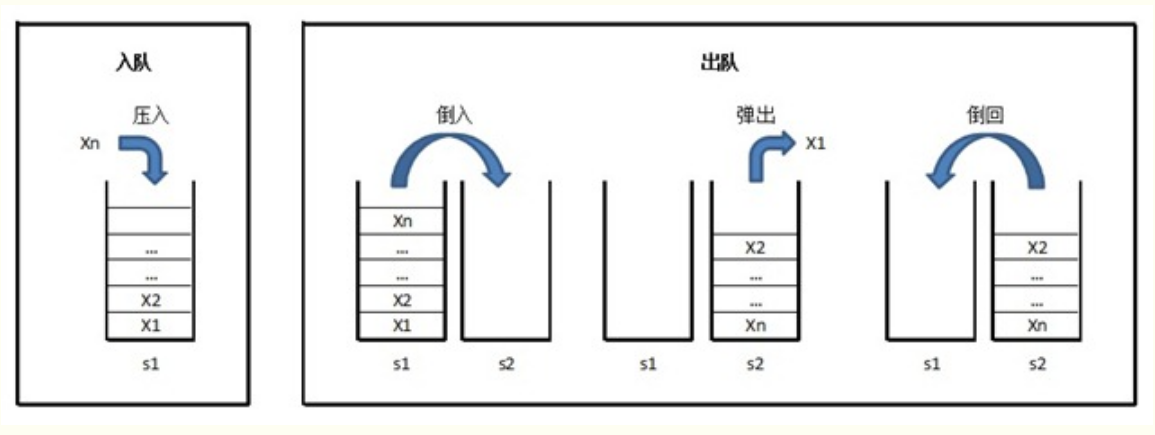class Solution
{
public:
//压入的时候以stack1
void push(int node) {
stack1.push(node);
}
int pop() {
int n=stack1.size();
for(int i=0;i<n;i++){
stack2.push(stack1.top());
stack1.pop();
}
int result=stack2.top();
stack2.pop();
int m=stack2.size();
for(int i=0;i<m;i++){
stack1.push(stack2.top());
stack2.pop();
}
return result;
}
private:
stack<int> stack1;
stack<int> stack2;
};

６．旋转数组的最小数字
题目描述
把一个数组最开始的若干个元素搬到数组的末尾，我们称之为数组的旋转。输入一个非递减排序的数组的一个旋转，输出旋转数组的最小元素。例如数组{3,4,5,1,2}为{1,2,3,4,5}的一个旋转，该数组的最小值为1。NOTE：给出的所有元素都大于0，若数组大小为0，请返回0。
思路
用两个指针来进行查找，首先第一个指针指向数组头。第二个指针指向数组尾。第一个指针总是指向前面递增数组的元素，而第二个指针总是指向后面递增数组的元素。最终第一个指针将指向前面子数组的最后一个元素，而第二个指针会指向后面子数组的第一个元素。也就是它们最终会指向两个相邻的元素，而第二个指针指向的刚好是最小的元素。这就是循环结束的条件。数组用a来表示，指针移动的逻辑为:若a[left]<=a[mid]则说明a[mid]的位置还在前一个递增序列中，则可以令left=mid;若a[right]>=a[mid]，则说明a[mid]的位置还在第二个递增序列中，则可以令right=mid
下图展示了在该数组中查找最小值的过程：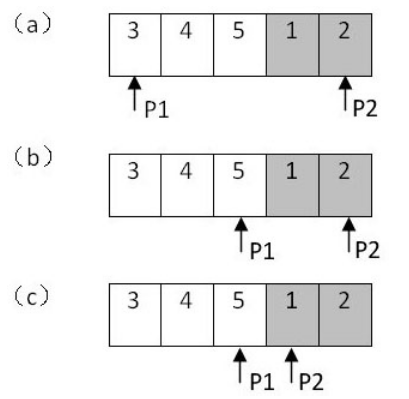class Solution {
public:
int minNumberInRotateArray(vector<int> rotateArray) {
int i=0,j=rotateArray.size()-1,m=0;
while (j-i>1){
m=(i+j)/2;
if(rotateArray[i]<=rotateArray[m]){
i=m;
}
if(rotateArray[j]>=rotateArray[m]){
j=m;
}
}
return rotateArray[j];
}
};

７．斐波那契数列
题目描述
大家都知道斐波那契数列，现在要求输入一个整数n，请你输出斐波那契数列的第n项（从0开始，第0项为0）。
n<=39
思路
非常简单了　就是第n项的值等于第n-1加 n-2的值。1,1,2,3,5,8,13…
int Fibonacci(int n) {
if(n==0){
return 0;
}
if(n==1){
return 1;
}
return Fibonacci(n-1)+Fibonacci(n-2);

}

以上的写法是使用递归的思想写的，写起来和清楚明了，但是这样子写是不能AC的，算法的复杂度过大。所以我们不应该使用递归的想法写，而应该用循环写。
class Solution {
public:
int Fibonacci(int n) {
int a=0,b=1,c=1;
if(n==0)return 0;
else if(n==1) return 1;
else {
for (int i = 2; i <=n; i++) {
c=a+b;
a=b;
b=c;
}
return c;
}
}
};

８．跳台阶
题目描述
一只青蛙一次可以跳上1级台阶，也可以跳上2级。求该青蛙跳上一个n级的台阶总共有多少种跳法（先后次序不同算不同的结果）。
思路
这个其实还是可以用递归的思想来，每次条台阶可以有两种跳法，跳一次之后还剩下n-1个台阶，则又变成和跳台阶一样的问题。跳两级之后还剩n-2个台阶，同样也变成和跳台阶一样的问题。则f(n)=f(n-1)+f(n-2)
class Solution {
public:
int jumpFloor(int number) {
if(number==2){
return 2;
}
else if(number==1){
return 1;
}
return jumpFloor(number-1)+jumpFloor(number-2);
}
};

９．变态跳台阶
题目描述
一只青蛙一次可以跳上1级台阶，也可以跳上2级……它也可以跳上n级。求该青蛙跳上一个n级的台阶总共有多少种跳法。
思路
用Fib(n)表示青蛙跳上n阶台阶的跳法数，青蛙一次性跳上n阶台阶的跳法数1(n阶跳)，
设定Fib(0) = 1；
当n = 2 时， 有两种跳的方式，一阶跳和二阶跳：Fib(2) = Fib(1) + Fib(0) = 2;
当n = 3 时，Fib(3) = Fib(2) + Fib(1)+Fib(0)=4;
当n = n 时，Fib(n) = Fib(n-1)+Fib(n-2)+Fib(n-3)+…+Fib(n-n)=Fib(0)+Fib(1)+Fib(2)+…+Fib(n-1)
又因为Fib(n-1)=Fib(0)+Fib(1)+Fib(2)+…+Fib(n-2)
两式相减得：Fib(n)-Fib(n-1)=Fib(n-1) －－－－－－>Fib(n) = 2*Fib(n-1)     n >= 2
因此递归等式如下：
$\operatorname{Fib}(n)=\left\{\begin{array}{ll}{1} & {n=0} \\ {1} & {n=1} \\ {2 * F i b(n-1)} & {n>2}\end{array}\right.$
class Solution {
public:
int jumpFloorII(int number) {
if (number == 1) {
return 1;
}
return 2*jumpFloorII(number-1);
}
};

１０．矩形覆盖
题目描述
我们可以用21的小矩形横着或者竖着去覆盖更大的矩形。请问用n个21的小矩形无重叠地覆盖一个2*n的大矩形，总共有多少种方法？
思路
n=1 - 只有横放一个矩形一种解决办法
n=2 - 有横放一个矩形，竖放两个矩形两种解决办法
n=3 - n=2的基础上加1个横向，n=1的基础上加2个竖向
n=4 - n=3的基础上加1个横向，n=2的基础上加2个竖向
…
f(n)=f(n-1)+f(n-2)
class Solution {
public:
int rectCover(int number) {
if (number == 0)
return 0;
else if(number == 1){
return 1;
}
else if (number == 2){
return 2;
}
return rectCover(number - 1) + rectCover(number - 2);
}
};

１１．二进制中１的个数
题目描述
输入一个整数，输出该数二进制表示中1的个数。其中负数用补码表示
思路
如果一个整数不为0，那么这个整数至少有一位是1。如果我们把这个整数减1，那么原来处在整数最右边的1就会变为0，原来在1后面的所有的0都会变成1(如果最右边的1后面还有0的话)。其余所有位将不会受到影响。我们将原来的n和ｎ-1做&操作，则１的个数就会减一。一直做这样的操作，直到相与的结果为０。
class Solution {
public:
int  NumberOf1(int n) {
if(n==0) return 0;
int i=0;
while (n){
i++;
n=n&(n-1);
}
return i;
}
};

１２．数值的整数次方
题目描述
给定一个double类型的浮点数base和int类型的整数exponent。求base的exponent次方。保证base和exponent不同时为0
思路
这个题目非常简单，循环连乘，注意一下特殊的情况
class Solution {
public:
double Power(double base, int exponent) {
double result=0.0;
if(fabs(base-0)<0.000001){
return result;
}
else if(exponent==0){
return 1.0;
}
else if(exponent<0){
int n=-exponent;
result=1;
for(int i=0;i<n;i++){
result=result*base;
}
result=1/result;
return result;
}
else if(exponent>0){
result=1;
for(int i=0;i<exponent;i++){
result=result*base;
}
return result;
}
}
};

１３．调整数组顺序使奇数位于偶数前面
题目描述
输入一个整数数组，实现一个函数来调整该数组中数字的顺序，使得所有的奇数位于数组的前半部分，所有的偶数位于数组的后半部分，并保证奇数和奇数，偶数和偶数之间的相对位置不变。
思路
这里可以参考快排的思想，快速排序的基本思想是：通过一趟排序将待排记录分割成独立的两部分，其中一部分记录的关键字均比另一部分记录的关键字小，则可分别对这两部分记录继续进行排序，以达到整个序列有序的目的。因此，我们可以借鉴快速排序的思想，通过设置两个指针来进行交换操作，从而减少移动次数，提高效率：

Step第一个指针初始化时指向数组的第一个数字，它只向后移动；
Step第二个指针初始化时指向数组的最后一个数字，它只向前移动。
Step在两个指针相遇之前，第一个指针总是位于第二个指针的前面。如果第一个指针指向的数字是偶数，并且第二个指针指向的数字是奇数，我们就交换这两个数字。

下图展示了调整数组{1,2,3,4,5}使得奇数位于偶数前面的过程：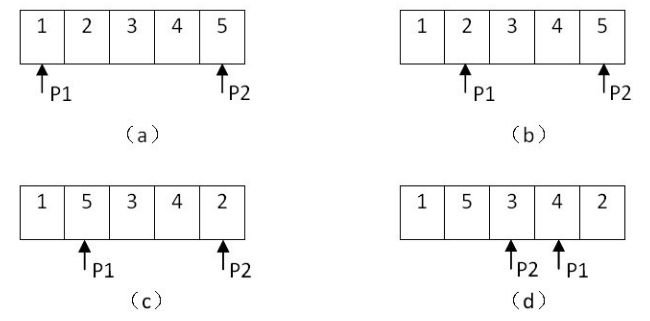class Solution {
public:
void reOrderArray(vector<int> &array) {
if(array.size()==0){
return;
}
int i=0,j=array.size()-1;
while (i!=j){
if(array[i]%2==0&&array[j]%2==1){
int temp;
temp=array[i];
array[i]=array[j];
array[j]=temp;
i++;
j--;
}
else if(array[i]%2==1){
i++;
}
else if(array[j]%2==0){
j--;
}
}
}
};

然而这样子写不能通过。因为快排是不稳定的，不能保证调整之后偶数和偶数，奇数与奇数之间的相对位置不变。
正解：从前往后找偶数，找到之后找这个偶数后边第一个奇数，每当找到前面的偶数和后面的奇数之后，那么把之间的部分（包括那个偶数）统一后移一位， 因为之间的都是偶数（从找到的偶数后面开始找到的第一个奇数和开始找到的偶数之间肯定都是偶数了），之前又没有偶数了（因为找的是从左往右第一个偶数），把他们统一后移一位，这样把提前保存起来的那个奇数放到前边空出来的那一位。这样相当于132457把5移动到24这两个偶数的前面，1 和5本来就是前后顺序的。这样堡整理调整之后的奇偶各自的相对顺序不会变。
class Solution {
public:
void reOrderArray(vector<int> &array) {
if(array.size()==0){
return;
}
int i=0,j=0;
while (i<array.size()){
if(array[i]%2==1){ //是奇数就后移
i++;
}
else if(array[i]%2==0){
j=i+1;
for (j;j<array.size();j++){ //找在这个偶数之后的第一个奇数
if(array[j]%2==1){
break;
}
}
if (j==array.size()) break;//如果之后都没有奇数的话，说明所有的排序完成
int tmp=array[j];
for(int k=j-1;k>=i;k--){//偶数包括奇数和偶数之间的数都后移一位
array[k+1]=array[k];
}
array[i]=tmp;
}
}
}
};

１４．链表中倒数第k个结点
题目描述
输入一个链表，输出该链表中倒数第k个结点。
思路
注意这个题目是返回节点，而不是返回值。返回值的话可以用栈来存储。返回节点则不能这样做。
设置两个指针，先让第一个指针移动k-1次。然后两个指针同时移动，当第一个指针到达最后一个节点，第二个指针就在倒数第k个节点。
注意边界：K长度可能超出链表长度，所以当第一个指针的next为空时，返回null
class Solution {
public:
ListNode* FindKthToTail(ListNode* pListHead, unsigned int k) {
return NULL;
for(int i=0;i<k;i++){
return NULL;
}
}
find=find->next;
}
return find;

}
};

１５．反转链表
题目描述
输入一个链表，反转链表后，输出新链表的表头。
思路
很简单，边遍历原来的链表，边创建新链表，新链表使用前插法插入。
    ListNode* ReverseList(ListNode* pHead) {
}
while (p!=NULL){
ListNode *tmp=new ListNode(p->val);
tmp->next=revers;
revers=tmp;
p=p->next;
}
return revers;
}

这样子会开创更多的空间，更好的方法是使用三个指针的遍历操作来完成。
class Solution {
public:
}
ListNode *pre = NULL; // 当前节点的前一个节点
ListNode *next = NULL; // 当前节点的下一个节点
pre = pHead; // pre 往右走
}
return pre;
}
};

１６．合并两个排序的链表
题目描述
输入两个单调递增的链表，输出两个链表合成后的链表，当然我们需要合成后的链表满足单调不减规则。
思路
这也是一个很简单的题目了
/*
struct ListNode {
int val;
struct ListNode *next;
ListNode(int x) :
val(x), next(NULL) {
}
};*/
class Solution {
public:
{
ListNode *merge=new ListNode(0),*p=merge;
p=p->next;
}
else{
p=p->next;
}
}
p=p->next;
}
}
p=p->next;
}
}
return merge->next;
}
};

１９．树的子结构
题目描述
输入两棵二叉树A，B，判断B是不是A的子结构。（ps：我们约定空树不是任意一个树的子结构）
思路
想要判断B是不是A的子结构，我们需要去依次遍历比较双方的结点value。这边采用的是递归的思想来解决该问题。
首先将A的根节点与B的根节点比较，
１．若不相等，则继续扫描A的左右子树，
×Ａ的左子树，与B的根节点比较，
＋若该子树的根节点与B的根节点相等，则继续遍历该子树的左右子树与Ｂ的左右子树是否相等
＋若不相等，则继续扫描A的子树结点，直到所有结点扫描结束
×Ａ的右子树，与B的根节点比较，
＋若该子树的根节点与B的根节点相等，则继续遍历该子树的左右子树与Ｂ的左右子树是否相等
＋若不相等，则继续扫描A的子树结点，直到所有结点扫描结束
２．若相等，则双方各自分别往其左右子树方向走；判断左右子树结点是否相等
所以代码如下：
/*
struct TreeNode {
int val;
struct TreeNode *left;
struct TreeNode *right;
TreeNode(int x) :
val(x), left(NULL), right(NULL) {
}
};*/
class Solution {
public:
bool HasSubtree(TreeNode* pRoot1, TreeNode* pRoot2)
{
if(pRoot1==NULL||pRoot2==NULL){
return false;
}
bool flag= false;
if(pRoot1->val==pRoot2->val) {//根结点相等,比较左右子树
flag=IsSubtree(pRoot1, pRoot2);
}
if(!flag){
flag= HasSubtree(pRoot1->left,pRoot2);//根节点不相同的时候，找左子树
}
if(!flag){
flag= HasSubtree(pRoot1->right,pRoot2);//左子树也没有找到的话，继续找右子树
}
return flag;

}
bool IsSubtree(TreeNode *pRoot1,TreeNode *pRoot2){
if(pRoot2==NULL){//如果子树都遍历完说明都找到了
return true;
}
if(pRoot1==NULL){//如果母树遍历完　说明没有子树结构
return false;
}
if(pRoot1->val==pRoot2->val){//当根节点一样的时候，继续递归，不是就返回false
return IsSubtree(pRoot1->left,pRoot2->left)&&IsSubtree(pRoot1->right,pRoot2->right);
}
else{
return false;
}

}
};

１８．二叉树的镜像
题目描述
操作给定的二叉树，将其变换为源二叉树的镜像。
二叉树的镜像定义：
源二叉树
8
/  \
6   10
/ \  / \
5  7 9 　11
镜像二叉树
8
/  \
10   6
/ \  / \
11 9 7  5

思路
就是将根结点的左右子树交换，写的时候，应该写交换指针的指向位置，而不是交换左右子树的值。然后依次递归。
/*
struct TreeNode {
int val;
struct TreeNode *left;
struct TreeNode *right;
TreeNode(int x) :
val(x), left(NULL), right(NULL) {
}
};*/
class Solution {
public:
void Mirror(TreeNode *pRoot) {
if(pRoot==NULL||pRoot->right==NULL&&pRoot->left==NULL){
return;
}
TreeNode *tmp;
tmp=pRoot->left;//记录下来左子树的指向位置
pRoot->left=pRoot->right;//左子树指向右子树
pRoot->right=tmp;//右子树指向左子树

Mirror(pRoot->left); //递归左子树
Mirror(pRoot->right);//递归右子树
}
};

１９．顺时针打印矩阵
题目描述
输入一个矩阵，按照从外向里以顺时针的顺序依次打印出每一个数字，例如，如果输入如下4 X 4矩阵： 1 2 3 4 5 6 7 8 9 10 11 12 13 14 15 16 则依次打印出数字1,2,3,4,8,12,16,15,14,13,9,5,6,7,11,10.
思路
就是按照顺时针顺序打印就好。用四个变量 left,right,up,down的组合来表示将打印的坐标。上边的一行是打印两个角，右边的一列打印右下的那个角，下边的一行是打印左边的那个角，最后左边的那一列，不打印角。
class Solution {
public:
vector<int> printMatrix(vector<vector<int> > matrix) {
// 存储结果
vector<int> result;
// 边界条件
if(matrix.empty())
return result;
// 二维矩阵行列
int height = matrix.size();
int with = matrix.size();
// 圈的四个角标
int left = 0;
int right = with-1;
int up = 0;
int down = height-1;
// 循环打印圈
while(left <= right && up <= down){             // 循环条件：
// 圈的第一步
for(int i=left;i<=right;++i)                // 第一步循环条件
result.push_back(matrix[up][i]);       // 第一步坐标
// 圈的第二步
if(up<down)                                 // 第二步边界条件
for(int i=up+1;i<=down;++i)             // 第二步循环条件
result.push_back(matrix[i][right]); // 第二步坐标
// 圈的第三步
if(up<down && left<right)                   // 第三步边界条件
for(int i=right-1;i>=left;--i)          // 第三步循环条件
result.push_back(matrix[down][i]);   // 第三步坐标
// 圈的第四步
if(up+1<down && left<right)                 // 第四步边界条件
for(int i=down-1;i>=up+1;--i)           // 第四步循环条件
result.push_back(matrix[i][left]);  // 第四步坐标

++left;--right;++up;--down;
}
return result;
}
};

２０．包含min函数的栈
题目描述
定义栈的数据结构，请在该类型中实现一个能够得到栈中所含最小元素的min函数（时间复杂度应为O（1））。
思路
新建一个辅助栈 min 来存放最小值。入栈时，与辅助栈顶比较大小，如果小就入辅助栈min ；如果大就只入栈data。
出栈时，注意辅助栈min 和 栈data 都要出栈。
如下例：
data栈 依次入栈元素 5,   4,   3,   8,   10,   11,   12,   1；
min栈 依次入栈元素5，  4,   3，no, no，no，no， 1。
出栈时，min的栈顶元素若与栈data的栈顶元素不一样，则栈min不出栈；否则栈min元素也要出栈。
using namespace std;
class Solution {
public:
stack<int> data;
stack<int> mindata;
void push(int value) {
data.push(value);
if(mindata.empty()){
mindata.push(value);
}
else{
if(mindata.top()>value){
mindata.push(value);
}
}
}
void pop() {
if(data.top()==mindata.top()){
data.pop();
mindata.pop();
}
else{
data.pop();
}
}
int top() {
return data.top();
}
int min() {
return mindata.top();
}
};

２１．栈的压入弹出序列
题目描述
输入两个整数序列，第一个序列表示栈的压入顺序，请判断第二个序列是否可能为该栈的弹出顺序。假设压入栈的所有数字均不相等。例如序列1,2,3,4,5是某栈的压入顺序，序列4,5,3,2,1是该压栈序列对应的一个弹出序列，但4,3,5,1,2就不可能是该压栈序列的弹出序列。（注意：这两个序列的长度是相等的）
思考
如果下一个弹出的数字刚好是栈顶数字，那么直接弹出
如果下一个弹出的数字不在栈顶，我们把压栈序列中还没有入栈的数字压入辅助栈，直到把下一个需要弹出的数字压入栈顶为止。
如果所有的数字都压入栈了仍然没有找到下一个弹出的数字，那么该序列不可能是一个弹出序列。
class Solution {
public:
bool IsPopOrder(vector<int> pushV,vector<int> popV) {
int i=0,j=0;//i遍历push,j遍历pop
stack<int> data;
while (i<=pushV.size()){
if(data.empty()){
data.push(pushV[i]);
i++;
}
else if(data.top()==popV[j]){
data.pop();
j++;
}
else{
data.push(pushV[i]);
i++;
}
}
if(j==popV.size()){
return true;
}
else{
return false;
}
}
};

２２．从上往下打印二叉树
题目描述
从上往下打印出二叉树的每个节点，同层节点从左至右打印。
思路
这个题目其实考察的是层次遍历。层序遍历的算法思想如下：
×初始化设置一个队列；
×将根结点指针入队列；
×当队列非空时，循环执行下列步骤：
（１）出队列取得一个结点指针， 访问该结点；
（２）若该结点的左子树非空，则将该结点的左子树指针入队列；
（３）若该结点的右子树非空，则将该结点的右子树指针入队列；
×直至队空算法结束。
/*
struct TreeNode {
int val;
struct TreeNode *left;
struct TreeNode *right;
TreeNode(int x) :
val(x), left(NULL), right(NULL) {
}
};*/
class Solution {
public:
vector<int> PrintFromTopToBottom(TreeNode* root) {
queue<TreeNode*> q;
vector<int> result;
if(root==NULL){
return result;
}
q.push(root);
while (!q.empty()){
TreeNode *tmp=q.front();
result.push_back(tmp->val);//访问
q.pop();//出队
if(tmp->left!=NULL){//存在左子树就左子树入队
q.push(tmp->left);
}
if(tmp->right!=NULL){//存在右子树就右子树入队
q.push(tmp->right);
}
}
return result;
}
};

２５．二叉搜索树的后序遍历序列
题目描述
输入一个整数数组，判断该数组是不是某二叉搜索树的后序遍历的结果。如果是则输出Yes,否则输出No。假设输入的数组的任意两个数字都互不相同。
思路
二叉搜索树的概念：根节点为参考，比根节点大的数据在右侧，比根节点小的数据在左侧，叶子节点左子节点比父节点小，右子节点比父节点要大。
所以以4，8，6，12，16，14，10为例子，首先，根节点10，这是可以直接得到的，那么根据其性质，根节点左侧的小于10，根节点右侧的大于10，那么可以将本题中的序列，切分为两个子集，切分为子集，一般的方式可以采用递归了，因为子集和其本身都是一个集合的抽象，将子集和全集视为一个类型的对象就可以了。注意这里当切分完成后需要进行一个检查，这个检查就是：第一个大于10的后面的这些序列中必须全部大于10，否则直接返回false。再看看左侧子集，左侧子集的话主要是4，8，6这个三个，然后将其视为新的全集进行上述操作，那么将6作为新的根节点，那么6而言，将4，8切分为两个子集，然后子集内进行上面的操作。
class Solution {
public:
bool VerifySquenceOfBST(vector<int> sequence) {
if(sequence.empty()){
return false;
}
if(sequence.size()==1){
return 1;
}

int root=sequence[sequence.size()-1];
int index=-1;
vector<int> left;
vector<int> right;
for(int i=0;i<sequence.size()-1;i++){
if(sequence[i]<root){
left.push_back(sequence[i]);
}
if(sequence[i]>root){
index=i;
break;
}
}
if(index>0||left.empty()) {//index大于0是确保不会出现right为空。left.empty()是所有都是右子树的情况。
for (int i = index; i < sequence.size() - 1; i++) {

if (sequence[i] > root) {
right.push_back(sequence[i]);
}
if (sequence[i] < root) {
return false;
}
//如果分界还存在比根小的，说明不是二叉搜索树的后序
}
}
//递归处理
if(left.empty()){
return VerifySquenceOfBST(right);
}
else if(right.empty()){
return VerifySquenceOfBST(left);
}
else {
return VerifySquenceOfBST(left) && VerifySquenceOfBST(right);
}
}
};

２４．二叉树中和为某一值的路径
题目描述
输入一颗二叉树的根节点和一个整数，打印出二叉树中结点值的和为输入整数的所有路径。路径定义为从树的根结点开始往下一直到叶结点所经过的结点形成一条路径。(注意: 在返回值的list中，数组长度大的数组靠前)
思路
例子如下图，二叉树中有两条和为22的路径：{10,5,7}和{10,12}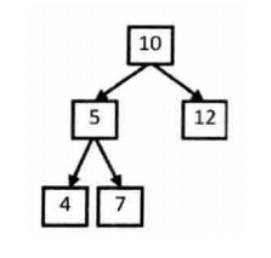本题使用前序遍历的方式访问节点，使用二维向量allpath存储全部路径，使用一维向量path存储当前路径。遍历二叉树的过程：按前序遍历顺序访问每一个节点。访问每个结点时，将结点添加到路径向量path中。如果当前结点是叶子结点，则判断当前路径是否是符合条件的路径，符合条件的路径存入到二维向量allpath；如果当前结点不是叶子结点，则递归当前节点的左右子节点。
那么有一个很重要的问题是：先序遍历便是先左后右。检查完左子树后，会对path就行修改，再去查找右子树，如何将path恢复到之前未进行左子树检查的状态？dfs的过程是先序遍历的过程，所以一旦遍历到叶子结点，便将path最后的节点移除掉，这样在递归一层一层进行的时候将值添加进path,在递归返回的过程中将path最末尾的元素一个一个移除。这样便依靠递归的特性完成了路径的恢复。
/*
struct TreeNode {
int val;
struct TreeNode *left;
struct TreeNode *right;
TreeNode(int x) :
val(x), left(NULL), right(NULL) {
}
};*/
class Solution {
public:
vector<vector<int>> allpath;
vector<int> path;
vector<vector<int> > FindPath(TreeNode* root,int expectNumber) {
if(root) {
dfsfind(root, expectNumber);
}
return allpath;
}
void dfsfind(TreeNode * root,int expectNumber){
path.push_back(root->val);
if(!root->left&&!root->right){//当遍历到叶节点的时候
if(expectNumber-root->val==0){//此条路径满足要求
allpath.push_back(path);
}
}
else {
if (root->left != NULL) {//如果存在左子树　递归左子树
dfsfind(root->left, expectNumber - root->val);
}
if (root->right != NULL) {//存在右子树　递归右子树
dfsfind(root->right, expectNumber - root->val);
}
}
if(!path.empty()){ //删除最后的结点找下一个路径
path.pop_back();
}
}
};

２５．复杂链表的复制
题目描述
思路
如下所示是一个复杂链表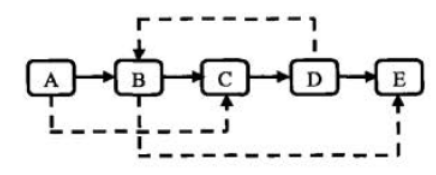第一步：根据原始链表的每个结点N 创建对应的 N’。把 N’链接在N的后面。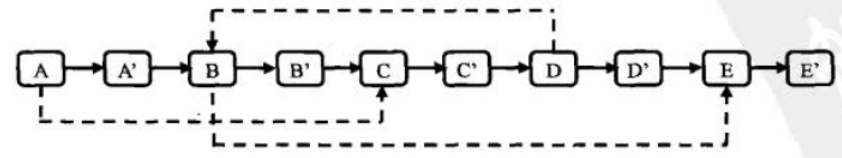第二步：设置复制出来的结点的 sibling。假设原始链表上的 N 的 sibling 指向结点 S，那么其对应复制出来的 N’是 N的 pext 指向的结点，同样 S’也是 S 的 next 指向的结点。设置 sibling 之后的链表下图所示。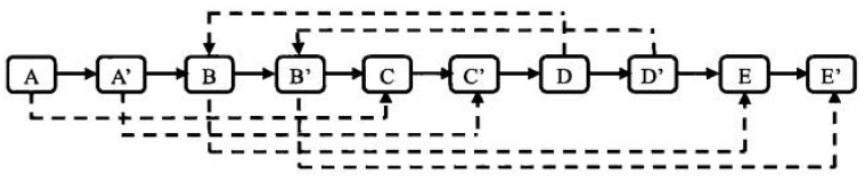第三步：把这个长链表拆分成两个链表。把奇数位置的结点用 next 链接起来就是原始链表，把偶数位置的结点用 next 链接起来就是复制 出来的链表。链表拆分之后的两个链表下图所示。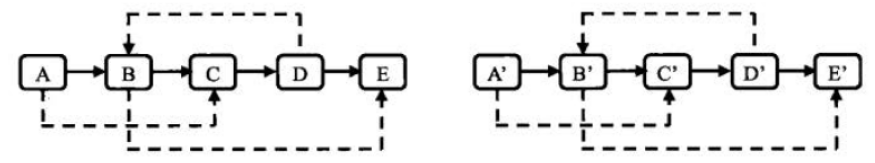/*
struct RandomListNode {
int label;
struct RandomListNode *next, *random;
RandomListNode(int x) :
label(x), next(NULL), random(NULL) {
}
};
*/
class Solution {
public:
{
while(p){ //完成第一步
RandomListNode *tmp=new RandomListNode(p->label);
tmp->next=p->next;
p->next=tmp;
p=tmp->next;
}
while (p){
if(p->random!=NULL) {
p->next->random = p->random->next;//找到random指针赋值
}
p=p->next->next;//遍历
}
while (p){
p->next=c->next;
p=p->next;
if(p==NULL){
c->next=NULL;
}
else {
c->next = p->next;
c = c->next;
}
}
}
};

２６．二叉搜索树与双向链表
题目描述
输入一棵二叉搜索树，将该二叉搜索树转换成一个排序的双向链表。要求不能创建任何新的结点，只能调整树中结点指针的指向。
思路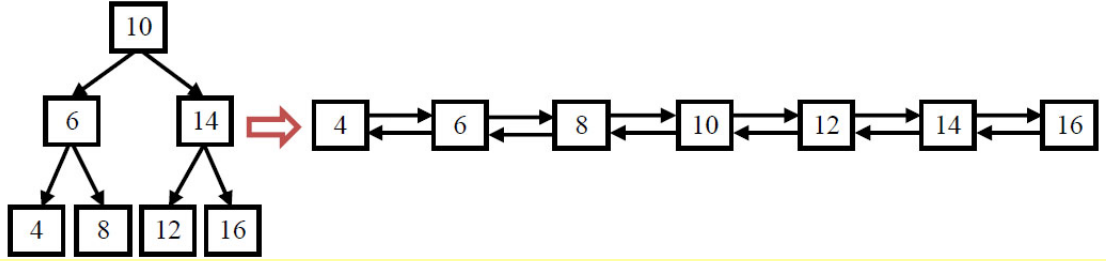/*
struct TreeNode {
int val;
struct TreeNode *left;
struct TreeNode *right;
TreeNode(int x) :
val(x), left(NULL), right(NULL) {
}
};*/
class Solution {
public:
TreeNode* Convert(TreeNode* pRootOfTree)
{
if(pRootOfTree==NULL||(pRootOfTree->left==NULL&&pRootOfTree->right==NULL)){
return pRootOfTree;
}
TreeNode *left=Convert(pRootOfTree->left);
TreeNode *p=left;
//找最大的那个结点
while (p!=NULL&&p->right!=NULL){
p=p->right;
}
//改变链接位置
if(left!=NULL) {
pRootOfTree->left = p;
p->right = pRootOfTree;
}
//右子树
TreeNode *right=Convert(pRootOfTree->right);
if(right!=NULL){
right->left=pRootOfTree;
pRootOfTree->right=right;
}
return left == NULL ? pRootOfTree : left;
}
};

关于递归掌握的还是不是很好。。。我哭了。。。
２７．字符串的排序
题目描述
输入一个字符串,按字典序打印出该字符串中字符的所有排列。例如输入字符串abc,则打印出由字符a,b,c所能排列出来的所有字符串abc,acb,bac,bca,cab和cba。
输入描述
输入一个字符串,长度不超过9(可能有字符重复),字符只包括大小写字母。
思路
解题思路：本题求整个字符串的全排列可以看做两步
1）首先求出所有可能出现在第一位置的字母，即begin与后面所有与它不同的字母进行交换
2）固定第一个字母，求后面字母的全排列，即递归此时begin = begin+1
class Solution {
vector<string> allpath;
public:
vector<string> Permutation(string str) {
if(str.empty()){
return allpath;
}
permutation(str,0);//进行递归
sort(allpath.begin(),allpath.end());//按照字典序输出
return allpath;
}
void permutation(string str,int position){
if(position==str.size()-1){//递归终止条件
allpath.push_back(str);
}
for (int i=position;i<str.size();i++){
if(i!=position&&str[i]==str[position]){//字母相同则不交换
continue;
}
swap(str[i],str[position]);
permutation(str,position+1); //递归操作
swap(str[i],str[position]);
}
}
};

２８．数组中出现次数超过一半的数字
题目描述
数组中有一个数字出现的次数超过数组长度的一半，请找出这个数字。例如输入一个长度为9的数组{1,2,3,2,2,2,5,4,2}。由于数字2在数组中出现了5次，超过数组长度的一半，因此输出2。如果不存在则输出0。
思路
一个数字在数组中出现次数超过了一半，则排序后，位于数组中间的数字一定就是该出现次数超过了长度一半的数字（可以用反证法证明），也即是说，这个数字就是统计学上的中位数。所以就取排序后在数组最中间的数字。如果存在长度超过一半的数字，那么这个数字一定是这个数字。是否存在则需要遍历一遍数组。计算出这个数字在数组中出现的次数。如果大于数组的长度就返回这个数字。否则返回0。
class Solution {
public:
int MoreThanHalfNum_Solution(vector<int> numbers) {
sort(numbers.begin(),numbers.end());
int num=numbers[numbers.size()/2];
int times=0;
for(int i=0;i<numbers.size();i++){
if(numbers[i]==num){
times++;
}
}
if(times>numbers.size()/2){
return num;
}
return 0;
}
};

２９．最小的k个数
题目描述
输入n个整数，找出其中最小的K个数。例如输入4,5,1,6,2,7,3,8这8个数字，则最小的4个数字是1,2,3,4,。
思路
这个题太简单了，就是排序。能stl中的函数，绝对不自己写。。。。当然快排啥的代码还是要熟记于心。
class Solution {
public:
vector<int> GetLeastNumbers_Solution(vector<int> input, int k) {
vector<int> res;
if(k>input.size()){
return res;
}
sort(input.begin(),input.end());
for(int i=0;i<k;i++){
res.push_back(input[i]);
}
return res;
}
};

３０．连续子数组的最大和
题目描述
HZ偶尔会拿些专业问题来忽悠那些非计算机专业的同学。今天测试组开完会后,他又发话了:在古老的一维模式识别中,常常需要计算连续子向量的最大和,当向量全为正数的时候,问题很好解决。但是,如果向量中包含负数,是否应该包含某个负数,并期望旁边的正数会弥补它呢？例如:{6,-3,-2,7,-15,1,2,2},连续子向量的最大和为8(从第0个开始,到第3个为止)。给一个数组，返回它的最大连续子序列的和，你会不会被他忽悠住？(子向量的长度至少是1)
思路
这个题目是一个动态规划的题目，我们可以用dp[i]来表示以i结束的最大子序列和。array为原来的数组。则我们可以知道有以下关系，i表示遍历下标。
if(i==0||dp[i-1]<=0) dp[i]=array[i]
if(dp[i-1]>0) dp[i]=dp[i-1]+array[i]

class Solution {
public:
int FindGreatestSumOfSubArray(vector<int> array) {
if(array.empty()){
return 0;
}
vector<int> dp(array.size());
dp=array;
int max=-999999999;//初始设置一个很小的数
for (int i=1;i<array.size();i++){
if(dp[i-1]<=0){
dp[i]=array[i];

}
else{
dp[i]=dp[i-1]+array[i];
}
if(dp[i]>max){ //在dp中找最大的
max=dp[i];
}
}
return max;
}
};

３１．整数中１出现的次数
题目描述
求出1到13的整数中1出现的次数,并算出100到1300的整数中1出现的次数？为此他特别数了一下1~13中包含1的数字有1、10、11、12、13因此共出现6次,但是对于后面问题他就没辙了。ACMer希望你们帮帮他,并把问题更加普遍化,可以很快的求出任意非负整数区间中1出现的次数（从1 到 n 中1出现的次数）。
思路
最简单的方法，暴力遍历。
class Solution {
public:
int NumberOf1Between1AndN_Solution(int n)
{
int count = 0;
for(int i=0; i<=n; i++){
int temp = i;
//如果temp的任意位为1则count++
while(temp!=0){
if(temp%10 == 1){
count++;
}
temp /= 10;
}
}
return count;
}
};

３２．把数组排成最小的数
题目描述
输入一个正整数数组，把数组里所有数字拼接起来排成一个数，打印能拼接出的所有数字中最小的一个。例如输入数组{3，32，321}，则打印出这三个数字能排成的最小数字为321323。
思路
显而易见，要重新定义两个数的大小，排好序之后按顺序将字符串拼接起来即可。
如何定义两个数的大小，不太容易立刻想到。其实很简单，为了方便，先将所有的数字转换成字符串，想要比较字符串a和字符串b的大小，就是比较拼接之后的字符串ab和字符串ba的大小。如果ab < ba，则a<b。定义好这个规则之后，就可以用c++算法库中的sort 函数进行排序，最终将所有的字符串拼接起来。
注意
定义给sort函数使用的比较函数comp时，需要定义成静态函数或者全局函数，因为sort函数的第三个参数是具有两个形参的函数，而类函数会隐含的传递this指针，其实会多一个参数，导致sort函数运行报错！
如果非要用类函数的话，也不是没有办法。可以使用c++11的bind函数（注意包含头文件 #include <functional>）
写法如下：
bool comp(const string &str1,const string &str2){
string s1 = str1+str2;
string s2 = str2+str1;
return s1 < s2;
}
auto func = std::bind(&comp, this, std::placeholders::1, std::placeholders::2);
sort(nums_str.begin(), nums_str.end(),func);

class Solution {
public:
static bool comp(const string &str1,const string &str2){
string s1=str1+str2;
string s2=str2+str1;
return s1<s2;
}

string PrintMinNumber(vector<int> numbers) {

vector<string> allstr;
string result;
if(numbers.empty()){
return result;
}
for(int i=0;i<numbers.size();i++){
allstr.push_back(to_string(numbers[i]));
}
sort(allstr.begin(),allstr.end(),comp);
for(int i=0;i<allstr.size();i++){
result=result+allstr[i];
}
return result;
}

};

３３．丑数
题目描述
把只包含质因子2、3和5的数称作丑数（Ugly Number）。例如6、8都是丑数，但14不是，因为它包含质因子7。 习惯上我们把1当做是第一个丑数。求按从小到大的顺序的第N个丑数。
思路
最简单的方法就是先通过将一个数不断除以2，3，5来判定该数是不是丑数，而后在从1开始，依次往后判断每个数是不是丑数，并记下丑数的个数，这样当计算的个数为给定值时，便是需要求的第n个丑数，这种方法的时间复杂度为O（k），这里的k为第n个丑数的大小，比如第1500个丑数的大小为859963392，那么就需要判859963392次，时间效率非常低。
直观的优化措施就是看能不能将时间复杂度降低到O（n），即只在丑数上花时间，而不在非丑数上浪费时间。其核心思想是：每一个丑数必然是由之前的某个丑数与2，3或5的乘积得到的，这样下一个丑数就用之前的丑数分别乘以2，3，5，找出这三这种最小的并且大于当前最大丑数的值，即为下一个要求的丑数。
class Solution {
public:
int GetUglyNumber_Solution(int index) {
if(index==0){
return 0;
}
int *uglynumber=new int[index];
//分别表示应该成对应的书的index
int m2=0;
int m3=0;
int m5=0;
int nextindex=1;
uglynumber=1;
while (nextindex<index){
//下一个丑数赋值，明显应该取乘2,乘3,乘5的最小的那个。
uglynumber[nextindex]=min(uglynumber[m2]*2,uglynumber[m3]*3,uglynumber[m5]*5);
while (uglynumber[m2]*2<=uglynumber[nextindex]){
m2++;
}
while (uglynumber[m3]*3<=uglynumber[nextindex]){
m3++;
}
while (uglynumber[m5]*5<=uglynumber[nextindex]){
m5++;
}
nextindex++;
}
return uglynumber[index-1];
}

int min(const int &a,const int &b,const int &c){
int m=(a>b?b:a);
return (c>m?m:c);
}

};

３４．第一个只出现一次的字符
题目描述
在一个字符串(0<=字符串长度<=10000，全部由字母组成)中找到第一个只出现一次的字符,并返回它的位置, 如果没有则返回 -1（需要区分大小写）
思路
对于题目要求，想到的是对于每一个字符，记录它出现的次数，然后再一次遍历整个字符串，第一个出现次数为1的字符的位置即为所求。因此采用hash表的思想。对于一个字符将其ASCII码为数组下标所对应的值+1，即其ASCII码为数组下标对应的数组值为其在字符串中出现的次数。
class Solution {
public:
int FirstNotRepeatingChar(string str) {
const int size=256;
int hashtable[size]={0};
for(int i=0;i<str.size();i++){
hashtable[str[i]]+=1;
}
for(int i=0;i<str.size();i++){
if(hashtable[str[i]]==1){
return i;
}
}
return -1;
}
};

３５．数组中的逆序对
题目描述
在数组中的两个数字，如果前面一个数字大于后面的数字，则这两个数字组成一个逆序对。输入一个数组,求出这个数组中的逆序对的总数P。并将P对1000000007取模的结果输出。 即输出P%1000000007
输入描述
题目保证输入的数组中没有的相同的数字数据范围：
对于%50的数据,size<=10^4
对于%75的数据,size<=10^5
对于%100的数据,size<=2*10^5

思路
直接求解的时间复杂度太高，所以还是用归并排序的思想
首先拆分，然后合并，以数组｛7, 5, 6, 4｝为例来分析统计逆序对的过程。
（１）拆分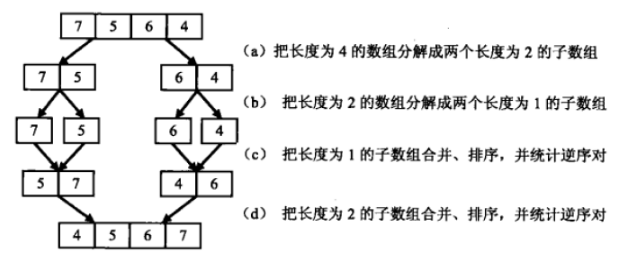（２）归并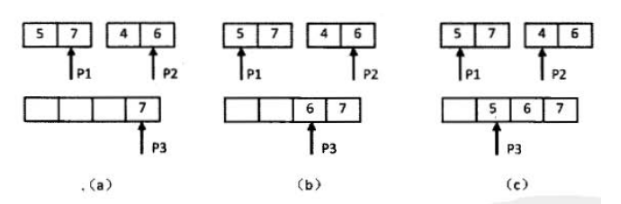（a）P1指向的数字大于P2指向的数字，表明数组中存在逆序对．P2 指向的数字是第二个子数组的第二个数字， 因此第二个子数组中有两个数字比7 小． 把逆序对数目加2，并把7 复制到辅助数组，向前移动P1和P3.
（b） P1指向的数字小子P2 指向的数字，没有逆序对．把P2 指向的数字复制到辅助数组，并向前移动P2 和P3 .
（c） P1指向的数字大于P2 指向的数字，因此存在逆序对． 由于P2 指向的数字是第二个子数组的第一个数字，子数组中只有一个数字比5 小． 把逆序对数目加1 ，并把5复制到辅助数组，向前移动P1和P3 .
（d）复制第二个子数组最后剩余的4 到辅助数组中
所以最后的返回的应该是left的逆序对+right的逆序对+归并的逆序对。
class Solution {
public:
int InversePairs(vector<int> data)
{
int length = data.size();
if(length <= 0)
return 0;
//定义辅助数组
vector<int> copy;
for(int i=0;i<length;i++)
{
copy.push_back(data[i]);
}
int count = InversePairsCore(data,copy,0,length-1);

return count;
}
int InversePairsCore(vector<int> &data,vector<int> &copy,int start,int end)
{
//结束递归条件,只剩一个元素
if(start == end)
{
copy[start] = data[start];
return 0;
}
int length = (end-start)/2;
int left = InversePairsCore(copy,data,start,start+length)%1000000007;
int right = InversePairsCore(copy,data,start+length+1,end)%1000000007;

//i初始化为前半段最后一个数字下标
int i = start+length;
//j吃书画问后半段最后一个数字下标
int j = end;
int indexCopy = end;
int count = 0;
while(i>=start && j>=start+length+1)
{
if(data[i]>data[j])
{
copy[indexCopy--] = data[i--];
count+=j-start-length;//右侧一共有j-start-length个元素，都小于data[i]
if(count >= 1000000007)
{
count %= 1000000007;
}
}
else
{
copy[indexCopy--] = data[j--];//右侧元素大不存在逆序对
}
}
for(;i>=start;i--)
{
copy[indexCopy--] = data[i];
}
for(;j>=start+length+1;j--)
{
copy[indexCopy--] = data[j];
}

return (left+right+count)%1000000007;
}
};

依然递归。。。。什么时候能写好递归。。。。。
３６．两个链表的第一个公共结点
题目描述
输入两个链表，找出它们的第一个公共结点。
思路
遍历链表求得两个链表各自的长度；因为两个链表的最后部分是重叠的，所以先让长的链表走几步，相同后同时向前遍历。过程中节点相同的时候，即为第一个公共节点。时间复杂度O(m+n)
/*
struct ListNode {
int val;
struct ListNode *next;
ListNode(int x) :
val(x), next(NULL) {
}
};*/
class Solution {
public:
return NULL;
}
int L1=0,L2=0;
while (p1){
p1=p1->next;
L1++;
}
while (p2){
p2=p2->next;
L2++;
}
int k=L2-L1;
if(k>=0){//说明L2这个更长
}
else{
k=-k;
}
while (p3){
if(k>0){
p3=p3->next;
k--;
}
else if(p3==p4){
return p3;
}
else{
p3=p3->next;
p4=p4->next;
}
}
return NULL;
}
};

３７．数字在排序数组中出现的次数
题目描述
统计一个数字在排序数组中出现的次数。
思路
本题因为数组是有序的，因此采用二分查找的方式找到k，要知道k出现次数，先找到第一个k出现的位置，再找到最后一个k出现的位置，最后k出现次数为last-first+1
class Solution {
public:
int GetNumberOfK(vector<int> data ,int k) {
int length=data.size();
if(length==0){
return 0;
}
int first=0,last=0;
int start=0,end=length-1,mid=0;
//找左边的
while(true){
if(start>end){
return 0;
}
mid=(start+end)/2;
if(data[mid]==k){
if(mid==0||data[mid-1]!=k){//前一个不为k或者这一个为0说明是第一个k
first=mid;
break;
}
else{
end=mid-1;
}
}
else if(data[mid]>k){
end=mid-1;
}
else{
start=mid+1;
}
}
//找右边的
start=0,end=length-1,mid=0;
while(true){
if(start>end){
return 0;
}
mid=(start+end)/2;
if(data[mid]==k){
if(mid==length-1||data[mid+1]!=k){//为最后一个或者后一个不为k
last=mid;
break;
}
else{
start=mid+1;
}
}
else if(data[mid]>k){
end=mid-1;
}
else{
start=mid+1;
}
}
return last-first+1;
}
};

３８．二叉树的深度
题目描述
输入一棵二叉树，求该树的深度。从根结点到叶结点依次经过的结点（含根、叶结点）形成树的一条路径，最长路径的长度为树的深度。
思路
1、只有一个根结点，树的深度为1
2、求左子树的深度
3、求右子树的深度
4、树的深度为两个子树中大的那个
/*
struct TreeNode {
int val;
struct TreeNode *left;
struct TreeNode *right;
TreeNode(int x) :
val(x), left(NULL), right(NULL) {
}
};*/
class Solution {
public:
int TreeDepth(TreeNode* pRoot)
{
if(pRoot==NULL){
return 0;
}
int left=1+TreeDepth(pRoot->left);
int right=1+TreeDepth(pRoot->right);
return  left>right?left:right;
}
};

３９．平衡二叉树
题目描述
输入一棵二叉树，判断该二叉树是否是平衡二叉树。
思路
平衡二叉树，对于每个根节点左右子树高度差小于等于1，所以就是求左右子树的深度，然后再依次判断是否相差１以内。
class Solution {
public:
int TreeDepth(TreeNode* pRoot)
{
if(pRoot==NULL){
return 0;
}
int left=1+TreeDepth(pRoot->left);
int right=1+TreeDepth(pRoot->right);
return  left>right?left:right;
}
bool IsBalanced_Solution(TreeNode* pRoot){
if(pRoot==NULL){
return true;
}
int left=TreeDepth(pRoot->left);
int right=TreeDepth(pRoot->right);
int gap=abs(left-right);
if(gap<=1){
return true;
}
else return false;
return IsBalanced_Solution(pRoot->left)&&IsBalanced_Solution(pRoot->right);
}
};

４０．数组中只出现一次的数字
题目描述
一个整型数组里除了两个数字之外，其他的数字都出现了两次。请写程序找出这两个只出现一次的数字。
思路
这个题目可以用异或的思路来做
首先先看看异或的基础知识
异或是一种基于二进制的位运算，用符号XOR或者 ^ 表示，其运算法则是对运算符两侧数的每一个二进制位，同值取0，异值取1。它与布尔运算的区别在于，当运算符两侧均为1时，布尔运算的结果为1，异或运算的结果为0。
异或的性质：

1、交换律：a^b = b^a；

2、结合律：(a^b)^c = a^(b^c)；

3、对于任意的a：a^a=0，a^0=a，a^(-1)=~a。

对于任意的a，有a^b^c^d^a^k = b^c^d^k^(a^a) = b^c^d^k^0 = b^c^d^k，也就是说，如果有多个数异或，其中有重复的数，则无论这些重复的数是否相邻，都可以根据异或的性质将其这些重复的数消去，具体来说，如果重复出现了偶数次，则异或后会全部消去，如果重复出现了奇数次，则异或后会保留一个。
（１）有一个数组中只有一个数字出现了一次，其他的全部出现了两次，求出这个数字。
这个很容易了，将数组中所有的元素全部异或，最后出现两次的元素会全部被消去，而最后会得到该只出现一次的数字。
（２）本题目中出现一次的数字有两个，那这道题目应该怎么做呢？
两个数（我们假设是AB）出现一次的数组。我们首先还是先异或，剩下的数字肯定是A、B异或的结果，这个结果的二进制中的1，表现的是A和B的不同的位。我们就取第一个1所在的位数，假设是第3位，接着把原数组分成两组，分组标准是第3位是否为1。如此，相同的数肯定在一个组，因为相同数字所有位都相同，而不同的数，肯定不在一组。然后把这两个组按照最开始的思路，依次异或，剩余的两个结果就是这两个只出现一次的数字。
在这里补充一点非常基础的知识。因为我写代码的时候就忘记了
a>>1 //代表a的最后一位去掉，如当a=５时，二进制为101，进行这个操作之后，二进制为10,十进制为２
a<<1 //代表a最后一位加０，如当a＝５时，二进制为101，进行这个操作后，二进制为1010，十进制为10
8bit=1byte
求int类型的bit为8*sizeof(int)

class Solution {
public:
void FindNumsAppearOnce(vector<int> data,int* num1,int *num2) {
if(data.empty())
return;
int rel=0;//不同的两个数的异或结果
for(int i=0;i<data.size();i++){
rel^=data[i];
}
int index=findindex(rel);
*num1 = *num2 = 0;
for(int i=0;i<data.size();i++){
if(((data[i]>>index))&1==1){
*num1^=data[i];
}
else {
*num2^=data[i];
}
}

}
int findindex(int n){//求从右边数起第一位是1的数
int index=0;
while(((n&1)==0)&&index<=8* sizeof(int)){
n=n>>1;
index++;
}
return index;
}
};

４１．和为S的连续整数序列
题目描述
小明很喜欢数学,有一天他在做数学作业时,要求计算出9~16的和,他马上就写出了正确答案是100。但是他并不满足于此,他在想究竟有多少种连续的正数序列的和为100(至少包括两个数)。没多久,他就得到另一组连续正数和为100的序列:18,19,20,21,22。现在把问题交给你,你能不能也很快的找出所有和为S的连续正数序列? Good Luck!
输出描述
输出所有和为S的连续正数序列。序列内按照从小至大的顺序，序列间按照开始数字从小到大的顺序

思路
1、定义start = 1，end =2 ，然后和res = start+end；如果res == sum，则表示找到一个序列，把start到end的值存入vector
2、如果大于的话，依次从小到大删除start，res减少，直到相等或者结束
3、如果小于的话，增大end，再次执行循环
循环结束条件start == (1+sum)/2
class Solution {
public:
vector<vector<int> > FindContinuousSequence(int sum) {
vector<vector<int>> allpath;
vector<int> path;
if(sum<3){
return allpath;
}
int start=1,end=2;
int n=0;
while (start<(1+sum)/2){
n=(start+end)*(end-start+1)/2;//当前序列和　等差数列和=(首项＋末项)*个数/2
if(n==sum){　//等于sum说明当前序列满足条件
for(int i=start;i<=end;i++){
path.push_back(i);
}
allpath.push_back(path);
path.clear();
end++;
start++;
}
else if(n>sum){//增大start 和变小
start=start+1;
}
else{
end=end+1;//增大end 和变大
}
}
return allpath;
}
};

４２．和为S的两个数字
题目描述
输入一个递增排序的数组和一个数字S，在数组中查找两个数，使得他们的和正好是S，如果有多对数字的和等于S，输出两个数的乘积最小的。
思路
本题主要是如何保证找到的两个数的乘积是最小的。通过证明，定义start、end一个指向数组头，一个指向数组尾。如果相等，则为找到，大于sum，则end–，小于sum则start++，这样找到的第一组即为乘积最小的一组。
class Solution {
public:
vector<int> FindNumbersWithSum(vector<int> array,int sum) {
vector<int> result;
if(array.empty())
return result;
int start=0,end=array.size()-1;
while (end>start){
int n=array[start]+array[end];
if(n==sum){
result.push_back(array[start]);
result.push_back(array[end]);
break;
}
else if(n>sum){
end--;
}
else{
start++;
}
}
return result;
}
};

４３．左旋转字符串
题目描述
汇编语言中有一种移位指令叫做循环左移（ROL），现在有个简单的任务，就是用字符串模拟这个指令的运算结果。对于一个给定的字符序列S，请你把其循环左移K位后的序列输出。例如，字符序列S=”abcXYZdef”,要求输出循环左移3位后的结果，即“XYZdefabc”。是不是很简单？OK，搞定它！
思路
这个很简单了，利用stl中的string的一些api就可以很容易的实现
class Solution {
public:
string LeftRotateString(string str, int n) {
if (str.empty()){
return str;
}
for (int i=0;i<n;i++){
char tmp=str;
str.erase(str.begin());
str.push_back(tmp);
}
return str;
}
};

４４．翻转单词顺序列
题目描述
牛客最近来了一个新员工Fish，每天早晨总是会拿着一本英文杂志，写些句子在本子上。同事Cat对Fish写的内容颇感兴趣，有一天他向Fish借来翻看，但却读不懂它的意思。例如，“student. a am I”。后来才意识到，这家伙原来把句子单词的顺序翻转了，正确的句子应该是“I am a student.”。Cat对一一的翻转这些单词顺序可不在行，你能帮助他么？
思路
可以用一个stack存放每个单词，然后再将单词组成句子,但是这样子就会多用一个栈的空间。所以不再另外开辟空间的做法是先翻转整个句子。然后再翻转单词。
class Solution {
public:
string ReverseSentence(string str) {
if(str.empty()){
return str;
}
int s=0,e=str.size()-1;
while (s<e){//反转整个句子
swap(str[s],str[e]);
s++;
e--;
}
int start=0,end=0;
for(int i=0;i<str.size();i++){
if(i==0||str[i-1]==' '){//找单词的开始
start=i;
}
if(i+1==str.size()||str[i+1]==' '){//找单词的结束
end=i;
}
if(start<=end){//反转单词
while (start<end){
swap(str[start],str[end]);
start++;
end--;
}
}
}
return str;
}
};

４５．扑克牌顺子
题目描述
LL今天心情特别好,因为他去买了一副扑克牌,发现里面居然有2个大王,2个小王(一副牌原本是54张_)…他随机从中抽出了5张牌,想测测自己的手气,看看能不能抽到顺子,如果抽到的话,他决定去买体育彩票,嘿嘿！！“红心A,黑桃3,小王,大王,方片5”,“Oh My God!”不是顺子…LL不高兴了,他想了想,决定大\小 王可以看成任何数字,并且A看作1,J为11,Q为12,K为13。上面的5张牌就可以变成“1,2,3,4,5”(大小王分别看作2和4),“So Lucky!”。LL决定去买体育彩票啦。 现在,要求你使用这幅牌模拟上面的过程,然后告诉我们LL的运气如何， 如果牌能组成顺子就输出true，否则就输出false。为了方便起见,你可以认为大小王是0。
思路
本题采用排序处理，然后记录数组中0的个数，以及牌间隔的差值和，如果0的个数大于等于差值则，可以构成顺子，否则不可以。其中如果在遍历中发现有相等的，则退出，返回false
class Solution {
public:
bool IsContinuous( vector<int> numbers ) {
if (numbers.empty()){
return false;
}
sort(numbers.begin(),numbers.end());
int king=0;
for(int i=0;i<numbers.size();i++){
if(numbers[i]==0){
king++;
continue;
}
if(i>0&&numbers[i]==numbers[i-1]){
return false;
}
if(numbers[i-1]!=0&&numbers[i]-numbers[i-1]>king+1){
return false;
}
if(numbers[i-1]!=0&&numbers[i]-numbers[i-1]<=king+1){
king=king+1-(numbers[i]-numbers[i-1]);
}
}
return true;
}
};

４６．孩子们的游戏
题目描述
每年六一儿童节,牛客都会准备一些小礼物去看望孤儿院的小朋友,今年亦是如此。HF作为牛客的资深元老,自然也准备了一些小游戏。其中,有个游戏是这样的:首先,让小朋友们围成一个大圈。然后,他随机指定一个数m,让编号为0的小朋友开始报数。每次喊到m-1的那个小朋友要出列唱首歌,然后可以在礼品箱中任意的挑选礼物,并且不再回到圈中,从他的下一个小朋友开始,继续0…m-1报数…这样下去…直到剩下最后一个小朋友,可以不用表演,并且拿到牛客名贵的“名侦探柯南”典藏版(名额有限哦!!_)。请你试着想下,哪个小朋友会得到这份礼品呢？(注：小朋友的编号是从0到n-1)
如果没有小朋友，请返回-1
思路
很简单，就按照题意模拟这个过程,用i来表示对小朋友的遍历，用j来计数是否应该删除小朋友了。用一个vector来存放小朋友，用编号表示小朋友，然后当j到m-1时，删除相应的小朋友。
class Solution {
public:
int LastRemaining_Solution(int n, int m)
{
if(n<=0||m<=0){
return -1;
}
vector<int> flag;//编号表示小朋友
for(int i=0;i<n;i++){
flag.push_back(i);
}
int i=0;
int j=0;
while (flag.size()>1){//当只有一个小朋友时，返回这个小朋友
if(i==flag.size()){//遍历到最后一个小朋友后，再从第一个小朋友开始
i=0;
}
if(j==m-1){//到m-1删除小朋友
flag.erase(flag.begin()+i);
j=0;
} else{　//遍历小朋友
i++;
j++;
}

}
return flag;
}
};

４７．求1+2+3…n
题目描述
求1+2+3+…+n，要求不能使用乘除法、for、while、if、else、switch、case等关键字及条件判断语句（A?B:C）
思路
本题采用递归的方式实现，见代码吧。主要是将终止条件用了result&&(result=n+Sum_Solution(n-1));来代替if判断
class Solution {
public:
int Sum_Solution(int n) {

int result=n;
result&&(result=n+Sum_Solution(n-1));
return result;

}
};

４８．不用加减乘除做加法
题目描述
写一个函数，求两个整数之和，要求在函数体内不得使用+、-、*、/四则运算符号。
思路
其实一个加法可以分解为两个部分，一个是有进位的加法，一个是没有进位的加法。
例如1100+1001
没有进位的加法实际上是做异或。1100^1001=0101
有进位的实际上是做与操作以后再左移一位。1100&1001=1000<<1=10000
然后再将这两部分加起来，其实就相当于是做按个过程的循环，直到没存在有进位的情况。
class Solution {
public:
{
while (num2!=0){
num2=num1&num2;
num2=num2<<1;
}
return num1;
}
};

４９．把字符串转换成整数
题目描述
将一个字符串转换成一个整数，要求不能使用字符串转换整数的库函数。 数值为0或者字符串不是一个合法的数值则返回0
如果是合法的数值表达则返回该数字，否则返回0
输入：+2147483647
​            1a33
输出：2147483647
0
思路
很简单　主要是利用ascii
int StrToInt(string str) {
long long  num=0;
long long idx=1;
if(str>'0'&&str<'9'||str=='-'||str=='+'){
for (int i=str.size()-1;i>=0;i--) {
if(i==0&&str=='-'){
num=num*-1;
if(num< (signed int)0x80000000){
num=0;
break;
}
}
else if(i==0&&str=='+'){
continue;
}
else if(str[i]>'0'&&str[i]<'9'){
num=num+(str[i]-'0')*idx;
idx=idx*10;
}
else {
num=0;
break;
}

}
}
else{
return 0;
}
if(num > 0x7FFFFFFF){
return 0;
}
return num;
}

５０．数组中重复的数字
题目描述
在一个长度为n的数组里的所有数字都在0到n-1的范围内。 数组中某些数字是重复的，但不知道有几个数字是重复的。也不知道每个数字重复几次。请找出数组中任意一个重复的数字。 例如，如果输入长度为7的数组{2,3,1,0,2,5,3}，那么对应的输出是第一个重复的数字2。
思路
一、一个简单的思路是先将数组排序，然后从头开始寻找重复数字。排序的时间复杂度为O(nlogn)；
二、利用hash表存储元素，若表中存在元素则找到重复数字。Hash查询时间仅用O(1)，算法时间复杂度为O(n)，但是需要一个哈希表，空间复杂度为O(n)；
三、利用ｎ和ｎ-1这个关键点
数组中的数字都在0到n-1的数字范围内。如果数组中没有重复出现的数字，那么当数组排序后数字i就出现在数组中下标为i的元素处。那么数组中如果存在重复数字的话，有些位置的对应的数字就没有出现，而有些位置可能存在多个数字。数组用numbers表示
那么我们重排这个数组。从第0个元素开始。

比较numbers[i]和i的值，如果i与numbers[i]相等，也就是对数组排序后，numbers[i]就应该在对应的数组的第i个位置处，那么继续判断下一个位置。
如果i和numbers[i]的值不相等，那么判断以numbers[i]为下标的数组元素是什么。

如果numbers[numbers[i]]等于numbers[i]的话，那么就是说有两个相同的值了，重复了。找到了重复的数字
如果numbers[numbers[i]]不等于numbers[i]的话，那么就将numbers[numbers[i]]和numbers[i]互换。继续进行1的判断。

循环退出的条件是直至数组最后一个元素，仍没有找到重复的数字，数组中不存在重复的数字。

class Solution {
public:
// Parameters:
//        numbers:     an array of integers
//        length:      the length of array numbers
//        duplication: (Output) the duplicated number in the array number
// Return value:       true if the input is valid, and there are some duplications in the array number
//                     otherwise false
bool duplicate(int numbers[], int length, int* duplication) {
bool flag= false;
int i=0;
while (!flag&& i<length){
if(i!=numbers[i]){
if(numbers[numbers[i]]==numbers[i]){
flag= true;
* duplication=numbers[i];
} else {
int tmp = numbers[numbers[i]];
numbers[numbers[i]] = numbers[i];
numbers[i] = tmp;
}
}
else if(i==numbers[i]){
i=i+1;
}
}
return flag;
}
};

５１．构建乘积数组
题目描述
给定一个数组A[0,1,…,n-1],请构建一个数组B[0,1,…,n-1],其中B中的元素B[i]=AA…*A[i-1]A[i+1]…*A[n-1]。不能使用除法。
解题思路
解题思路：B[i] = (A*A[i]*...*A[i-1]) * (A[i+1]*A[i+2]*...*A[n-1])

C[i] = A*A*...*A[i-1] = C[i-1]*A[i-1];

D[i] = A[i+1]*A[i+2]*...*A[n-1] = A[i+1]*D[i+2];

B[i] = C[i]*D[i];

代码如下
class Solution {
public:
vector<int> multiply(const vector<int>& A) {
vector<int> D(A.size());
D[A.size()-1]=1;
D[A.size()-2]=A[A.size()-1];
for (int i=A.size()-3;i>=0;i--){
D[i]=A[i+1]*D[i+1];
}

vector<int> C(A.size());
C=1;
vector<int> B(A.size());
for (int i=0;i<A.size();i++){
if(i==0){
C[i]=1;
}
else{
C[i]=C[i-1]*A[i-1];
}
B[i]=C[i]*D[i];
}
return B;
}
};

５２．正则表达式匹配
题目描述
请实现一个函数用来匹配包括’.‘和’*‘的正则表达式。模式中的字符’.‘表示任意一个字符，而’*'表示它前面的字符可以出现任意次（包含0次）。 在本题中，匹配是指字符串的所有字符匹配整个模式。例如，字符串"aaa"与模式"a.a"和"ab*ac*a"匹配，但是与"aa.a"和"ab*a"均不匹配
思路
递归的思想。
代码
class Solution {
public:
bool match(char* str, char* pattern)
{
if (*str=='\0'&&*pattern=='\0'){
return true;
}
else if(*str!='\0'&&*pattern=='\0'){
return  false;
}
else if(*str=='\0'&&*pattern!='\0'){
if(*(pattern+1)=='*'){
return match(str,pattern+2);
}
else return false;
}
else{
if(*str==*pattern&&*(pattern+1)!='*'){
return match(str+1,pattern+1);
}
else if(*str==*pattern&&*(pattern+1)=='*'||*pattern=='.'&&*(pattern+1)=='*'){
return (match(str+1,pattern)||match(str+1,pattern+2)||match(str,pattern+2));
}
else if(*str!=*pattern&&*(pattern+1)=='*'){
return match(str,pattern+2);
}
else if(*pattern=='.') {
return match(str + 1, pattern + 1);
}
else return false;
}
}
};

５３．表示数值的字符串
题目描述
请实现一个函数用来判断字符串是否表示数值（包括整数和小数）。例如，字符串"+100",“5e2”,"-123",“3.1416"和”-1E-16"都表示数值。 但是"12e",“1a3.14”,“1.2.3”,"±5"和"12e+4.3"都不是。
思路
很简单，其实就是分情况讨论
代码
class Solution {
public:
//判断是否是无符号的整数
bool isint(char *string){
if(*string=='\0'){
return false;
}
int i=0;
while (string[i]!='\0'){
if(string[i]>'9'||string[i]<'0'){
return false;
}
else{
i++;
}
}
return true;
}
bool isNumeric(char* string)
{
if (*string=='\0'){
return false;
}
if(string=='+'||string=='-'){
string++;
}
//是数字就继续遍历
while(*string!='\0'){
if(*string<='9'&&*string>='0'){
string++;
}
//这是为一个小数的情况
else if(*string=='.'){
if(isint(string+1)){
return true;
}
string=string+1;
//这是小数后边为e整数
while (*string!='e'&&*string!='E'){
if(*string<='9'&&*string>='0'){
string++;
}
else{
return false;
}
}
//找到e的位置
if(*(string+1)=='-'||*(string+1)=='+'){
return isint(string+2);
}
else{
return isint(string+1);
}
}
else if(*string=='e'||*string=='E'){
if(*(string+1)=='-'||*(string+1)=='+'){
return isint(string+2);
}
else{
return isint(string+1);
}
}
else{
return false;
}
}
return true;
}
};

５４．字符流中第一个不重复的字符
题目描述
思路
采用哈希表来实现。用字符的ASCII嘛作为哈希表的键值，而把字符对应的位置作为哈希表的值。遍历所有字母，如果该字母出现1次，且该字符对应的位置<minIndex,更新minIndex。其中-1表示未出现过，-2表示多次出现，0表示只出现一次
代码
class Solution
{
public:
Solution():index(0)
{
for(int i=0;i<256;i++)
occurence[i] = -1;
}
//Insert one char from stringstream
void Insert(char ch)
{
if(occurence[ch] == -1)
occurence[ch] = index;
else if(occurence[ch] >= 0)
occurence[ch] = -2;
index++;
}
//return the first appearence once char in current stringstream
char FirstAppearingOnce()
{
char ch = '\0';
int minIndex = 99999;
for(int i=0;i<256;i++)
{
if(occurence[i] >=0 && occurence[i] <minIndex)//minIndex表示在字符串中出现的位置
{
ch = (char)i;
minIndex = occurence[i];//i存储的是字符串的acii码，数组occ[i]存储的是位置，多次就是-2，没出现就是-1
}
}
if(ch == '\0')//如果当前字符流没有存在出现一次的字符，返回#字符。
return '#';
return ch;
}
private:
int occurence;//其中数组下标i对应与ASCII码
int index;//存储第一个只出现一次的字符的索引

};

５５．链表中环的入口结点
题目描述
给一个链表，若其中包含环，请找出该链表的环的入口结点，否则，输出null。
思路
受到第15题的启发剑指Offer–015-链表中倒数第k个结点, 我们考虑这样一个事实
假设链表长度为N, 那么第N链接到了第k个节点形成了环，即我们需要查找到倒数第N-K+1个节点, 那么环中就有N-K+1个节点，这时候我们定义两个指针P1P1和P2P2指向链表的头部, 指针P1P1先在链表中向前移动n-k+1步,到达第n-k+2个节点, 然后两个指针同步向前移动, 当P2P2走了K-1步到达环的入口的时候, 指针P1P1正好走了N+1步, 到达了环的入口, 即两个指针会相遇在环的入口处
那么我们剩下的问题就是如何得到环中节点的数目?
我们可以使用一快一慢两个指针（比如慢指针一次走一步，　慢指针一次走两步），如果走的过程中发现快指针追上了慢指针, 说明遇见了环，而且相遇的位置一定在环内, 考虑一下环内, 从任何一个节点出现再回到这个节点的距离就是环的长度, 于是我们可以进一步移动慢指针，快指针原地不动, 当慢指针再次回到相遇位置时, 正好在环内走了一圈, 从而我们通过计数就可以获取到环的长度
第一步，找环中相汇点。分别用p1，p2指向链表头部，p1每次走一步，p2每次走二步，直到p1==p2找到在环中的相汇点。
第二步，找环的长度。从环中的相汇点开始, p2不动, p1前移，　当再次相遇时，p1刚好绕环一周, 其移动即为环的长度K
第三步, 求换的起点, 转换为求环的倒数第N-K个节点，则两指针left和right均指向起始, right先走K步, 然后两个指
代码
/*
struct ListNode {
int val;
struct ListNode *next;
ListNode(int x) :
val(x), next(NULL) {
}
};
*/
class Solution {
public:
//通过快慢指针找到在环中的相遇点
{
//找到在环中的结点--快慢指针
return NULL;
while(fast != NULL && fast->next != NULL)
{
slow = slow->next;
fast = fast->next->next;
if(fast == slow)//注意此处先移动，再判断
return fast;
}
return NULL;
}
{
//找到相遇的结点
if(meetNode == NULL)
return NULL;
//找环的结点个数
int nodesInLoop = 1;
ListNode *pNode1 = meetNode;
while(pNode1->next != meetNode)
{
pNode1 = pNode1->next;
nodesInLoop++;
}
//找到之后和倒数k个结点的写法类似
for(int i=0;i<nodesInLoop;i++)
{
pNode1 = pNode1->next;
}
while(pNode1 != pNode2)
{
pNode1 = pNode1->next;
pNode2 = pNode2->next;
}
return pNode1;
}
};

５６．删除链表中重复的结点
题目描述
在一个排序的链表中，存在重复的结点，请删除该链表中重复的结点，重复的结点不保留，返回链表头指针。 例如，链表1->2->3->3->4->4->5 处理后为 1->2->5
思路
解题思路：从头遍历整个链表，如果当前结点和下一结点值相同，则应当删除。为了保证结点不断，需要保存pre结点，然后找到不相等的next，pre->next = next;注意删除的是头结点的情况，单独处理。
代码
/*
struct ListNode {
int val;
struct ListNode *next;
ListNode(int x) :
val(x), next(NULL) {
}
};
*/
class Solution {
public:
{
return NULL;
ListNode *pPreNode = NULL;
while(pNode != NULL)
{
ListNode *pNext = pNode->next;
bool needDelete = false;
if(pNext != NULL && pNext->val == pNode->val)
needDelete = true;
if(!needDelete)//不等
{
pPreNode = pNode;
pNode = pNode->next;
}
else//相等该删除
{
//保存value是为了确保所有值相等的结点都被删除掉了
int value = pNode->val;
ListNode *pToBeDel = pNode;
while(pToBeDel != NULL && pToBeDel->val == value)
{
pNext = pToBeDel->next;
delete pToBeDel;
pToBeDel = NULL;
//下一个结点为将要删除的结点，如果这个结点的值不等于保存的value就会跳出循环
pToBeDel = pNext;
}
if(pPreNode == NULL)//头结点被删除了
else
pPreNode->next = pNext;
pNode = pNext;

}
}
}
};

５７．二叉树的下一个结点
题目描述
给定一个二叉树和其中的一个结点，请找出中序遍历顺序的下一个结点并且返回。注意，树中的结点不仅包含左右子结点，同时包含指向父结点的指针。


展开全文• 文章目录剑指 Offer 06. 从尾到头打印链表利用栈逆向输入数组剑指 Offer 18. 删除链表的节点解法剑指 Offer 22. 链表中倒数第k个节点快慢指针剑指 Offer 24. 反转链表解法剑指 Offer 35. 复杂链表的复制解法剑指 ...
文章目录剑指 Offer 06. 从尾到头打印链表利用栈逆向输入数组剑指 Offer 18. 删除链表的节点解法剑指 Offer 22. 链表中倒数第k个节点快慢指针剑指 Offer 24. 反转链表解法剑指 Offer 35. 复杂链表的复制解法剑指 Offer 52. 两个链表的第一个公共节点解法推荐阅读
剑指 Offer 06. 从尾到头打印链表
剑指 Offer 06. 从尾到头打印链表
输入一个链表的头节点，从尾到头反过来返回每个节点的值（用数组返回）。

示例 1：
输出：[2,3,1]
限制：
0 <= 链表长度 <= 10000
利用栈
/**
* public class ListNode {
*     int val;
*     ListNode next;
*     ListNode(int x) { val = x; }
* }
*/
class Solution {
}
int size = stack.size();
int[] res = new int[size];
int index = 0;
while (!stack.isEmpty()) {
res[index++] = stack.pollFirst();
}
return res;
}
}

逆向输入数组
/**
* public class ListNode {
*     int val;
*     ListNode next;
*     ListNode(int x) { val = x; }
* }
*/
class Solution {
int size = 0;
size++;
}
int[] res = new int[size];
for (int i = size - 1; i >=0; i--) {
}
return res;
}
}

剑指 Offer 18. 删除链表的节点
剑指 Offer 18. 删除链表的节点
给定单向链表的头指针和一个要删除的节点的值，定义一个函数删除该节点。
返回删除后的链表的头节点。
注意：此题对比原题有改动
示例 1:

输入: head = [4,5,1,9], val = 5
输出: [4,1,9]
解释: 给定你链表中值为 5 的第二个节点，那么在调用了你的函数之后，该链表应变为 4 -> 1 -> 9.
示例 2:

输入: head = [4,5,1,9], val = 1
输出: [4,5,9]
解释: 给定你链表中值为 1 的第三个节点，那么在调用了你的函数之后，该链表应变为 4 -> 5 -> 9.

说明：

题目保证链表中节点的值互不相同
若使用 C 或 C++ 语言，你不需要 free 或 delete 被删除的节点

解法
用前一个节点进行比较，可以直接删除节点。还可以用一个哑巴节点做头结点，这样就不用单独判断第一个节点了。
/**
* public class ListNode {
*     int val;
*     ListNode next;
*     ListNode(int x) { val = x; }
* }
*/
class Solution {
public ListNode deleteNode(ListNode head, int val) {
return pre;
}
while (pre.next != null) {
if (pre.next.val == val) {
pre.next = pre.next.next;
}
pre = pre.next;
}
return null;
}
}

剑指 Offer 22. 链表中倒数第k个节点
剑指 Offer 22. 链表中倒数第k个节点
输入一个链表，输出该链表中倒数第k个节点。为了符合大多数人的习惯，本题从1开始计数，即链表的尾节点是倒数第1个节点。例如，一个链表有6个节点，从头节点开始，它们的值依次是1、2、3、4、5、6。这个链表的倒数第3个节点是值为4的节点。

示例：
给定一个链表: 1->2->3->4->5, 和 k = 2.
返回链表 4->5.
快慢指针
/**
* public class ListNode {
*     int val;
*     ListNode next;
*     ListNode(int x) { val = x; }
* }
*/
class Solution {
public ListNode getKthFromEnd(ListNode head, int k) {
while (k-- > 0) {
fast = fast.next;
}
while (fast != null) {
fast = fast.next;
slow = slow.next;
}
return slow;
}
}

剑指 Offer 24. 反转链表
剑指 Offer 24. 反转链表
定义一个函数，输入一个链表的头节点，反转该链表并输出反转后链表的头节点。

示例:

输入: 1->2->3->4->5->NULL
输出: 5->4->3->2->1->NULL

限制：

0 <= 节点个数 <= 5000

解法
/**
* public class ListNode {
*     int val;
*     ListNode next;
*     ListNode(int x) { val = x; }
* }
*/
class Solution {
}
}
}
}

剑指 Offer 35. 复杂链表的复制
剑指 Offer 35. 复杂链表的复制
请实现 copyRandomList 函数，复制一个复杂链表。在复杂链表中，每个节点除了有一个 next 指针指向下一个节点，还有一个 random 指针指向链表中的任意节点或者 null。

示例 1：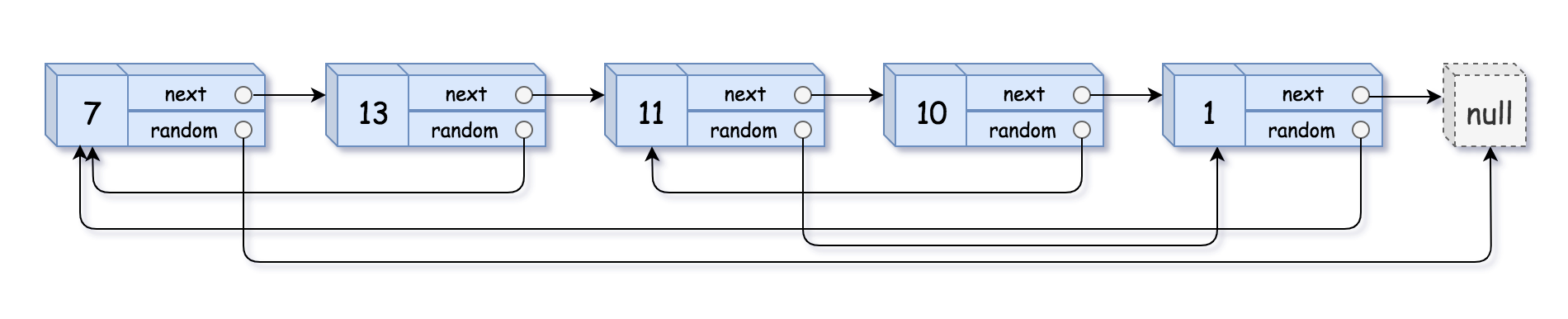输入：head = [[7,null],[13,0],[11,4],[10,2],[1,0]]
输出：[[7,null],[13,0],[11,4],[10,2],[1,0]]

示例 2：输入：head = [[1,1],[2,1]]
输出：[[1,1],[2,1]]

示例 3：输出：[[3,null],[3,0],[3,null]]
示例 4：

输出：[]
解释：给定的链表为空（空指针），因此返回 null。

提示：

-10000 <= Node.val <= 10000
Node.random 为空（null）或指向链表中的节点。
节点数目不超过 1000 。

解法
/*
// Definition for a Node.
class Node {
int val;
Node next;
Node random;

public Node(int val) {
this.val = val;
this.next = null;
this.random = null;
}
}
*/

class Solution {
return null;
}
// 将原链表每个节点旁边增加一个节点
while (ptr != null) {
Node newNode = new Node(ptr.val);
newNode.next = ptr.next;
ptr.next = newNode;
ptr = newNode.next;
}
// 将复制链表的random指向对应的位置
while (ptr != null) {
ptr.next.random = (ptr.random != null) ? ptr.random.next : null;
ptr = ptr.next.next;
}
// 将复制链表的next指向对应的位置
while (ptrOld != null) {
ptrOld.next = ptrOld.next.next;
ptrNew.next = (ptrNew.next != null) ? ptrNew.next.next : null;
ptrOld = ptrOld.next;
ptrNew = ptrNew.next;
}
}
}

剑指 Offer 52. 两个链表的第一个公共节点
[剑指 Offer 52. 两个链表的第一个公共节点](剑指 Offer 52. 两个链表的第一个公共节点)
输入两个链表，找出它们的第一个公共节点。
如下面的两个链表：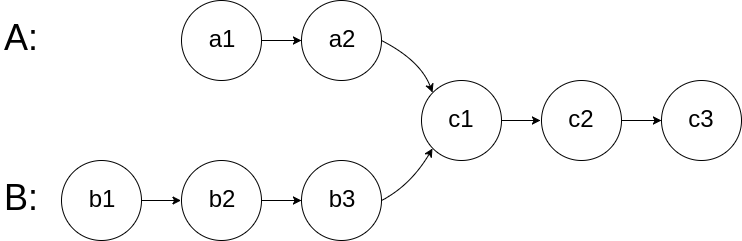在节点 c1 开始相交。
示例 1：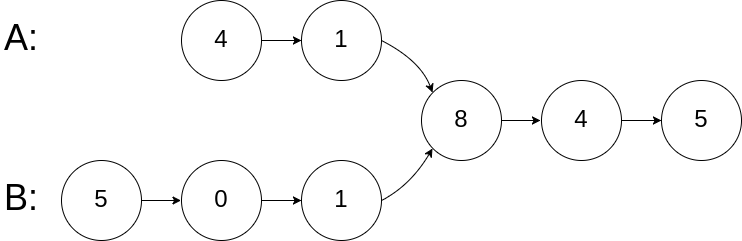输入：intersectVal = 8, listA = [4,1,8,4,5], listB = [5,0,1,8,4,5], skipA = 2, skipB = 3
输出：Reference of the node with value = 8
输入解释：相交节点的值为 8 （注意，如果两个列表相交则不能为 0）。从各自的表头开始算起，链表 A 为 [4,1,8,4,5]，链表 B 为 [5,0,1,8,4,5]。在 A 中，相交节点前有 2 个节点；在 B 中，相交节点前有 3 个节点。

示例 2：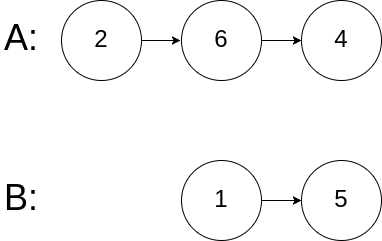输入：intersectVal = 0, listA = [2,6,4], listB = [1,5], skipA = 3, skipB = 2
输出：null
输入解释：从各自的表头开始算起，链表 A 为 [2,6,4]，链表 B 为 [1,5]。由于这两个链表不相交，所以 intersectVal 必须为 0，而 skipA 和 skipB 可以是任意值。
解释：这两个链表不相交，因此返回 null。

注意：

如果两个链表没有交点，返回 null.
在返回结果后，两个链表仍须保持原有的结构。
可假定整个链表结构中没有循环。
程序尽量满足 O(n) 时间复杂度，且仅用 O(1) 内存。

解法
A和B两个链表长度可能不同，但是A+B和B+A的长度是相同的，所以遍历A+B和遍历B+A一定是同时结束。 如果A,B相交的话A和B有一段尾巴是相同的，所以两个遍历的指针一定会同时到达交点 如果A,B不相交的话两个指针就会同时到达A+B（B+A）的尾节点。
/**
* public class ListNode {
*     int val;
*     ListNode next;
*     ListNode(int x) {
*         val = x;
*         next = null;
*     }
* }
*/
public class Solution {
while (l1 != l2) {
l1 = (l1 == null) ? headB : l1.next;
l2 = (l2 == null) ? headA : l2.next;
}
return l1;
}
}


推荐阅读

机器学习资料汇总
吴恩达《机器学习》视频、作业、源码
106页《Python进阶》中文版正式发布
李航《统计学习方法》第二版完整课件
机器学习数学全书，1900页PDF下载展开全文java 链表
• ## 剑指offer题目及答案

万次阅读 多人点赞 2017-04-06 19:07:25
剑指offer最近在牛客网上刷剑指offer的题目，现将题目和答案总结如下
剑指offer

最近在牛客网上刷剑指offer的题目，现将题目和答案总结如下：
1. 二维数组的查找
2. 替换空格
3. 从尾到头打印链表
4. 重建二叉树
5. 用两个栈实现队列
6. 旋转数组的最小数字
7. 斐波那契数列
8. 跳台阶
9. 变态跳台阶
10. 矩阵覆盖
11. 二进制中1的位数
12. 数值的整数次方
13. 调整数组顺序使奇数位于偶数前面
14. 链表中倒数第k个结点
15. 反转链表
16. 合并两个排序的链表
17. 树的子结构
18. 二叉树的镜像
19. 顺时针打印矩阵
20. 包含min函数的栈
21. 栈的压入、弹出序列
22. 从上往下打印二叉树
23. 二叉搜索树的后序遍历序列
24. 二叉树中和为某一值的路径
25. 复杂链表的复制
26. 二叉搜索树与双向链表
27. 字符串的排列
28. 数组中出现次数超过一半的数字
29. 最小的K个数
30. 连续子数组的最大和
31. 整数中1出现的次数（从1到n整数中1出现的次数）
32. 把数组排成最小的数
33. 丑数
34. 第一个只出现一次的字符
35. 数组中的逆序对
36. 两个链表的第一个公共结点
37. 数字在排序数组中出现的次数
38. 二叉树的深度
39. 平衡二叉树
40. 数组中只出现一次的数字
41. 和为S的连续正数序列
42. 和为S的两个数字
43. 左旋转字符串
44. 翻转单词顺序列
45. 扑克牌顺子
46. 孩子们的游戏(圆圈中最后剩下的数)
47. 求1+2+3+…+n
48. 不用加减乘除做加法
49. 把字符串转换成整数
50. 数组中重复的数字
51. 构建乘积数组
52. 正则表达式匹配
53. 表示数值的字符串
54. 字符流中第一个不重复的字符
55. 链表中环的入口结点
56. 删除链表中重复的结点
57. 二叉树的下一个结点
58. 对称的二叉树
59. 按之字形顺序打印二叉树
60. 把二叉树打印成多行
61. 序列化二叉树
62. 二叉搜索树的第k个结点
63. 数据流中的中位数
64. 滑动窗口的最大值
65. 矩阵中的路径
66. 机器人的运动范围

1. 二维数组的查找

在一个二维数组中，每一行都按照从左到右递增的顺序排序，每一列都按照从上到下递增的顺序排序。请完成一个函数，输入这样的一个二维数组和一个整数，判断数组中是否含有该整数。

/* 思路： 依次比较右上角的数字；如果该数字大于要查找的数字，则剔除列；如果该数字大于要查找的数字，则剔除行；
复杂度：O(m+n), 行数m，列数n */
class Solution {
public:
bool Find(int target, vector<vector<int> > array) {
bool found=false;
if (array.empty())
return found;
int rows, columns, row, column;
rows = array.size();
columns = array.size();
row = 0;
column = columns - 1;
while(row < rows && column >= 0)
{
if(array[row][column] == target)
{
found = true;
break;
}
else if (array[row][column] > target)
-- column;
else
++ row;
}
return found;
}
};

2. 替换空格

请实现一个函数，将一个字符串中的空格替换成“%20”。例如，当字符串为We Are Happy.则经过替换之后的字符串为We%20Are%20Happy。

/* 思路：首先计算原字符串长度，空格个数；然后计算替换之后的长度；设置两个指针分别指向原，新字符串的尾部，逐个赋值；
复杂度：O(n) */
class Solution {
public:
void replaceSpace(char *str,int length) {
if(str == nullptr || length <=0)
return;

int original_length = 0;
int number_of_space = 0;
int i = 0;
while(str[i] != '\0')
{
++ original_length;
if(str[i] == ' ')
++ number_of_space;
++ i;
}

if (number_of_space <= 0)
return;

int new_length = original_length + 2*number_of_space;

int index_of_original = original_length;
int index_of_new = new_length;

while(index_of_original>=0 && index_of_new>=index_of_original)
{
if(str[index_of_original] == ' ')
{
str[index_of_new--] = '0';
str[index_of_new--] = '2';
str[index_of_new--] = '%';
}
else
{
str[index_of_new--] = str[index_of_original];
}
-- index_of_original;
}

}
};

3. 从尾到头打印链表

输入一个链表，从尾到头打印链表每个节点的值。

/**
*  struct ListNode {
*        int val;
*        struct ListNode *next;
*        ListNode(int x) :
*              val(x), next(NULL) {
*        }
*  };
*/
// 思路：借助辅助栈，或者使用递归；
class Solution {
public:
vector<int> reverse_list;
stack<int> nodes;

while(p_node != nullptr)
{
nodes.push(p_node->val);
p_node = p_node->next;
}

int tempVal;
while(!nodes.empty())
{
tempVal = nodes.top();
reverse_list.push_back(tempVal);
nodes.pop();
}
return reverse_list;
}
};

4.重建二叉树

输入某二叉树的前序遍历和中序遍历的结果，请重建出该二叉树。假设输入的前序遍历和中序遍历的结果中都不含重复的数字。例如输入前序遍历序列{1,2,4,7,3,5,6,8}和中序遍历序列{4,7,2,1,5,3,8,6}，则重建二叉树并返回。

/**
* Definition for binary tree
* struct TreeNode {
*     int val;
*     TreeNode *left;
*     TreeNode *right;
*     TreeNode(int x) : val(x), left(NULL), right(NULL) {}
* };
*/
/* 思路（递归）：根据前序遍历的第一个数字创建根节点；在中序便利找到根节点的位置；确定左右子树节点数量；递归构建左右子树；*/
class Solution {
public:
TreeNode* reConstructBinaryTree(vector<int> pre,vector<int> vin) {
if(pre.empty() || vin.empty() || pre.size()!=vin.size())
return nullptr;

vector<int> left_pre, right_pre, left_vin, right_vin;
TreeNode *node = new TreeNode(pre);

int left_length = 0;
while(pre!=vin[left_length] && left_length < pre.size())
++ left_length;

for(int i=0; i<left_length; i++)
{
left_pre.push_back(pre[i+1]);
left_vin.push_back(vin[i]);
}

for(int i=left_length+1; i<pre.size(); i++)
{
right_pre.push_back(pre[i]);
right_vin.push_back(vin[i]);
}
node->left = reConstructBinaryTree(left_pre, left_vin);
node->right = reConstructBinaryTree(right_pre, right_vin);

return node;
}
};

5.用两个栈实现队列

用两个栈来实现一个队列，完成队列的Push和Pop操作。 队列中的元素为int类型。

/*思路：stack1:负责压栈，stack2负责弹栈（如果为空，则将stack1中元素压入stack2）；*/
class Solution
{
public:
void push(int node) {
stack1.push(node);
}

int pop() {
if(stack2.empty())
{
while(!stack1.empty())
{
int val = stack1.top();
stack1.pop();
stack2.push(val);
}
}
int val = stack2.top();
stack2.pop();
return val;
}

private:
stack<int> stack1;
stack<int> stack2;
};

6.  旋转数组的最小数字

把一个数组最开始的若干个元素搬到数组的末尾，我们称之为数组的旋转。
输入一个非递减排序的数组的一个旋转，输出旋转数组的最小元素。
例如数组{3,4,5,1,2}为{1,2,3,4,5}的一个旋转，该数组的最小值为1。
NOTE：给出的所有元素都大于0，若数组大小为0，请返回0。

/*简单方法*/
class Solution {
public:
int minNumberInRotateArray(vector<int> rotateArray)
{         //数组为空时
if(rotateArray.size() == 0)
return -1;
//前部分数据旋转
for(int i = 0; i < rotateArray.size() - 1; i++)
{
if (rotateArray[i] > rotateArray[i + 1])
return rotateArray[i + 1];
}
//全部数据旋转，相当于没有旋转，最小数即为第一个数
return rotateArray;
}
};

/*思路：二分查找思想*/
class Solution {
public:
int minNumberInRotateArray(vector<int> rotateArray) {
int length = rotateArray.size();
if (length == 0)
return 0;

int left = 0, right = length-1;
int mid;
while(rotateArray[left] >= rotateArray[right])
{
if(left == right - 1)
return rotateArray[right];

mid = (left + right)/2;

if(rotateArray[left] == rotateArray[mid] &&
rotateArray[mid] == rotateArray[right])
{
int min_num = rotateArray[left];
for(int i=left; i < right; i++)
min_num = rotateArray[i]<min_num? rotateArray[i]:min_num;
return min_num;
}
if(rotateArray[left] <= rotateArray[mid])
left = mid;
else
right = mid;

}
return rotateArray[left];

}
};

7.斐波那契数列

大家都知道斐波那契数列，现在要求输入一个整数n，请你输出斐波那契数列的第n项。
n<=39

/* 思路： 循环，保存中间结果（递归的话，重复计算太多）*/
class Solution {
public:
int Fibonacci(int n) {
if(n<=0)
return 0;
if(n==1)
return 1;
int fib1=1, fib2=0;
int fibn;
for(int i=2; i<=n; i++)
{
fibn = fib1+fib2;

fib2 = fib1;
fib1 = fibn;
}
return fibn;
}
};

8. 跳台阶

一只青蛙一次可以跳上1级台阶，也可以跳上2级。求该青蛙跳上一个n级的台阶总共有多少种跳法。

class Solution {
public:
int jumpFloor(int number) {
if(number == 1)
return 1;

int pre1=1, pre2=1;
int cur;
for(int i=2; i<=number; i++)
{
cur = pre1 + pre2;

pre2 = pre1;
pre1 = cur;
}

return cur;
}
};

9.变态跳台阶

一只青蛙一次可以跳上1级台阶，也可以跳上2级……它也可以跳上n级。求该青蛙跳上一个n级的台阶总共有多少种跳法

思路：其实是隔板问题，假设n个台阶，有n-1个空隙，可以用0~n-1个隔板分割，c(n-1,0)+c(n-1,1)+...+c(n-1,n-1)=2^(n-1)，其中c表示组合。 有人用移位1<<--number，这是最快的。

class Solution {
public:
int jumpFloorII(int number) {
int jump_number = 1;
for(int i=0; i<number-1; i++)
jump_number = jump_number * 2;
return jump_number;
}
};

/**********更加简单的方法**********/

class Solution {
public:
int jumpFloorII(int number) {
return 1<<(--number);
}
};


10. 矩阵覆盖

我们可以用2*1的小矩形横着或者竖着去覆盖更大的矩形。请问用n个2*1的小矩形无重叠地覆盖一个2*n的大矩形，总共有多少种方法？

/* 思路：
第一块有两种方式：横着放和竖着放
横这放对应为发f(n-2);
竖着放下一步的放方法为f(n-1);
所以总的放的方法为f(n)=f(n-1)+f(n-2);
*/

class Solution {
public:
int rectCover(int number) {
if(number <= 2)
return number;
int pre1 = 2, pre2 = 1;
int cur;
for(int i=2; i<number; i++)
{
cur = pre1 + pre2;

pre2 = pre1;
pre1 = cur;
}
return cur;
}
};

11. 二进制中1的位数

输入一个整数，输出该数二进制表示中1的个数。其中负数用补码表示。

/************ 简单方法 ************/
class Solution {
public:
int  NumberOf1(int n) {
int count = 0;
unsigned int flag = 1;
while(flag)
{
if(n & flag)
++ count;
flag = flag << 1;
}
return count;
}
};

/******* 巧妙方法 *******/
思路：一个整数减去1，在与原整数做与运算，会将最右边的一个1变成0.
那么二进制中有多少个1，可进行这样的操作多少次；
class Solution {
public:
int NumberOf1(int n) {
int count = 0;
while(n)
{
++ count;
n = (n-1)&n;
}
return count;
}
};


12. 数值的整数次方

给定一个double类型的浮点数base和int类型的整数exponent。求base的exponent次方。

/*思路 需要考虑以下几种情况
1) base的正负；
2) base是否等于0；
3) exponent的正负；
4) exponent是否为1；*/

class Solution {
public:
double Power(double base, int exponent) {
if (base>-0.0000001 && base<0.0000001 && exponent<0)
return 0.0;

double result = 1.0;

unsigned int abs_exponent = (unsigned int) exponent;
if(exponent < 0)
abs_exponent = (unsigned int) (-exponent);

/*
for(int i=0; i<abs_exponent; i++)
result = result * base;
*/
//
if(abs_exponent == 0)
return 1.0;
if(abs_exponent == 1)
return base;

result = base;
abs_exponent = abs_exponent >> 1;
while(abs_exponent)
{
result *= result;
abs_exponent = abs_exponent >> 1;
}
if(exponent & 0x1 == 1)
result *= base;
//
if(exponent < 0 && result > 0.0)
result = 1.0 / result;
return result;
}
};

13. 调整数组顺序使奇数位于偶数前面

输入一个整数数组，实现一个函数来调整该数组中数字的顺序，使得所有的奇数位于数组的前半部分，所有的偶数位于位于数组的后半部分，并保证奇数和奇数，偶数和偶数之间的相对位置不变。

注：相比剑指offer书要难（要保证相对顺序不变）
class Solution {
public:
void reOrderArray(vector<int> &array) {
int length = array.size();
if(length==0 || length==1)
return;

int index_even=0, index_odd;
while(index_even<length)
{
while(index_even<length && !isEven(array[index_even]))
++ index_even;
index_odd = index_even+1;
while(index_odd<length && isEven(array[index_odd]))
++ index_odd;

if(index_odd<length)
{
int temp = array[index_odd];
for(int i=index_odd; i>index_even; i--)
array[i] = array[i-1];
array[index_even] = temp;
}
else
break;
}

}

bool isEven(int number){
if((number & 0x1) == 0)
return true;
return false;
}

};

/*************方法二 申请空间***********/
class Solution {
public:
void reOrderArray(vector<int> &array) {
int length = array.size();
if(length==0 || length ==1)
return;

vector<int> res;
for(int i=0; i<length; i++)
{
if((array[i]&0x1) != 0)
res.push_back(array[i]);
}

for(int i=0; i<length; i++)
{
if((array[i]&0x1) == 0)
res.push_back(array[i]);
}
array = res;
}
};

14. 链表中倒数第k个结点

输入一个链表，输出该链表中倒数第k个结点。

/*
struct ListNode {
int val;
struct ListNode *next;
ListNode(int x) :
val(x), next(NULL) {
}
};*/
// 定义快慢指针，快的先走K步；
class Solution {
public:
ListNode* FindKthToTail(ListNode* pListHead, unsigned int k) {
return nullptr;

for(int i=0; i<k-1; i++)
{
else
return nullptr;
}

{
pAfter = pAfter->next;
}

return pAfter;

}
};

15. 反转链表

输入一个链表，反转链表后，输出链表的所有元素。

/*
struct ListNode {
int val;
struct ListNode *next;
ListNode(int x) :
val(x), next(NULL) {
}
};*/
/* 思路：定义三个指针，分别指向当前结点，前一个结点，后一个结点 */
class Solution {
public:
return nullptr;

pPreNode->next = nullptr;
while(pCurNode->next != nullptr)
{
pNextNode = pCurNode->next;

pCurNode->next = pPreNode;
pPreNode = pCurNode;
pCurNode = pNextNode;
}
pCurNode->next = pPreNode;
return pCurNode;
}
};

16.合并两个排序的链表

输入两个单调递增的链表，输出两个链表合成后的链表，当然我们需要合成后的链表满足单调不减规则。

/*
struct ListNode {
int val;
struct ListNode *next;
ListNode(int x) :
val(x), next(NULL) {
}
};*/

/*------------------------------方法一 递归版本--------------------------*/
class Solution {
public:
{

ListNode *pMerge;
{
}
else
{
}
return pMerge;
}
};


17. 树的子结构

输入两棵二叉树A，B，判断B是不是A的子结构。（ps：我们约定空树不是任意一个树的子结构）

/*
struct TreeNode {
int val;
struct TreeNode *left;
struct TreeNode *right;
TreeNode(int x) :
val(x), left(NULL), right(NULL) {
}
};*/

/*分两步，判断根节点是否相等；判断子结构是否相等*/
class Solution {
public:
bool HasSubtree(TreeNode* pRoot1, TreeNode* pRoot2)
{
bool result = false;
if(pRoot1!=nullptr && pRoot2!=nullptr)
{
if(pRoot1->val == pRoot2->val)
result = DoesTree1HaveTree2(pRoot1, pRoot2);
if(!result)
result = HasSubtree(pRoot1->left, pRoot2);
if(!result)
result = HasSubtree(pRoot1->right, pRoot2);
}
return result;
}

bool DoesTree1HaveTree2(TreeNode *Tree1, TreeNode *Tree2)
{
if(Tree2 == nullptr)
return true;
if(Tree1 == nullptr)
return false;
if(Tree1->val != Tree2->val)
return false;

return DoesTree1HaveTree2(Tree1->left, Tree2->left) &&
DoesTree1HaveTree2(Tree1->right, Tree2->right);
}
};

18. 二叉树的镜像

操作给定的二叉树，将其变换为源二叉树的镜像。

/*
struct TreeNode {
int val;
struct TreeNode *left;
struct TreeNode *right;
TreeNode(int x) :
val(x), left(NULL), right(NULL) {
}
};*/
/* 思路：相当于树的遍历 */
/*-------------- 递归方法 ------------*/
class Solution {
public:
void Mirror(TreeNode *pRoot) {
if(pRoot == nullptr || (pRoot->left==nullptr && pRoot->right==nullptr))
return;

if(pRoot->left != nullptr)
Mirror(pRoot->left);
if(pRoot->right != nullptr)
Mirror(pRoot->right);
TreeNode *temp = pRoot->left;
pRoot->left = pRoot->right;
pRoot->right = temp;
}
};

/*------------- 使用栈 ------------------*/
class Solution {
public:
void Mirror(TreeNode *pRoot) {
if(pRoot == nullptr || (pRoot->left==nullptr && pRoot->right==nullptr))
return;

stack<TreeNode*> stackNodes;
stackNodes.push(pRoot);

while(stackNodes.size() > 0)
{
TreeNode *pNode = stackNodes.top();
stackNodes.pop();

TreeNode *pTemp = pNode->left;
pNode->left = pNode->right;
pNode->right = pTemp;

if(pNode->left != nullptr)
stackNodes.push(pNode->left);
if(pNode->right != nullptr)
stackNodes.push(pNode->right);
}

}
};

19. 顺时针打印矩阵

输入一个矩阵，按照从外向里以顺时针的顺序依次打印出每一个数字，例如，如果输入如下矩阵： 1 2 3 4 5 6 7 8 9 10 11 12 13 14 15 16 则依次打印出数字1,2,3,4,8,12,16,15,14,13,9,5,6,7,11,10.

class Solution {
public:
vector<int> printMatrix(vector<vector<int> > matrix) {
int rows, columns;
if(matrix.size()>0)
{
rows = matrix.size();
columns = matrix.size();
}

vector<int> result;

int startX = 0, endX = columns-1;
int startY = 0, endY = rows-1;
while(startX <= endX && startY<=endY)
{
if(startX<=endX && startY<=endY)
{
for(int i=startX; i<=endX; i++)
result.push_back(matrix[startY][i]);
++ startY;
}

if(startY<=endY && startX<=endX)
{
for(int i=startY; i<=endY; i++)
result.push_back(matrix[i][endX]);
-- endX;
}
if(startX<=endX && startY<=endY)
{
for(int i=endX; i>=startX; i--)
result.push_back(matrix[endY][i]);
-- endY;
}
if(startY<=endY && startX<=endX)
{
for(int i=endY; i>=startY; i--)
result.push_back(matrix[i][startX]);
++ startX;
}
}
return result;
}
};

20. 包含min函数的栈

定义栈的数据结构，请在该类型中实现一个能够得到栈最小元素的min函数。

/* 借助辅助栈，保存每次压栈之后的最小值 */
class Solution {
public:
void push(int value) {
if(s_data.empty()){
s_data.push(value);
s_min.push(value);
}
else{
s_min.push(value<s_min.top()?value:s_min.top());
s_data.push(value);
}s
}
void pop() {
s_data.pop();
s_min.pop();
}
int top() {
return s_data.top();
}
int min() {
return s_min.top();
}
private:
stack<int> s_data;
stack<int> s_min;
};

21. 栈的压入、弹出序列

输入两个整数序列，第一个序列表示栈的压入顺序，请判断第二个序列是否为该栈的弹出顺序。假设压入栈的所有数字均不相等。例如序列1,2,3,4,5是某栈的压入顺序，序列4，5,3,2,1是该压栈序列对应的一个弹出序列，但4,3,5,1,2就不可能是该压栈序列的弹出序列。（注意：这两个序列的长度是相等的）

/* 辅助栈：模拟整个过程 */
class Solution {
public:
bool IsPopOrder(vector<int> pushV,vector<int> popV) {
int length = pushV.size();
int length2 = popV.size();
if(length<=0 || length2<=0 || (length!=length2))
return false;

stack<int> stackV;
int index_push = 0, index_pop=0;
while(index_pop < length)
{
while(stackV.empty() || stackV.top()!=popV[index_pop])
{
if(index_push == length)
break;
stackV.push(pushV[index_push++]);
}
if(stackV.top() != popV[index_pop])
break;
++ index_pop;
stackV.pop();
}
if(stackV.empty() && index_pop==length)
return true;
else
return false;
}
};

22. 从上往下打印二叉树

从上往下打印出二叉树的每个节点，同层节点从左至右打印。

/*
struct TreeNode {
int val;
struct TreeNode *left;
struct TreeNode *right;
TreeNode(int x) :
val(x), left(NULL), right(NULL) {
}
};*/
/* 思路：辅助队列 */
class Solution {
public:
vector<int> PrintFromTopToBottom(TreeNode* root){
vector<int> result;
if(root == nullptr)
return result;

queue<TreeNode *> nodes;
nodes.push(root);
while(!nodes.empty())
{
TreeNode *pNode = nodes.front();
result.push_back(pNode->val);

if(pNode->left != nullptr)
nodes.push(pNode->left);
if(pNode->right != nullptr)
nodes.push(pNode->right);

nodes.pop();
}

return result;
}
};

23. 二叉搜索树的后序遍历序列

输入一个整数数组，判断该数组是不是某二叉搜索树的后序遍历的结果。如果是则输出Yes,否则输出No。假设输入的数组的任意两个数字都互不相同。

/* 递归判断：左子树<根节点<右子树，不满足则为false，否则为true； */
class Solution {
public:
bool VerifySquenceOfBST(vector<int> sequence) {
if(sequence.size()<=0)
return false;
int start=0, end=sequence.size()-1;
return isLastOrder(sequence, start, end);
}

private:
bool isLastOrder(vector<int> &sequence, int start, int end)
{
if(start > end)
return false;

int root = sequence[end];
int i = start;
for(; i<end; i++)
{
if(sequence[i]>root)
break;
}
int j = i;
for(; j<end; j++)
{
if(sequence[j]<root)
return false;
}
bool left = true;
if(i-1 > start)
left = isLastOrder(sequence, start, i-1);

bool right = true;
if(i < end-1)
right = isLastOrder(sequence, i, end-1);

return(left && right);
}

};

24. 二叉树中和为某一值的路径

输入一颗二叉树和一个整数，打印出二叉树中结点值的和为输入整数的所有路径。路径定义为从树的根结点开始往下一直到叶结点所经过的结点形成一条路径。

/*
struct TreeNode {
int val;
struct TreeNode *left;
struct TreeNode *right;
TreeNode(int x) :
val(x), left(NULL), right(NULL) {
}
};*/
/* 回溯法，终止条件是为叶子节点，且值相等； */
class Solution {
public:
vector<vector<int> > FindPath(TreeNode* root,int expectNumber) {
vector<vector<int>> pathes;
if(root==nullptr)
return pathes;

vector<int> onePath;
int curSum = 0;
findPath(root, pathes, onePath, expectNumber, curSum);

return pathes;
}
private:
void findPath(TreeNode *pRoot,vector<vector<int>> &pathes, vector<int> onePath, int expectNumber, int &curSum)
{
curSum += pRoot->val;
onePath.push_back(pRoot->val);

bool isLeaf = false;
if(pRoot->left==nullptr && pRoot->right==nullptr)
isLeaf = true;
if(isLeaf && curSum==expectNumber)
{
pathes.push_back(onePath);
}

if(pRoot->left != nullptr)
findPath(pRoot->left, pathes, onePath, expectNumber, curSum);
if(pRoot->right != nullptr)
findPath(pRoot->right, pathes, onePath, expectNumber, curSum);

curSum -=pRoot->val;
onePath.pop_back();
}
};

25. 复杂链表的复制

/*
struct RandomListNode {
int label;
struct RandomListNode *next, *random;
RandomListNode(int x) :
label(x), next(NULL), random(NULL) {
}
};
*/
/*分为三步：
1）在旧链表中创建新链表，此时不处理新链表的兄弟节点 ；
2）根据旧链表的兄弟节点，初始化新链表的兄弟节点；
3）从旧链表中拆分得到新链表；*/
class Solution {
public:
{
return nullptr;
// step.1 Copy Node
while(pNode!=nullptr)
{
RandomListNode* pCloned = new RandomListNode(pNode->label);
pCloned->next = pNode->next;
// newNode->random = nullptr;

pNode->next = pCloned;
pNode = pCloned->next;
}

// step.2 Copy random
while(pNode!=nullptr)
{
RandomListNode *pCloned = pNode->next;
if(pNode->random != nullptr)
pCloned->random = pNode->random->next;
pNode = pCloned->next;
}

// step.3 Split

if(pNode!=nullptr)
{
pNode->next = pCloneNode->next;
pNode = pNode->next;
}

while(pNode != nullptr)
{
pCloneNode->next = pNode->next;
pCloneNode = pCloneNode->next;
pNode->next = pCloneNode->next;
pNode = pNode->next;
}

}
};

26. 二叉搜索树与双向链表

输入一棵二叉搜索树，将该二叉搜索树转换成一个排序的双向链表。要求不能创建任何新的结点，只能调整树中结点指针的指向。

/*
struct TreeNode {
int val;
struct TreeNode *left;
struct TreeNode *right;
TreeNode(int x) :
val(x), left(NULL), right(NULL) {
}
};*/
/*解题思路：递归版
1.将左子树构造成双链表，并返回链表头节点。2.定位至左子树双链表最后一个节点。3.如果左子树链表不为空的话，将当前root追加到左子树链表。4.将右子树构造成双链表，并返回链表头节点。5.如果右子树链表不为空的话，将该链表追加到root节点之后。6.根据左子树链表是否为空确定返回的节点。*/
class Solution {
public:
TreeNode* Convert(TreeNode* pRootOfTree)
{
if(pRootOfTree==nullptr)
return nullptr;

pRootOfTree = ConvertNode(pRootOfTree);
while(pRootOfTree->left!=nullptr)
pRootOfTree = pRootOfTree->left;

return pRootOfTree;
}

TreeNode* ConvertNode(TreeNode *pRoot)
{
if(pRoot==nullptr)
return nullptr;
if(pRoot->left!=nullptr)
{
TreeNode *left = ConvertNode(pRoot->left);
while(left->right!=nullptr)
left = left->right;
pRoot->left = left;
left->right = pRoot;
}
if(pRoot->right != nullptr)
{
TreeNode *right=ConvertNode(pRoot->right);
while(right->left!=nullptr)
right = right->left;

pRoot->right = right;
right->left = pRoot;
}
return pRoot;
}
};

27. 字符串的排列

输入一个字符串,按字典序打印出该字符串中字符的所有排列。例如输入字符串abc,则打印出由字符a,b,c所能排列出来的所有字符串abc,acb,bac,bca,cab和cba。

/*全排列问题*/
class Solution {
public:
vector<string> Permutation(string str) {
vector<string> result;
if(str.size()<=0)
return result;
PermutationCore(result, str, 0);
sort(result.begin(), result.end());
return result;
}

void PermutationCore(vector<string> &result, string str, int begin)
{
if(begin == str.size()-1)
result.push_back(str);
else
{
for(int i=begin; i<str.size(); i++)
{
if(i!=begin && str[i]==str[begin])
continue;
swap(str[i], str[begin]);
PermutationCore(result, str, begin+1);
swap(str[i], str[begin]);
}
}
}
};

28. 数组中出现次数超过一半的数字

数组中有一个数字出现的次数超过数组长度的一半，请找出这个数字。例如输入一个长度为9的数组{1,2,3,2,2,2,5,4,2}。由于数字2在数组中出现了5次，超过数组长度的一半，因此输出2。如果不存在则输出0。

/*一个思路是基于快排中partition（会修改数组中的值）；
还有就是：定义一个times记录当前牟数字出现的次数，如果小于0则替换；
复杂度都是O(n)
*/
class Solution {
public:
int MoreThanHalfNum_Solution(vector<int> numbers) {
if(numbers.size() <= 0)
return 0;
int result = numbers;
int times = 1;

for(int i=0; i<numbers.size(); i++)
{
if(times==0)
{
result = numbers[i];
times = 1;
}
else if(times>0 && result==numbers[i])
++ times;
else
-- times;
}
if(!isMoreThanHalf(numbers, result))
return 0;

return result;

}

private:
bool isMoreThanHalf(vector<int> numbers, int result)
{
int times = 0;
for(int i=0; i<numbers.size(); i++)
if(numbers[i] == result)
++ times;
if(2*times <= numbers.size())
return false;
return true;
}
};

29. 最小的K个数

输入n个整数，找出其中最小的K个数。例如输入4,5,1,6,2,7,3,8这8个数字，则最小的4个数字是1,2,3,4,。

/*复杂度为O(n logn)*/
class Solution {
public:
vector<int> GetLeastNumbers_Solution(vector<int> input, int k) {
vector<int> result;
if(input.size()<k || k<1)
return result;

sort(input.begin(),input.end());

for(int i=0; i<k; i++)
result.push_back(input[i]);
return result;
}
};

/*复杂度为O(nlogk), 基于红黑树*/
class Solution {
public:
vector<int> GetLeastNumbers_Solution(vector<int> input, int k) {
if(input.size()<k || k<0)
return vector<int> ();

multiset<int, greater<int>> leastNumbers;
vector<int>::const_iterator iter=input.begin();
for(; iter!=input.end(); iter++)
{
if(leastNumbers.size() < k)
leastNumbers.insert(*iter);
else
{
multiset<int, greater<int>>::iterator iterGreatest=leastNumbers.begin();
if(*iter < *iterGreatest)
{
leastNumbers.erase(*iterGreatest);
leastNumbers.insert(*iter);
}
}
}
return vector<int>(leastNumbers.begin(), leastNumbers.end());
}
};

30. 连续子数组的最大和

HZ偶尔会拿些专业问题来忽悠那些非计算机专业的同学。今天测试组开完会后,他又发话了:在古老的一维模式识别中,常常需要计算连续子向量的最大和,当向量全为正数的时候,问题很好解决。但是,如果向量中包含负数,是否应该包含某个负数,并期望旁边的正数会弥补它呢？例如:{6,-3,-2,7,-15,1,2,2},连续子向量的最大和为8(从第0个开始,到第3个为止)。你会不会被他忽悠住？(子向量的长度至少是1)

/* 思路：动态规划复杂度为O(n)， 首先定义一个值保存当前最大值；
如果当前和为负数，直接舍弃；如果不为负数，则累加；得到 当前和 与 当前最大值 比较*/
class Solution {
public:
int FindGreatestSumOfSubArray(vector<int> array) {
if(array.size() < 1)
return 0;

int curSum = array;
int greatestSum = array;
for(int i=1; i<array.size(); i++)
{
if(curSum < 0)
curSum = array[i];
else
curSum += array[i];

if(greatestSum < curSum)
greatestSum = curSum;
}
return greatestSum;
}
};

31. 整数中1出现的次数（从1到n整数中1出现的次数）

求出1~13的整数中1出现的次数,并算出100~1300的整数中1出现的次数？为此他特别数了一下1~13中包含1的数字有1、10、11、12、13因此共出现6次,但是对于后面问题他就没辙了。ACMer希望你们帮帮他,并把问题更加普遍化,可以很快的求出任意非负整数区间中1出现的次数。

/*思路：穷举*/
class Solution {
public:
int NumberOf1Between1AndN_Solution(int n)
{
if(n < 1)
return 0;

int count=0;
for(int i=1; i<=n; i++)
{
count += NumberOfN(i);
}
return count;
}
int NumberOfN(int n)
{
int count=0;
while(n)
{
if(n%10 == 1)
count += 1;
n = n/10;
}
return count;
}

};

32. 把数组排成最小的数

输入一个正整数数组，把数组里所有数字拼接起来排成一个数，打印能拼接出的所有数字中最小的一个。例如输入数组{3，32，321}，则打印出这三个数字能排成的最小数字为321323。

/*思路：通过字符串解决大数问题，然后通过自定义的字符串比较规则，对字符串排序*/
class Solution {
public:
string PrintMinNumber(vector<int> numbers) {
if(numbers.size()<1)
return string();

string result;
vector<string> numberString;
for(int i=0; i<numbers.size(); i++)
{
stringstream ss;
ss<<numbers[i];
string s = ss.str();
numberString.push_back(s);
}
sort(numberString.begin(), numberString.end(), Compare);

for(int i=0; i<numberString.size(); i++)
result.append(numberString[i]);

return result;
}

static bool Compare(const string &str1, const string &str2)
{
string s1 = str1+str2;
string s2 = str2+str1;
return s1<s2;
}
};

33. 丑数

把只包含因子2、3和5的数称作丑数（Ugly Number）。例如6、8都是丑数，但14不是，因为它包含因子7。 习惯上我们把1当做是第一个丑数。求按从小到大的顺序的第N个丑数。

/* 思路:创建数组保存已经找到的丑数，空间换时间； */
class Solution {
public:
int GetUglyNumber_Solution(int index) {
if(index <= 0)
return 0;

vector<int> res(index);
res = 1;
int t2=0, t3=0, t5=0;

for(int i=1; i<index; i++)
{
res[i] = min(2*res[t2], min(3*res[t3], 5*res[t5]));

while(res[i] >= 2*res[t2]) ++t2;
while(res[i] >= 3*res[t3]) ++t3;
while(res[i] >= 5*res[t5]) ++t5;
}
return res[index-1];
}
};

34. 第一个只出现一次的字符

在一个字符串(1<=字符串长度<=10000，全部由大写字母组成)中找到第一个只出现一次的字符,并返回它的位置。

/*思路：借助哈希表，但是空间复杂度为O(1)，时间复杂度为O(n); */
class Solution {
public:
int FirstNotRepeatingChar(string str) {
if(str.size()<=0)
return -1;
int tableSize = 256;
vector<int> numOfChar(tableSize, 0);

for(int i=0; i<str.size(); i++)
++numOfChar[str[i]];

for(int i=0; i<str.size(); i++)
if(numOfChar[str[i]]==1 && str[i]!='\0')
return i;

return -1;
}
};

35.数组中的逆序对

在数组中的两个数字，如果前面一个数字大于后面的数字，则这两个数字组成一个逆序对。输入一个数组,求出这个数组中的逆序对的总数P。并将P对1000000007取模的结果输出。 即输出P%1000000007

/* 思路：归并排序的改进，把数据分成前后两个数组(递归分到每个数组仅有一个数据项)，合并数组，合并时，出现前面的数组值array[i]大于后面数组值array[j]时；则前面数组array[i]~array[mid]都是大于array[j]的，count += mid+1 - i参考剑指Offer，但是感觉剑指Offer归并过程少了一步拷贝过程。还有就是测试用例输出结果比较大，对每次返回的count mod(1000000007)求余 */
class Solution {
public:
int InversePairs(vector<int> data) {
int length = data.size();
if(length <= 0)
return 0;
int count = MergeSort(data, 0, length-1);
return count % 1000000007;
}

int MergeSort(vector<int> &data, int start, int end)
{
if(start >= end)
return 0;

int mid = (start+end)/2;
int left = MergeSort(data, start, mid);
int right = MergeSort(data, mid+1, end);

vector<int> copy(data);
int i = mid;
int j = end;
int counts = 0;
int indexCopy = end;
while(i>=start && j>=mid+1)
{
if(data[i] > data[j])
{
copy[indexCopy--] = data[i--];
counts += (j - mid);
}
else
copy[indexCopy--] = data[j--];
}

while(i >= start)
copy[indexCopy--] = data[i--];
while(j >= mid+1)
copy[indexCopy--] = data[j--];

for(int k=start; k<=end; k++)
data[k] = copy[k];

return left+right+counts;
}
};

36. 两个链表的第一个公共结点

输入两个链表，找出它们的第一个公共结点。


/*
struct ListNode {
int val;
struct ListNode *next;
ListNode(int x) :
val(x), next(NULL) {
}
};*/
/*思路：统计两个链表的长度，计算差值k，定义快慢指针，长链表先走k步*/
class Solution {
public:
return nullptr;

int diff = length1-length2;
if(length1 < length2)
{
diff = length2-length1;
}

for(int i=0; i<diff; i++)

{
pBehind = pBehind->next;
}

return nullptr;
}

private:
{
return 0;
int length = 0;
{
++ length;
}
return length;
}

};


37.数字在排序数组中出现的次数

统计一个数字在排序数组中出现的次数。

/*思路：基于二分查找复杂度为O(logn)；二分查找开始位置，二分查找结尾位置，做差；*/
class Solution {
public:
int GetNumberOfK(vector<int> data ,int k) {
int length = data.size();
if(length <= 0)
return 0;

int first = firstIndex(data, k);
int last = lastIndex(data, k);
if(first > -1 && last > -1)
return last-first+1;

return 0;
}

int firstIndex(vector<int> data, int k)
{
int low = 0;
int high = data.size()-1;
int midIndex, midData;
while(low <= high)
{
midIndex = (low+high)/2;
midData = data[midIndex];

if(midData == k)
{
if(midIndex == 0 || data[midIndex-1] != k)
return midIndex;
else
high = midIndex-1;
}
else if(midData > k)
high = midIndex-1;
else
low = midIndex+1;
}
return -1;
}

int lastIndex(vector<int> data, int k)
{
int low = 0;
int high = data.size()-1;
int midIndex, midData;
while(low <= high)
{
midIndex = (low+high)/2;
midData = data[midIndex];

if(midData == k)
{
if(midIndex == data.size()-1 || data[midIndex+1] != k)
return midIndex;
else
low = midIndex+1;
}
else if(midData > k)
high = midIndex-1;
else
low = midIndex+1;
}
return -1;
}
};

38.二叉树的深度

输入一棵二叉树，求该树的深度。从根结点到叶结点依次经过的结点（含根、叶结点）形成树的一条路径，最长路径的长度为树的深度。

/********** 递归版本 **********/
class Solution {
public:
int TreeDepth(TreeNode* pRoot)
{
if(pRoot == nullptr)
return 0;
int left = TreeDepth(pRoot->left);
int right = TreeDepth(pRoot->right);

return left>=right?(left+1):(right+1);
}
};

/********** 循环版本 **********/
class Solution {
public:
int TreeDepth(TreeNode* pRoot)
{
queue<TreeNode*> q;
if(!pRoot)
return 0;
q.push(pRoot);
int level=0;
while(!q.empty())
{
int len=q.size();
level++;
while(len--)
{
TreeNode *tmp=q.front();
q.pop();
if(tmp->left)
q.push(tmp->left);
if(tmp->right)
q.push(tmp->right);
}
}
return level;
}
};


39.平衡二叉树

输入一棵二叉树，判断该二叉树是否是平衡二叉树。

/* 递归判断左右子树的方法重复计算太多；
下面的方法相当于从叶节点向上遍历，只需要遍历一次。记录每个结点到叶节点的长度； */
class Solution {
public:
bool IsBalanced_Solution(TreeNode* pRoot) {
if(pRoot == nullptr)
return true;
int depth=0;
return IsBalanced(pRoot, &depth);
}

private:
bool IsBalanced(TreeNode *pRoot, int *depth)
{
if(pRoot == nullptr)
{
*depth = 0;
return true;
}
int left, right;
if(IsBalanced(pRoot->left, &left) && IsBalanced(pRoot->right, &right))
{
int diff = left - right;
if(diff <= 1 && diff >= -1)
{
*depth = left>right?(left+1):(right+1);
return true;
}
}
return false;
}
};

40. 数组中只出现一次的数字

一个整型数组里除了两个数字之外，其他的数字都出现了两次。请写程序找出这两个只出现一次的数字。

/*思路：可以用位运算实现，如果将所有所有数字相异或，则最后的结果肯定是那两个只出现一次的数字异或的结果；
所以根据异或的结果1所在的最低位，把数字分成两半，每一半里都还有只出现一次的数据和成对出现的数据；
这样继续对每一半相异或则可以分别求出两个只出现一次的数字*/
class Solution {
public:
void FindNumsAppearOnce(vector<int> data,int* num1,int *num2) {
int length = data.size();
if(length < 2)
return;

int xorNumber = 0;
for(int i=0; i<length; i++)
xorNumber ^= data[i];

int indexOf1 = findFirstBitIs1(xorNumber);

*num1 = 0;
*num2 = 0;
for(int i=0; i<length; i++)
{
if(isBit1(data[i], indexOf1))
*num1 ^= data[i];
else
*num2 ^= data[i];
}
}

int findFirstBitIs1(int number)
{
int indexBit = 0;
while((number&1)==0 && indexBit<8*sizeof(int))
{
number = number >> 1;
++ indexBit;
}
return indexBit;
}

bool isBit1(int number, int indexBit)
{
number = number >> indexBit;
return (number & 1);
}
};

41.和为S的连续正数序列

输出所有和为S的连续正数序列。序列内按照从小至大的顺序，序列间按照开始数字从小到大的顺序
(小明很喜欢数学,有一天他在做数学作业时,要求计算出9~16的和,他马上就写出了正确答案是100。但是他并不满足于此,他在想究竟有多少种连续的正数序列的和为100(至少包括两个数)。没多久,他就得到另一组连续正数和为100的序列:18,19,20,21,22。现在把问题交给你,你能不能也很快的找出所有和为S的连续正数序列? Good Luck! )

/*用两个数字small和big分别表示序列的最大值和最小值，首先将small初始化为1，end初始化为2.
如果从small到big的和大于s，我们就从序列中去掉较小的值(即增大small)
相反，只需要增大big。终止条件为：一直增加small到(1+sum)/2并且big小于sum为止*/
class Solution {
public:
vector<vector<int> > FindContinuousSequence(int sum) {
vector<vector<int> > result;
if(sum < 3)
return result;

int small = 1;
int big = 2;
int middle = (1+sum)/2;
int curSum = small + big;
while(small < middle) //这里一定是小于，不能是小于等于
{
if(curSum == sum)
insertIntoResult(result, small, big);

while(curSum > sum && small < middle)
{
curSum -= small;
++ small;
if(curSum == sum)
insertIntoResult(result, small, big);
}
++ big;
curSum += big;
}
return result;
}

private:
void insertIntoResult(vector<vector<int> > &result, int small, int big)
{
vector<int> tmpSeq;
for(int i=small; i<=big; i++)
tmpSeq.push_back(i);

result.push_back(tmpSeq);
}
};


42.和为S的两个数字

输入一个递增排序的数组和一个数字S，在数组中查找两个数，是的他们的和正好是S，如果有多对数字的和等于S，输出两个数的乘积最小的。
输出描述: 对应每个测试案例，输出两个数，小的先输出。


/*思路：数列满足递增，设两个头尾两个指针i和j；
若ai + aj == sum，就是答案（相差越远乘积越小）
若ai + aj > sum，j -= 1
若ai + aj < sum，i += 1 */
class Solution {
public:
vector<int> FindNumbersWithSum(vector<int> array,int sum) {
vector<int> result;
int length = array.size();
if(length < 2)
return result;
int start = 0;
int end = length - 1;
// sort(array.begin(), array.end());
while(start < end)
{
int curSum = array[start]+array[end];
if(curSum == sum)
{
result.push_back(array[start]);
result.push_back(array[end]);
return result;
}
else if(curSum < sum)
++ start;
else
-- end;
}
return result;
}
};

43.左旋转字符串

汇编语言中有一种移位指令叫做循环左移（ROL），现在有个简单的任务，就是用字符串模拟这个指令的运算结果。对于一个给定的字符序列S，请你把其循环左移K位后的序列输出。例如，字符序列S=”abcXYZdef”,要求输出循环左移3位后的结果，即“XYZdefabc”。是不是很简单？OK，搞定它！

/* 思路：三次翻转 */
class Solution {
public:
string LeftRotateString(string str, int n) {
int len = str.size();
if(len < 0 || len < n)
return str;
for(int i=0, j=n-1; i<j; i++, j--)
swap(str[i], str[j]);
for(int i=n, j=len-1; i<j; i++, j--)
swap(str[i], str[j]);
for(int i=0, j=len-1; i<j; i++, j--)
swap(str[i], str[j]);
return str;
}
};

/*************** 也可以写函数 **************/

class Solution {
public:
string LeftRotateString(string str, int n) {
int len = str.size();
if(len < 0 || len < n)
return str;

reverseString(str, 0, n-1);
reverseString(str, n, len-1);
reverseString(str, 0, len-1);

return str;
}
void reverseString(string &str, int start, int end)
{
for(int i=start, j=end; i<j; i++, j--)
swap(str[i], str[j]);
}
};


44.翻转单词顺序列

牛客最近来了一个新员工Fish，每天早晨总是会拿着一本英文杂志，写些句子在本子上。同事Cat对Fish写的内容颇感兴趣，有一天他向Fish借来翻看，但却读不懂它的意思。例如，“student. a am I”。后来才意识到，这家伙原来把句子单词的顺序翻转了，正确的句子应该是“I am a student.”。Cat对一一的翻转这些单词顺序可不在行，你能帮助他么？

/* 思路：两次翻转 */
class Solution {
public:
string ReverseSentence(string str) {
int len = str.size();
if(len <= 0)
return str;
reverseString(str, 0, len-1);

int i = 0, j = 0;
while(j <= len)
{
if(str[j]==' ' || j==len)
{
reverseString(str, i, j-1);
i = j + 1;
j = i + 1;
}
else
++ j;
}
return str;
}

void reverseString(string &str, int start, int end)
{
for(int i=start, j=end; i<j; i++, j--)
swap(str[i], str[j]);
}
};

45.扑克牌顺子

LL今天心情特别好,因为他去买了一副扑克牌,发现里面居然有2个大王,2个小王(一副牌原本是54张^_^)…他随机从中抽出了5张牌,想测测自己的手气,看看能不能抽到顺子,如果抽到的话,他决定去买体育彩票,嘿嘿！！“红心A,黑桃3,小王,大王,方片5”,“Oh My God!”不是顺子…..LL不高兴了,他想了想,决定大\小 王可以看成任何数字,并且A看作1,J为11,Q为12,K为13。上面的5张牌就可以变成“1,2,3,4,5”(大小王分别看作2和4),“So Lucky!”。LL决定去买体育彩票啦。 现在,要求你使用这幅牌模拟上面的过程,然后告诉我们LL的运气如何。为了方便起见,你可以认为大小王是0。

/*
1.对数组排序；
2.统计0的个数；
3.统计间隔数；
4.对比nZ，nG；
*/
class Solution {
public:
bool IsContinuous( vector<int> numbers ) {
int length = numbers.size();
if(length < 5)
return false;

sort(numbers.begin(), numbers.end());

int i, numberOfZero = 0, numberOfGap = 0;
for(i=0; i<length && numbers[i]==0; i++)
++ numberOfZero;

int small=i, big=small+1;
while(big < length)
{
if(numbers[small] == numbers[big])
return false;
numberOfGap += numbers[big]-numbers[small]-1;
++ small;
++ big;
}
return numberOfZero>=numberOfGap?true:false;
}
};

46.孩子们的游戏(圆圈中最后剩下的数)

每年六一儿童节,牛客都会准备一些小礼物去看望孤儿院的小朋友,今年亦是如此。HF作为牛客的资深元老,自然也准备了一些小游戏。其中,有个游戏是这样的:首先,让小朋友们围成一个大圈。然后,他随机指定一个数m,让编号为0的小朋友开始报数。每次喊到m-1的那个小朋友要出列唱首歌,然后可以在礼品箱中任意的挑选礼物,并且不再回到圈中,从他的下一个小朋友开始,继续0…m-1报数….这样下去….直到剩下最后一个小朋友,可以不用表演,并且拿到牛客名贵的“名侦探柯南”典藏版(名额有限哦!!^_^)。请你试着想下,哪个小朋友会得到这份礼品呢？(注：小朋友的编号是从0到n-1)

/*
思路一：用STL中std::list来模拟这个环形列表。由于list并不是一个环形的结构，因此每次跌代器扫描到列表末尾的时候，要记得把跌代器移到列表的头部。这样就是按照一个圆圈的顺序来遍历这个列表了。这种思路需要一个有n个结点的环形列表来模拟这个删除的过程，因此内存开销为O(n)。而且这种方法每删除一个数字需要m步运算，总共有n个数字，因此总的时间复杂度是O(mn)；
思路二：数学归纳法
{  0                  n=1
f(n,m)={
{  [f(n-1,m)+m]%n     n>1
时间复杂度为O(n)，空间复杂度为O(1)的方法；
*/
//思路一
class Solution {
public:
int LastRemaining_Solution(int n, int m)
{
if(n < 1 || m < 1)
return -1;

unsigned int i = 0;

list<int> integers;
for(i = 0; i < n; ++ i)
integers.push_back(i);

list<int>::iterator curinteger = integers.begin();
while(integers.size() > 1)
{
for(int i = 1; i < m; ++ i)
{
curinteger ++;
if(curinteger == integers.end())
curinteger = integers.begin();
}

list<int>::iterator nextinteger = ++ curinteger;
if(nextinteger == integers.end())
nextinteger = integers.begin();

-- curinteger;
integers.erase(curinteger);
curinteger = nextinteger;
}

return *(curinteger);
}
};

//思路二
class Solution {
public:
int LastRemaining_Solution(int n, int m)
{
if(n <= 0 || m < 0)
return -1;
int lastinteger = 0;

for (int i = 2; i <= n; i ++)
lastinteger = (lastinteger + m) % i;

return lastinteger;
}
};

47.求1+2+3+…+n

求1+2+3+…+n，要求不能使用乘除法、for、while、if、else、switch、case等关键字及条件判断语句（A?B:C）。

//解题思路：1.需利用逻辑与的短路特性实现递归终止
//2.当n==0时，(n>0)&&((sum+=Sum_Solution(n-1))>0)只执行前面的判断，为false，然后直接返回0；3.当n>0时，执行sum+=Sum_Solution(n-1)，实现递归计算Sum_Solution(n)。
class Solution {
public:
int Sum_Solution(int n) {
int sum = n;
bool ans = (n>0)&&((sum+=Sum_Solution(n-1))>0);
return sum;
}
};

48.不用加减乘除做加法

写一个函数，求两个整数之和，要求在函数体内不得使用+、-、*、/四则运算符号。

/*
考虑位运算，分三步：
第一步：不进位加 n1
第二步：计算进位 n2
第三步：n1 和 n2 求和（重复第一步，直到进位为0，即n2=0)
在第一步中，采用异或
第二步中，采用按位与，左移一位
*/
class Solution {
public:
{
int sum, carry;
do
{
sum = num1 ^ num2; //异或
carry = (num1 & num2) << 1;
num1 = sum;
num2 = carry;
}
while(num2 != 0);
return num1;
}
};


49.把字符串转换成整数

将一个字符串转换成一个整数，要求不能使用字符串转换整数的库函数。 数值为0或者字符串不是一个合法的数值则返回0
输入一个字符串,包括数字字母符号,可以为空;
如果是合法的数值表达则返回该数字，否则返回0;

/*
考虑首字符的正负；
字符是否有效；
数字是否溢出；
*/
class Solution {
public:
int StrToInt(string str) {
if(str.empty())
return 0;
int symbol = 1;
if(str == '-')
{
symbol = -1;
str = '0';
}
else if(str == '+')
{
symbol = 1;
str = '0';
}
int sum = 0;
for(int i=0; i<str.size(); i++)
{
if(str[i] < '0' || str[i] > '9')
{
sum = 0;
break;
}
sum = sum * 10 + str[i] - '0';
}
return symbol * sum;
}
};

50.数组中重复的数字

在一个长度为n的数组里的所有数字都在0到n-1的范围内。 数组中某些数字是重复的，但不知道有几个数字是重复的。也不知道每个数字重复几次。请找出数组中任意一个重复的数字。 例如，如果输入长度为7的数组{2,3,1,0,2,5,3}，那么对应的输出是重复的数字2或者3。

/*
思路一（会修改原数组）：通过比较这个数字(m)是不是等于i，如果是，接着扫描下一个数字。如果不是则拿它和第m个数字进行比较，如果和第m个数字相等，则找到一个重复的数字。如果它和第m个数字不相等，就把第i个数字和第m个数字交换，把m放到属于它的位置。复杂度为O(n)
*/
class Solution {
public:
bool duplicate(int numbers[], int length, int* duplication) {
if(numbers == nullptr || length<=0)
return false;
for(int i=0; i<length; i++)
if(numbers[i]<0 || numbers[i]>length-1)
return false;

for(int i=0; i<length; i++)
{
while(numbers[i] != i)
{
if(numbers[i] == numbers[numbers[i]])
{
*duplication = numbers[i];
return true;
}
int tmp = numbers[i];
numbers[i] = numbers[tmp];
numbers[tmp] = tmp;
}
}
return false;
}
};

51.构建乘积数组

给定一个数组A[0,1,…,n-1],请构建一个数组B[0,1,…,n-1],其中B中的元素B[i]=AA…A[i-1]*A[i+1]…*A[n-1]。不能使用除法。

/*
思路一：直接用连乘的话，复杂度为O(n^2);
思路二：将其构建为一个矩阵(对角值全为1)，先求左边（从上往下）的连乘结果，在求右边（从下往上）的连乘结果；
左右两侧结果相乘即可；复杂度为O(n);
*/
class Solution {
public:
vector<int> multiply(const vector<int>& A) {
int length = A.size();
vector<int> B;
if(length <= 0)
return B;

for(int i=0; i<length; i++)
B.push_back(1);

for(int i=1; i<length; i++)
B[i] = B[i-1] * A[i-1];

int tmp = 1;
for(int i=length-2; i>=0; i--)
{
tmp *= A[i+1];
B[i] *= tmp;
}
return B;
}
};

52.正则表达式匹配

请实现一个函数用来匹配包括’.’和’‘的正则表达式。模式中的字符’.’表示任意一个字符，而’‘表示它前面的字符可以出现任意次（包含0次）。 在本题中，匹配是指字符串的所有字符匹配整个模式。例如，字符串”aaa”与模式”a.a”和”ab*ac*a”匹配，但是与”aa.a”和”ab*a”均不匹配

/*
非确定有限状态机
*/
class Solution {
public:
bool match(char* str, char* pattern)
{
if(str == nullptr || pattern == nullptr)
return false;
return matchCore(str, pattern);
}

private:
bool matchCore(char *str, char * pattern)
{
if(*str == '\0' && *pattern == '\0')
return true;
if(*str != '\0' && *pattern == '\0')
return false;

if(*(pattern + 1) == '*' )
{
if(*pattern == *str || (*pattern == '.' && *str != '\0'))
return matchCore(str+1, pattern+2)
|| matchCore(str+1, pattern)
|| matchCore(str, pattern+2);
else
return matchCore(str, pattern+2);
}
if(*str == *pattern || (*pattern == '.') && *str != '\0')
return matchCore(str+1, pattern+1);
return false;
}
};

53.表示数值的字符串

请实现一个函数用来判断字符串是否表示数值（包括整数和小数）。例如，字符串”+100”,”5e2”,”-123”,”3.1416”和”-1E-16”都表示数值。 但是”12e”,”1a3.14”,”1.2.3”,”+-5”和”12e+4.3”都不是。

/*
1.首先判断第一位是不是正负号；
2.接下来是若干个0~9之间的数；
3.如果有小数点，则后面有若干个0~9的数字；
4.科学记数法，'e','E';
*/
class Solution {
public:
// 数字的格式可以用A[.[B]][e|EC]或者.B[e|EC]表示，其中A和C都是
// 整数（可以有正负号，也可以没有），而B是一个无符号整数
bool isNumeric(const char* str)
{
if(str == nullptr)
return false;

bool numeric = scanInteger(&str);

// 如果出现'.'，接下来是数字的小数部分
if(*str == '.')
{
++str;

// 下面一行代码用||的原因：
// 1. 小数可以没有整数部分，例如.123等于0.123；
// 2. 小数点后面可以没有数字，例如233.等于233.0；
// 3. 当然小数点前面和后面可以有数字，例如233.666
numeric = scanUnsignedInteger(&str) || numeric;
}

// 如果出现'e'或者'E'，接下来跟着的是数字的指数部分
if(*str == 'e' || *str == 'E')
{
++str;

// 下面一行代码用&&的原因：
// 1. 当e或E前面没有数字时，整个字符串不能表示数字，例如.e1、e1；
// 2. 当e或E后面没有整数时，整个字符串不能表示数字，例如12e、12e+5.4
numeric = numeric && scanInteger(&str);
}

return numeric && *str == '\0';
}
private:
bool scanUnsignedInteger(const char** str)
{
const char* before = *str;
while(**str != '\0' && **str >= '0' && **str <= '9')
++(*str);

// 当str中存在若干0-9的数字时，返回true
return *str > before;
}

// 整数的格式可以用[+|-]B表示, 其中B为无符号整数
bool scanInteger(const char** str)
{
if(**str == '+' || **str == '-')
++(*str);
return scanUnsignedInteger(str);
}

};


54.字符流中第一个不重复的字符

一个链表中包含环，请找出该链表的环的入口结点。

/*
借助hash表；
*/
class Solution
{
public:
//Insert one char from stringstream
void Insert(char ch)
{
s += ch;
hash[ch] ++;
}
//return the first appearence once char in current stringstream
char FirstAppearingOnce()
{
int size = s.size();
for(int i=0; i<size; ++i)
{
if(hash[s[i]] == 1)
return s[i];
}
return '#';
}
private:
string s;
char hash = {0};
};

55.链表中环的入口结点

一个链表中包含环，请找出该链表的环的入口结点。

/*
1.定义快慢指针，找到相遇节点；
2.计算环的长度length；
3.在定义快慢指针，先让快指针走length步，在让慢指针走。直到两个指针相等时，即为入口节点；
复杂度为O(n);
*/
class Solution {
public:
{
if(meetNode == nullptr)
return nullptr;

int loopLength=1;
ListNode *pNode1 = meetNode->next;
while(pNode1 != meetNode)
{
++ loopLength;
pNode1 = pNode1->next;
}

for(int i=0; i<loopLength; i++)
pNode1 = pNode1->next;

while(pNode1 != pNode2)
{
pNode1 = pNode1->next;
pNode2 = pNode2->next;
}
return pNode1;

}

{
return nullptr;

if(pSlow == nullptr)
return nullptr;

ListNode *pFast = pSlow->next;
while(pFast != nullptr && pSlow != nullptr)
{
if(pFast == pSlow)
return pFast;
pSlow = pSlow->next;
pFast = pFast->next;
if(pFast != nullptr)
pFast = pFast->next;
}
return nullptr;
}
};

56.删除链表中重复的结点

在一个排序的链表中，存在重复的结点，请删除该链表中重复的结点，重复的结点不保留，返回链表头指针。 例如，链表1->2->3->3->4->4->5 处理后为 1->2->5

/************* 不保留重复节点版本 *****************/
// 1 2 2 3 4 4 5
// 1 3 5

/*
struct ListNode {
int val;
struct ListNode *next;
ListNode(int x) :
val(x), next(NULL) {
}
};
*/
class Solution {
public:
{
return nullptr;
ListNode *pPreNode = nullptr;

while(pCurNode != nullptr)
{
ListNode *pNext = pCurNode->next;
bool toBeDeleted = false;
if(pNext != nullptr && pCurNode->val == pNext->val)
toBeDeleted = true;
if(!toBeDeleted)
{
pPreNode = pCurNode;
pCurNode = pCurNode->next;
}
else
{
int value = pCurNode->val;
ListNode *delNode = pCurNode;
while(delNode!=nullptr && delNode->val==value)
{
pNext = delNode->next;
delete delNode;
delNode = pNext;
}
if(pPreNode == nullptr)
else
pPreNode->next = pNext;
pCurNode = pNext;
}
}
}
};

/************* 保留重复节点版本 *****************/
// 1 2 2 3 4 4 5
// 1 2 3 4 5
class Solution {
public:
{
return nullptr;
while(pCurNode != nullptr)
{
ListNode *pNext = pCurNode->next;
if(pNext != nullptr && pCurNode->val == pNext->val)
{
int value = pCurNode->val;
ListNode *delNode = pNext;
while(delNode!=nullptr && delNode->val==value)
{
pNext = delNode->next;
delete delNode;
delNode = pNext;
}
pCurNode->next = pNext;
pCurNode = pCurNode->next;
}
else
pCurNode = pCurNode->next;
}
}
};

57.二叉树的下一个结点

给定一个二叉树和其中的一个结点，请找出中序遍历顺序的下一个结点并且返回。注意，树中的结点不仅包含左右子结点，同时包含指向父结点的指针。

/*
int val;
struct TreeLinkNode *next;   // next 指向父节点；
TreeLinkNode(int x) :val(x), left(NULL), right(NULL), next(NULL) {
}
};

思路：有两种情况
1.存在右孩子，那么下一个节点就是右孩子的左孩子（的左孩子的左孩子......）
2.不存在右节点，下一个就是是其父节点且满足该父节点是其父节点的左孩子；
*/
class Solution {
public:
{
if(pNode == nullptr)
return nullptr;

if(pNode->right != nullptr)
{
while(pRight->left != nullptr)
pRight = pRight->left;

pNext = pRight;
}
else if(pNode->next != nullptr)
{
while(pParent != nullptr && pCurrent == pParent->right)
{
pCurrent = pParent;
pParent = pParent->next;
}

pNext = pParent;
}

return pNext;
}
};

58.对称的二叉树

请实现一个函数，用来判断一颗二叉树是不是对称的。注意，如果一个二叉树同此二叉树的镜像是同样的，定义其为对称的

/*
struct TreeNode {
int val;
struct TreeNode *left;
struct TreeNode *right;
TreeNode(int x) :
val(x), left(NULL), right(NULL) {
}
};

思路：递归判断：R1->left与R2->right比较，R2->left与R1->right比较；
*/
class Solution {
public:
bool isSymmetrical(TreeNode* pRoot)
{
return isSymmetrical(pRoot, pRoot);
}
private:
bool isSymmetrical(TreeNode *pRoot1, TreeNode *pRoot2)
{
if(pRoot1 == nullptr && pRoot2 == nullptr)
return true;
if(pRoot1 == nullptr || pRoot2 == nullptr)
return false;
if(pRoot1->val != pRoot2->val)
return false;
return isSymmetrical(pRoot1->left, pRoot2->right)
&& isSymmetrical(pRoot1->right, pRoot2->left);
}
};

59.按之字形顺序打印二叉树

请实现一个函数按照之字形打印二叉树，即第一行按照从左到右的顺序打印，第二层按照从右至左的顺序打印，第三行按照从左到右的顺序打印，其他行以此类推。

/*
借助两个辅助栈；
在打印某一行节点时，把下一层的子节点保存到相应的栈里。
如果当前打印的是奇数层（一，三层等），则先保存左子结点再保存右子结点到第一个栈里；
如果当前打印的是偶数层（二，四层等），则先保存右子结点再保存左子结点到第二个栈里；
*/
class Solution {
public:
vector<vector<int> > Print(TreeNode* pRoot) {
vector<vector<int> > result;
if(pRoot == nullptr)
return result;

stack<TreeNode*> levels;
int current = 0;
int next = 1;

levels[current].push(pRoot);
vector<int> oneRow;
while(!levels.empty() || !levels.empty())
{
TreeNode *pNode = levels[current].top();
levels[current].pop();
oneRow.push_back(pNode->val);

if(current == 0)
{
if(pNode->left != nullptr)
levels[next].push(pNode->left);
if(pNode->right != nullptr)
levels[next].push(pNode->right);
}
else
{
if(pNode->right != nullptr)
levels[next].push(pNode->right);
if(pNode->left != nullptr)
levels[next].push(pNode->left);
}
if(levels[current].empty())
{
result.push_back(oneRow);
oneRow.clear();
current = 1 - current;
next = 1 - next;
}
}
return result;
}
};

60.把二叉树打印成多行

从上到下按层打印二叉树，同一层结点从左至右输出。每一层输出一行。

/*
借助队列存在要打印的结点；
引入两个变量：
toBePrinted表示当前层中还没有打印的个数；
nextLevel表示下一层的结点数；
*/
class Solution {
public:
vector<vector<int> > Print(TreeNode* pRoot)
{
vector<vector<int> > result;
if(pRoot == nullptr)
return result;

std::queue<TreeNode*> nodes;
nodes.push(pRoot);
int nextLevel = 0;
int toBePrinted = 1;
vector<int> oneRow;
while(!nodes.empty())
{
TreeNode* pNode = nodes.front();
oneRow.push_back(pNode->val);

if(pNode->left != nullptr)
{
nodes.push(pNode->left);
++nextLevel;
}
if(pNode->right != nullptr)
{
nodes.push(pNode->right);
++nextLevel;
}

nodes.pop();
--toBePrinted;
if(toBePrinted == 0)
{
result.push_back(oneRow);
oneRow.clear();
toBePrinted = nextLevel;
nextLevel = 0;
}
}
return result;
}
};


61.序列化二叉树

请实现两个函数，分别用来序列化和反序列化二叉树

/*
1. 对于序列化：使用前序遍历，递归的将二叉树的值转化为字符，并且在每次二叉树的结点
不为空时，在转化val所得的字符之后添加一个' ， '作为分割。对于空节点则以 '#' 代替。
2. 对于反序列化：按照前序顺序，递归的使用字符串中的字符创建一个二叉树(特别注意：
在递归时，递归函数的参数一定要是char ** ，这样才能保证每次递归后指向字符串的指针会
随着递归的进行而移动！！！)
*/
class Solution {
public:
char* Serialize(TreeNode *root) {
if(root == NULL)
return NULL;
string str;
Serialize(root, str);
char *ret = new char[str.length() + 1];
int i;
for(i = 0; i < str.length(); i++){
ret[i] = str[i];
}
ret[i] = '\0';
return ret;
}
void Serialize(TreeNode *root, string& str){
if(root == NULL){
str += '#';
return ;
}
string r = to_string(root->val);
str += r;
str += ',';
Serialize(root->left, str);
Serialize(root->right, str);
}

TreeNode* Deserialize(char *str) {
if(str == NULL)
return NULL;
TreeNode *ret = Deserialize(&str);
return ret;
}
TreeNode* Deserialize(char **str){//由于递归时，会不断的向后读取字符串
if(**str == '#'){  //所以一定要用**str,
++(*str);         //以保证得到递归后指针str指向未被读取的字符
return NULL;
}
int num = 0;
while(**str != '\0' && **str != ','){
num = num*10 + ((**str) - '0');
++(*str);
}
TreeNode *root = new TreeNode(num);
if(**str == '\0')
return root;
else
(*str)++;
root->left = Deserialize(str);
root->right = Deserialize(str);
return root;
}
};


62.二叉搜索树的第k个结点

给定一颗二叉搜索树，请找出其中的第k大的结点。例如， 5 / \ 3 7 /\ /\ 2 4 6 8 中，按结点数值大小顺序第三个结点的值为4。

//中序遍历
//中序遍历的结果就是有序序列，第K个元素就是vec[K-1]存储的节点指针；
/********** 递归版本中序遍历 ********/
class Solution {
public:
//中序遍历的结果就是有序序列，第K个元素就是vec[K-1]存储的节点指针；
TreeNode* KthNode(TreeNode* pRoot, unsigned int k)
{
if(pRoot==NULL||k<=0) return NULL;
vector<TreeNode*> vec;
Inorder(pRoot,vec);
if(k>vec.size())
return NULL;
return vec[k-1];
}
//中序遍历，将节点依次压入vector中
void Inorder(TreeNode* pRoot,vector<TreeNode*>& vec)
{
if(pRoot==NULL) return;
Inorder(pRoot->left,vec);
vec.push_back(pRoot);
Inorder(pRoot->right,vec);
}
};

/********** 非递归版本中序遍历 ********/
class Solution {
public:
TreeNode* KthNode(TreeNode* pRoot, int k)
{
int count = 0;
stack<TreeNode*> s;
TreeNode *p = pRoot;

while (!s.empty() || p) {
if (p) {
s.push(p);
p = p->left;
} else if (!s.empty()) {
p = s.top();
s.pop();
if (++count  == k)
return p;
p = p->right;
}
}
return nullptr;
}
};


63.数据流中的中位数

如何得到一个数据流中的中位数？如果从数据流中读出奇数个数值，那么中位数就是所有数值排序之后位于中间的数值。如果从数据流中读出偶数个数值，那么中位数就是所有数值排序之后中间两个数的平均值。

/*
插入的复杂度：O(logn), 得到中位数的复杂度:O(1)
核心思路：
1.维护一个大顶堆，一个小顶堆，且保证两点：
1）小顶堆里的全大于 大顶堆里的；
2）2个堆个数的差值小于等于1
2.当insert的数字个数为奇数时：使小顶堆个数比大顶堆多1；
当insert的数字个数为偶数时，使大顶堆个数跟小顶堆个数一样。
3.那么当总数字个数为奇数时，中位数就是小顶堆堆头；
当总数字个数为偶数时，中卫数就是 2个堆堆头平均数
*/
class Solution {
public:
void Insert(int num)
{
count+=1;
// 元素个数是偶数时,将小顶堆堆顶放入大顶堆
if(count%2==0){
big_heap.push(num);
small_heap.push(big_heap.top());
big_heap.pop();
}
else{
small_heap.push(num);
big_heap.push(small_heap.top());
small_heap.pop();
}
}
double GetMedian()
{
if(count&0x1){
return big_heap.top();
}
else{
return double((small_heap.top()+big_heap.top())/2.0);
}
}
private:
int count=0;
priority_queue<int, vector<int>, less<int>> big_heap;        // 左边一个大顶堆
priority_queue<int, vector<int>, greater<int>> small_heap;   // 右边一个小顶堆
// 大顶堆所有元素均小于等于小顶堆的所有元素.
};

64.滑动窗口的最大值

给定一个数组和滑动窗口的大小，找出所有滑动窗口里数值的最大值。例如，如果输入数组{2,3,4,2,6,2,5,1}及滑动窗口的大小3，那么一共存在6个滑动窗口，他们的最大值分别为{4,4,6,6,6,5}； 针对数组{2,3,4,2,6,2,5,1}的滑动窗口有以下6个： {[2,3,4],2,6,2,5,1}， {2,[3,4,2],6,2,5,1}， {2,3,[4,2,6],2,5,1}， {2,3,4,[2,6,2],5,1}， {2,3,4,2,[6,2,5],1}， {2,3,4,2,6,[2,5,1]}。

/*时间复杂度o（n），空间复杂度为o（n）
思路就是采用双端队列，队列中的头节点保存的数据比后面的要大。
比如当前假如的数据比队尾的数字大，说明当前这个数字最起码在从现在起到后面的过程中可能是最大值
，而之前队尾的数字不可能最大了，所以要删除队尾元素。
此外，还要判断队头的元素是否超过size长度，由于存储的是下标，所以可以计算得到；
特别说明，我们在双端队列中保存的数字是传入的向量的下标；
*/
class Solution {
public:
vector<int> maxInWindows(const vector<int>& num, unsigned int size)
{
vector<int> vec;
if(num.size()<=0 || num.size()<size ||size<=0) return vec;//处理特殊情况
deque<int> dq;
//处理前size个数据，因为这个时候不需要输出最大值；
for(unsigned int i=0;i<size;i++)
{
//假如当前的元素比队列队尾的元素大，说明之前加入的这些元素不可能是最大值了。因为当前的这个数字比之前加入队列的更晚
while(!dq.empty()&&num[i]>=num[dq.back()])
dq.pop_back();//弹出比当前小的元素下标
dq.push_back(i);//队尾压入当前下标
}
//处理size往后的元素，这时候需要输出滑动窗口的最大值
for(unsigned int i=size;i<num.size();i++)
{
vec.push_back(num[dq.front()]);
while(!dq.empty()&&num[i]>=num[dq.back()])
dq.pop_back();
if(!dq.empty() && dq.front()<=(int)(i-size))//判断队头的下标是否超出size大小，如果超过，要删除队头元素
dq.pop_front();//删除队头元素
dq.push_back(i);//将当前下标压入队尾，因为可能在未来是最大值
}
vec.push_back(num[dq.front()]);//最后还要压入一次
return vec;
}
};

65.矩阵中的路径

请设计一个函数，用来判断在一个矩阵中是否存在一条包含某字符串所有字符的路径。路径可以从矩阵中的任意一个格子开始，每一步可以在矩阵中向左，向右，向上，向下移动一个格子。如果一条路径经过了矩阵中的某一个格子，则该路径不能再进入该格子。 例如[a b c e s f c s a d e e]是3*4矩阵，其包含字符串”bcced”的路径，但是矩阵中不包含“abcb”路径，因为字符串的第一个字符b占据了矩阵中的第一行第二个格子之后，路径不能再次进入该格子。

/*
分析：回溯算法
这是一个可以用回朔法解决的典型题。首先，在矩阵中任选一个格子作为路径的起点。如果路径上的第i个字符不是ch，那么这个格子不可能处在路径上的第i个位置。如果路径上的第i个字符正好是ch，那么往相邻的格子寻找路径上的第i+1个字符。除在矩阵边界上的格子之外，其他格子都有4个相邻的格子。重复这个过程直到路径上的所有字符都在矩阵中找到相应的位置。
由于回朔法的递归特性，路径可以被开成一个栈。当在矩阵中定位了路径中前n个字符的位置之后，在与第n个字符对应的格子的周围都没有找到第n+1个字符，这个时候只要在路径上回到第n-1个字符，重新定位第n个字符。
由于路径不能重复进入矩阵的格子，还需要定义和字符矩阵大小一样的布尔值矩阵，用来标识路径是否已经进入每个格子。 当矩阵中坐标为（row,col）的格子和路径字符串中相应的字符一样时，从4个相邻的格子(row,col-1),(row-1,col),(row,col+1)以及(row+1,col)中去定位路径字符串中下一个字符如果4个相邻的格子都没有匹配字符串中下一个的字符，表明当前路径字符串中字符在矩阵中的定位不正确，我们需要回到前一个，然后重新定位。
一直重复这个过程，直到路径字符串上所有字符都在矩阵中找到合适的位置.
*/
class Solution {
public:
bool hasPath(char* matrix, int rows, int cols, char* str)
{
if(str==NULL||rows<=0||cols<=0)
return false;
bool *isOk=new bool[rows*cols]();
for(int i=0;i<rows;i++)
{
for(int j=0;j<cols;j++)
if(isHsaPath(matrix,rows,cols,str,isOk,i,j))
return true;
}
return false;
}
private:
bool isHsaPath(char *matrix,int rows,int cols,char *str,bool *isOk,int curx,int cury)
{
if(*str=='\0')
return true;
if(cury==cols)
{
curx++;
cury=0;
}
if(cury==-1)
{
curx--;
cury=cols-1;
}
if(curx<0||curx>=rows)
return false;
if(isOk[curx*cols+cury]||*str!=matrix[curx*cols+cury])
return false;
isOk[curx*cols+cury]=true;
bool sign=isHsaPath(matrix,rows,cols,str+1,isOk,curx-1,cury)
||isHsaPath(matrix,rows,cols,str+1,isOk,curx+1,cury)
||isHsaPath(matrix,rows,cols,str+1,isOk,curx,cury-1)
||isHsaPath(matrix,rows,cols,str+1,isOk,curx,cury+1);
isOk[curx*cols+cury]=false;
return sign;
}
};

66.机器人的运动范围

地上有一个m行和n列的方格。一个机器人从坐标0,0的格子开始移动，每一次只能向左，右，上，下四个方向移动一格，但是不能进入行坐标和列坐标的数位之和大于k的格子。 例如，当k为18时，机器人能够进入方格（35,37），因为3+5+3+7 = 18。但是，它不能进入方格（35,38），因为3+5+3+8 = 19。请问该机器人能够达到多少个格子？

class Solution {
public:
int movingCount(int threshold, int rows, int cols)
{
bool* flag=new bool[rows*cols];
for(int i=0;i<rows*cols;i++)
flag[i]=false;
int count=moving(threshold,rows,cols,0,0,flag);//从（0,0）坐标开始访问;
delete[] flag;
return count;
}
//计算最大移动位置
int moving(int threshold,int rows,int cols,int i,int j,bool* flag)
{
int count=0;
if(check(threshold,rows,cols,i,j,flag))
{
flag[i*cols+j]=true;
//标记访问过，这个标志flag不需要回溯，因为只要被访问过即可。
//因为如果能访问，访问过会加1.不能访问，也会标记下访问过。
count=1+moving(threshold,rows,cols,i-1,j,flag)
+moving(threshold,rows,cols,i,j-1,flag)
+moving(threshold,rows,cols,i+1,j,flag)
+moving(threshold,rows,cols,i,j+1,flag);
}
return count;
}
//检查当前位置是否可以访问
bool check(int threshold,int rows,int cols,int i,int j,bool* flag)
{
if(i>=0 && i<rows && j>=0 && j<cols
&& getSum(i)+getSum(j)<=threshold
&& flag[i*cols+j]==false)
return true;
return false;
}
//计算位置的数值
int getSum(int number)
{
int sum=0;
while(number>0)
{
sum+=number%10;
number/=10;
}
return sum;
}
};
展开全文C++ 牛客网
• 文章目录剑指offer汇总3.数组中重复的数字 ★题目描述1.HashSet2.利用下标4.二维数组中的查找 ★1.暴力破解法2.线性查找5.替换空格 ★[面试题05. 替换空格](https://leetcode-cn.com/problems/ti-huan-kong-ge-lcof/...
• ## 剑指offer C语言实现

千次阅读 2017-05-01 11:59:21c语言
• 剑指 Offer》 代码高质量 1.考虑清楚所有可能的输入 编码前设计单元测试 1.1 功能测试 1.2 边界测试 1.3 负面测试 使用错误测试用例，测试系统是否不执行它不应该完成的操作。 2. 面试思路 一个合格的应聘者应该在...
• 保证base和exponent不同时为0 思路 直接做运算，不知道其他语言有没有坑，反正python是直接过的 代码 # -*- coding:utf-8 -*... 2019-12-20 17:35 − 1.二维数组中的查找（简单题） 思路：初始在数组的左下角进行...
• C/C++中每个字符串都以字符‘\0’作为结尾。 char str; strcpy(str,"0123456789"); 长度为10的数组，把字符串“0123456789”复制到数组中，看起来只有10个字符，但是实际上末尾还有一个‘\0’字符，...
• 老样子晚上 6 点 45 分准时更新公众号 每日精选算法题, 大家记得关注哦~ 另外在公众号里回复 offer 就能看到剑指 offer 系列当前连载的所有文章了 题目描述 给定单向链表的头指针和一个要删除的节点的值，定义一个...链表 算法 数据结构 leetcode python
• 很快秋招就来了，今天开始刷剑指，应该不会太慢吧，之前刷了一百道leetcode，按照tag刷的，想着这个月前把剑指刷一遍，记录下： 3.重复的数字 题目描述： 在一个长度为n的数组里的所有数字都在0到n-1的范围内。 ...
• * 推出：x = (n-2m)c - a = (n - 2m - 1)c + b * 所以x长度为环的整数倍，加上相遇点到入口的距离，这时只需要一个 * 指针从起始点走，一个指针从相遇点走最后肯定会在入口相遇 */ public static ...
• 今天继续更新剑指 offer 系列, 这道题实在太经典了, 它也是动态规划的基础题目, 估计大家都见过, 今天就来复习一下吧~ 另外下面的做法还会有一些空间上的优化, 值得关注 若无意外, 每天晚上 6 点 45 分准时更新, ...算法 python 数据结构 leetcode
• 剑指Offer——如何做好自我介绍前言  各位老师好，我叫*，研究生三年级，就读于大学信息科学与工程学院软件工程专业。主要使用的开发语言是Java，熟悉基本数据结构和基本算法实现；熟悉MySQL数据库；掌握Linux基本...自我介绍
• 最近LeetCode上的《剑指offer》的题刷的差不多了，只剩几道无聊的题了。现在把做题过程中的记录放在这里，作为将来查看的笔记，也同时欢迎大家指出其中的不当之处（QQ）。虽然博主现在仍然没有拿到offer，但应该正如...算法 数据结构 Java 笔试
• 今天继续更新剑指 offer 系列, 老样子晚上 6 点 45 分准时更新公众号 每日精选算法题, 大家记得关注哦~ 另外在公众号里回复 offer 就能看到剑指 offer 系列当前连载的所有文章了 题目描述 输入一个英文句子，翻转...字符串 python 算法
• ## 剑指offer--Java实现

千次阅读 2019-02-25 22:56:03
剑指offer合辑之面试01-67 《剑指offer》面试题 Java 代码的 Github 地址 《剑指offer》面试题 Markdown 版本，包含题目、分析及代码的 Github 地址 面试题2 实现单例模式 面试题3 二维数组中的查找 面试题4 替换...面试算法题
• 目录剑指Offer-03 数组中重复的数字剑指Offer-04 二维数组中的查找剑指Offer-05 替换空格剑指Offer-06 从尾到头打印链表剑指Offer-07 重建二叉树剑指Offer-09 用两个栈实现队列剑指Offer-10-I 斐波那契数列剑指Offer...1024程序员节
• 语言C、C++、C# 问题（概念理解）：C++中，有哪四个与类型转换有关的关键字？各有什么特点？应该在什么场合下使用？ （1）static_cast：相关类型间的转换 使用场景：如在同一类层次结构中的一个指针类型到...sizeof
• 文章目录重建二叉树二叉树的下一个节点两个栈实现队列旋转数字的最小整数二进制中1的个数数值的整数次方打印从1到最大的n位数删除链表的节点删除链表中重复的结点[剑指 Offer 19. 正则表达式匹配]...
• 二维数组中的查找 题目描述 在一个二维数组中（每个一维数组的长度相同），每一行都按照从左到右递增的顺序排序，每一列都按照从上到下递增的顺序排序。请完成一个函数，输入这样的一个二维数组和一个整数，...
• 定义一个函数，输入一个链表的头节点，反转该链表并输出反转后链表的头节点。 定义3个指针，分别指向当前遍历到的节点、它的前一个节点及后一个节点。 class Solution: def reverse_list(self, node): ... pre, c...反转链表
• 3、二维数组中的查找 题目描述  在一个二维数组中，每一行都按照从左到右递增的顺序排序，每一列都按照从上到下递增的顺序排序。请完成一个函数，输入这样的一个二维数组和一个整数，判断数组中是否含有该......

# 剑指offerc语言c语言 订阅